# EP1266192B1 - Correcting for two-phase flow in a digital flowmeter - Google Patents

## Info

Publication number
EP1266192B1
EP1266192B1 EP20010918944 EP01918944A EP1266192B1 EP 1266192 B1 EP1266192 B1 EP 1266192B1 EP 20010918944 EP20010918944 EP 20010918944 EP 01918944 A EP01918944 A EP 01918944A EP 1266192 B1 EP1266192 B1 EP 1266192B1
Authority
EP
European Patent Office
Prior art keywords
measurement
signal
controller
flow
mass
Prior art date
Legal status (The legal status is an assumption and is not a legal conclusion. Google has not performed a legal analysis and makes no representation as to the accuracy of the status listed.)
Active
Application number
EP20010918944
Other languages
German (de)
French (fr)
Other versions
EP1266192A1 (en
Inventor
Maria Jesus De La Fuente
Manus P. Henry
Current Assignee (The listed assignees may be inaccurate. Google has not performed a legal analysis and makes no representation or warranty as to the accuracy of the list.)
Schneider Electric Systems USA Inc
Original Assignee
Schneider Electric Systems USA Inc
Priority date (The priority date is an assumption and is not a legal conclusion. Google has not performed a legal analysis and makes no representation as to the accuracy of the date listed.)
Filing date
Publication date
Priority to US19146500P priority Critical
Priority to US191465P priority
Priority to US71664400A priority
Priority to US716644 priority
Application filed by Schneider Electric Systems USA Inc filed Critical Schneider Electric Systems USA Inc
Priority to PCT/US2001/009332 priority patent/WO2001071291A1/en
Publication of EP1266192A1 publication Critical patent/EP1266192A1/en
Application granted granted Critical
Publication of EP1266192B1 publication Critical patent/EP1266192B1/en
Application status is Active legal-status Critical
Anticipated expiration legal-status Critical

• 238000000034 methods Methods 0 abstract claims description 194
• 230000001537 neural Effects 0 claims description 57
• 238000004458 analytical methods Methods 0 claims description 37
• 239000000463 materials Substances 0 claims description 36
• 239000010410 layers Substances 0 claims description 13
• 238000001739 density measurement Methods 0 claims description 12
• 230000015654 memory Effects 0 claims description 10
• 239000000047 products Substances 0 claims description 7
• 230000000737 periodic Effects 0 claims description 5
• 230000000694 effects Effects 0 description 63
• 238000005273 aeration Methods 0 description 47
• 238000005259 measurements Methods 0 description 45
• 230000000875 corresponding Effects 0 description 40
• 230000004044 response Effects 0 description 23
• 230000001976 improved Effects 0 description 22
• 230000002829 reduced Effects 0 description 20
• 230000001603 reducing Effects 0 description 19
• 238000006722 reduction reaction Methods 0 description 19
• 238000005070 sampling Methods 0 description 19
• 238000004364 calculation methods Methods 0 description 18
• 230000000051 modifying Effects 0 description 16
• 230000001702 transmitter Effects 0 description 16
• 230000001965 increased Effects 0 description 14
• 239000000727 fractions Substances 0 description 13
• 238000004088 simulation Methods 0 description 13
• 230000006399 behavior Effects 0 description 12
• 239000011800 void materials Substances 0 description 12
• 230000014509 gene expression Effects 0 description 11
• 230000001360 synchronised Effects 0 description 11
• 239000007789 gases Substances 0 description 10
• 239000003570 air Substances 0 description 9
• 238000004422 calculation algorithm Methods 0 description 6
• 239000000203 mixtures Substances 0 description 6
• 235000020127 ayran Nutrition 0 description 5
• 239000000872 buffers Substances 0 description 5
• 230000001934 delay Effects 0 description 5
• 239000010936 titanium Substances 0 description 5
• 238000005303 weighing Methods 0 description 5
• 230000002159 abnormal effects Effects 0 description 4
• 230000003321 amplification Effects 0 description 4
• 230000001419 dependent Effects 0 description 4
• 238000000691 measurement method Methods 0 description 4
• 238000003199 nucleic acid amplification method Methods 0 description 4
• 239000000243 solutions Substances 0 description 4
• 238000009529 body temperature measurement Methods 0 description 3
• 239000003990 capacitor Substances 0 description 3
• 230000001276 controlling effects Effects 0 description 3
• 238000005516 engineering processes Methods 0 description 3
• 230000001747 exhibited Effects 0 description 3
• 230000007274 generation of a signal involved in cell-cell signaling Effects 0 description 3
• 239000007788 liquids Substances 0 description 3
• 230000002194 synthesizing Effects 0 description 3
• 230000001133 acceleration Effects 0 description 2
• 230000003213 activating Effects 0 description 2
• 230000015572 biosynthetic process Effects 0 description 2
• 238000006243 chemical reaction Methods 0 description 2
• 238000001914 filtration Methods 0 description 2
• 235000013305 food Nutrition 0 description 2
• 230000001771 impaired Effects 0 description 2
• 239000011133 lead Substances 0 description 2
• 230000003534 oscillatory Effects 0 description 2
• 230000002688 persistence Effects 0 description 2
• 230000002104 routine Effects 0 description 2
• 230000035945 sensitivity Effects 0 description 2
• 238000003860 storage Methods 0 description 2
• 238000003786 synthesis Methods 0 description 2
• 230000001960 triggered Effects 0 description 2
• 101700028021 SIN3 family Proteins 0 description 1
• 230000002457 bidirectional Effects 0 description 1
• 230000033228 biological regulation Effects 0 description 1
• 238000009530 blood pressure measurement Methods 0 description 1
• 230000003139 buffering Effects 0 description 1
• 230000001721 combination Effects 0 description 1
• 238000004891 communication Methods 0 description 1
• 230000001447 compensatory Effects 0 description 1
• 230000001143 conditioned Effects 0 description 1
• 239000000562 conjugates Substances 0 description 1
• 239000011162 core materials Substances 0 description 1
• 239000010779 crude oil Substances 0 description 1
• 230000003247 decreasing Effects 0 description 1
• 238000003745 diagnosis Methods 0 description 1
• 238000006073 displacement Methods 0 description 1
• 238000009826 distribution Methods 0 description 1
• 230000003203 everyday Effects 0 description 1
• 238000004880 explosion Methods 0 description 1
• 239000011519 fill dirt Substances 0 description 1
• 230000002068 genetic Effects 0 description 1
• 238000009499 grossing Methods 0 description 1
• 230000036541 health Effects 0 description 1
• 230000000977 initiatory Effects 0 description 1
• 239000007924 injection Substances 0 description 1
• 238000002347 injection Methods 0 description 1
• 238000007689 inspection Methods 0 description 1
• 230000003993 interaction Effects 0 description 1
• 230000001788 irregular Effects 0 description 1
• 238000002955 isolation Methods 0 description 1
• 239000010912 leaf Substances 0 description 1
• 230000000670 limiting Effects 0 description 1
• 239000007791 liquid phases Substances 0 description 1
• 239000002609 media Substances 0 description 1
• 238000002156 mixing Methods 0 description 1
• 230000004048 modification Effects 0 description 1
• 238000009740 moulding (composite fabrication) Methods 0 description 1
• 238000003012 network analysis Methods 0 description 1
• 238000005457 optimization Methods 0 description 1
• 230000036961 partial Effects 0 description 1
• 238000005365 production Methods 0 description 1
• 230000000644 propagated Effects 0 description 1
• 230000004224 protection Effects 0 description 1
• 230000001105 regulatory Effects 0 description 1
• 230000004043 responsiveness Effects 0 description 1
• 238000000926 separation method Methods 0 description 1
• 230000035939 shock Effects 0 description 1
• 239000007787 solids Substances 0 description 1
• 230000003595 spectral Effects 0 description 1
• 238000001228 spectrum Methods 0 description 1
• 230000000638 stimulation Effects 0 description 1
• 238000001308 synthesis method Methods 0 description 1
• 230000026676 system process Effects 0 description 1
• 230000002277 temperature effect Effects 0 description 1
• 230000001131 transforming Effects 0 description 1
• 230000000007 visual effect Effects 0 description 1

## Images

•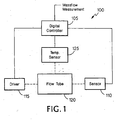•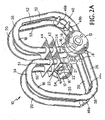•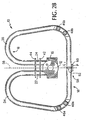•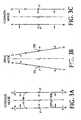•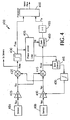•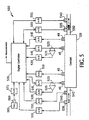•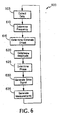•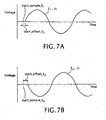•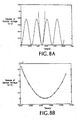•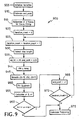•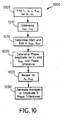•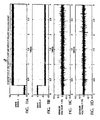•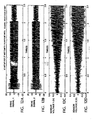•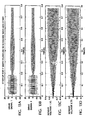•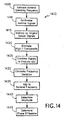•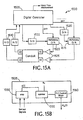•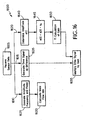•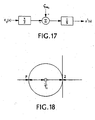•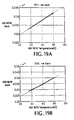•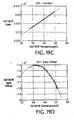•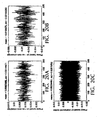•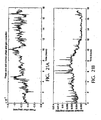•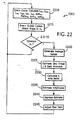•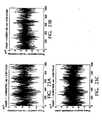•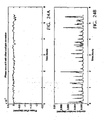•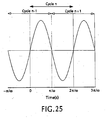•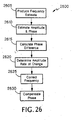•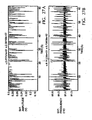•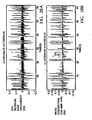•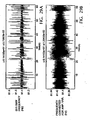•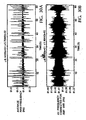•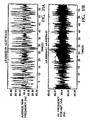•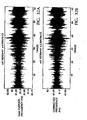•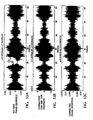•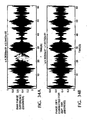•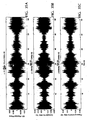•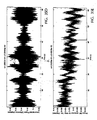•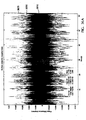•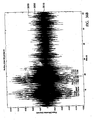•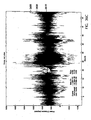•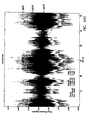•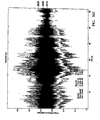•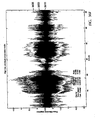•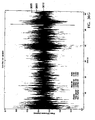••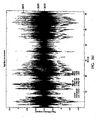•••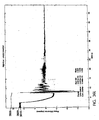•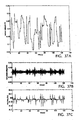•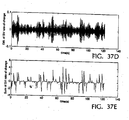•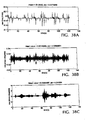•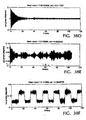•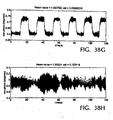•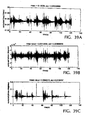••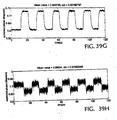•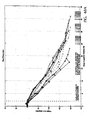•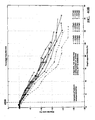••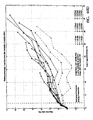••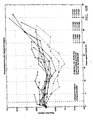•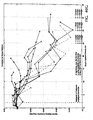••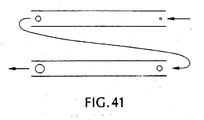•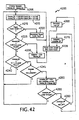•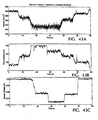••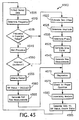•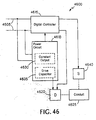•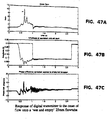•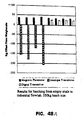•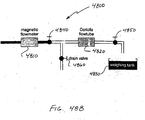•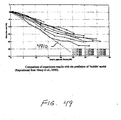••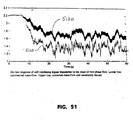•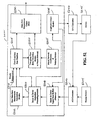•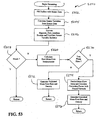•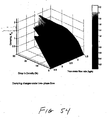•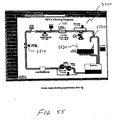•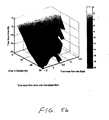•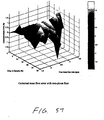•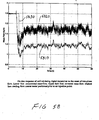## Classifications

• GPHYSICS
• G01MEASURING; TESTING
• G01FMEASURING VOLUME, VOLUME FLOW, MASS FLOW OR LIQUID LEVEL; METERING BY VOLUME
• G01F1/00Measuring the volume flow or mass flow of fluid or fluent solid material wherein the fluid passes through the meter in a continuous flow
• G01F1/76Devices for measuring mass flow of a fluid or a fluent solid material
• G01F1/78Direct mass flowmeters
• G01F1/80Direct mass flowmeters operating by measuring pressure, force, momentum, or frequency of a fluid flow to which a rotational movement has been imparted
• G01F1/84Gyroscopic mass flowmeters
• G01F1/8409Gyroscopic mass flowmeters constructional details
• G01F1/8431Gyroscopic mass flowmeters constructional details electronic circuits
• GPHYSICS
• G01MEASURING; TESTING
• G01FMEASURING VOLUME, VOLUME FLOW, MASS FLOW OR LIQUID LEVEL; METERING BY VOLUME
• G01F1/00Measuring the volume flow or mass flow of fluid or fluent solid material wherein the fluid passes through the meter in a continuous flow
• G01F1/74Devices for measuring flow of a fluid or flow of a fluent solid material in suspension in another fluid
• GPHYSICS
• G01MEASURING; TESTING
• G01FMEASURING VOLUME, VOLUME FLOW, MASS FLOW OR LIQUID LEVEL; METERING BY VOLUME
• G01F1/00Measuring the volume flow or mass flow of fluid or fluent solid material wherein the fluid passes through the meter in a continuous flow
• G01F1/76Devices for measuring mass flow of a fluid or a fluent solid material
• G01F1/78Direct mass flowmeters
• G01F1/80Direct mass flowmeters operating by measuring pressure, force, momentum, or frequency of a fluid flow to which a rotational movement has been imparted
• G01F1/84Gyroscopic mass flowmeters
• G01F1/8409Gyroscopic mass flowmeters constructional details
• G01F1/8436Gyroscopic mass flowmeters constructional details signal processing
• GPHYSICS
• G01MEASURING; TESTING
• G01FMEASURING VOLUME, VOLUME FLOW, MASS FLOW OR LIQUID LEVEL; METERING BY VOLUME
• G01F1/00Measuring the volume flow or mass flow of fluid or fluent solid material wherein the fluid passes through the meter in a continuous flow
• G01F1/76Devices for measuring mass flow of a fluid or a fluent solid material
• G01F1/78Direct mass flowmeters
• G01F1/80Direct mass flowmeters operating by measuring pressure, force, momentum, or frequency of a fluid flow to which a rotational movement has been imparted
• G01F1/84Gyroscopic mass flowmeters
• G01F1/845Gyroscopic mass flowmeters arrangements of measuring means, e.g., of measuring conduits
• G01F1/8468Gyroscopic mass flowmeters arrangements of measuring means, e.g., of measuring conduits vibrating measuring conduits
• G01F1/8481Gyroscopic mass flowmeters arrangements of measuring means, e.g., of measuring conduits vibrating measuring conduits having loop-shaped measuring conduits, e.g. the measuring conduits form a loop with a crossing point
• G01F1/8486Gyroscopic mass flowmeters arrangements of measuring means, e.g., of measuring conduits vibrating measuring conduits having loop-shaped measuring conduits, e.g. the measuring conduits form a loop with a crossing point with multiple measuring conduits
• GPHYSICS
• G01MEASURING; TESTING
• G01FMEASURING VOLUME, VOLUME FLOW, MASS FLOW OR LIQUID LEVEL; METERING BY VOLUME
• G01F15/00Details of, or accessories for, apparatus of the preceding groups insofar as such details or appliances are not adapted to particular types of such apparatus
• G01F15/02Compensating or correcting for variations in pressure, density or temperature
• G01F15/022Compensating or correcting for variations in pressure, density or temperature using electrical means
• G01F15/024Compensating or correcting for variations in pressure, density or temperature using electrical means involving digital counting

## Abstract

A flowmeter is disclosed. The flowmeter includes a vibratable conduit, and a driver connected to the conduit that is operable to impart motion to the conduit. A sensor is connected to the conduit and is operable to sense the motion of the conduit and generate a sensor signal. A controller is connected to receive the sensor signal. The controller is operable to detect a single-phase flow condition and process the sensor signal using a first process during the single-phase flow condition to generate a validated mass-flow measurement. The controller is also operable to detect a two-phase flow condition and process the sensor signal using a second process during the two-phase flow condition to generate the validated mass-flow measurement.

## Description

• The invention relates to flowmeters.
• Flowmeters provide information about materials being transferred through a conduit. For example, mass flowmeters provide a direct indication of the mass of material being transferred through a conduit. Similarly, density flowmeters, or densitometers, provide an indication of the density of material flowing through a conduit. Mass flowmeters also may provide an indication of the density of the material.
• Coriolis-type mass flowmeters are based on the well-known Coriolis effect, in which material flowing through a rotating conduit becomes a radially traveling mass that is affected by a Coriolis force and therefore experiences an acceleration. Many Coriolis-type mass flowmeters induce a Coriolis force by sinusoidally oscillating a conduit about a pivot axis orthogonal to the length of the conduit. In such mass flowmeters, the Coriolis reaction force experienced by the traveling fluid mass is transferred to the conduit itself and is manifested as a deflection or offset of the conduit in the direction of the Coriolis force vector in the plane of rotation.
• Energy is supplied to the conduit by a driving mechanism that applies a periodic force to oscillate the conduit. One type of driving mechanism is an electromechanical driver that imparts a force proportional to an applied voltage. In an oscillating flowmeter, the applied voltage is periodic, and is generally sinusoidal. The period of the input voltage is chosen so that the motion of the conduit matches a resonant mode of vibration of the conduit. This reduces the energy needed to sustain oscillation. An oscillating flowmeter may use a feedback loop in which a sensor signal that carries instantaneous frequency and phase information related to oscillation of the conduit is amplified and fed back to the conduit using the electromechanical driver.
• One prior art flowmeter, disclosed in EP 0919793 , comprises a vibratable conduit, a driver connected to the conduit and operable to impart motion to the conduit, a sensor connected to the conduit and operable to sense the motion of the conduit and generate a sensor signal, and a controller connected to receive the sensor signal, the controller being operable to generate a raw mass-flow measurement from the sensor-signal,detect-a-single-phase-flow-condition-and-process the raw mass-flow measurement using a first process during the single-phase flow condition to generate a first mass-flow measurement, and detect a two-phase flow condition using a second process during the two-phase flow condition to generate a second mass-flow measurement.
• US-A-5594180 discloses a flowmeter comprising: a vibratable conduit to receive a flow of fluid; a driver connected to the conduit and operable to impart motion to the conduit; a sensor connected to the conduit and operable to sense the motion of the conduit and generate a sensor signal; and a controller connected to receive the sensor signal, the controller being operable to: generate a raw mass-flow measurement from the sensor signal; detect a single-phase flow condition and process the raw mass-flow measurement using a first process during the single-phase flow condition to generate a first mass-flow measurement; and detect a two-phase flow condition and use a second process during the two-phase flow condition to generate a second mass-flow measurement wherein the second process includes generating an error correction factor based, at least in part, on the raw mass-flow measurement, and correcting the raw mass-flow measurement using the error-correction factor.
• The present invention provides a flowmeter which is characterised in that the controller is further operable to generate an observed density measurement from the sensor signal; and in that the error correction factor is also based on the observed density measurement.
• The second process may include a neural network processor to generate the error correction factor used to correct the raw mass-flow measurement. The neural network processor may receive at least one input parameter in addition to the raw mass flow measurement and a density drop measurement based on the observed density and apply a set of predetermined coefficients to the additional input parameter, raw mass flow measurement and density drop.
• The neural network processor may be a multi-layer perception neural network processor including an input layer for receiving the additional input parameter, the raw mass flow measurement and the density drop, a hidden layer having processing nodes for applying the set of predetermined coefficients to the additional input parameter, the raw mass flow measurement and the density drop and an output layer that generates an output parameter. The input parameter(s) may include a temperature parameter or a damping parameter. The flowmeter may further include a training module connected to the neural network processor to calculate an updated set of coefficients when supplied with training data. In other implementations of the flowmeter, the neural network processor may be a radial basis function network.
• The first mass-flow measurement may be a validated mass-flow measurement comprising the raw mass-flow measurement and an uncertainty parameter calculated by the controller. The second mass-flow measurement may be a validated mass-flow measurement comprising a corrected mass-flow measurement generated from the raw mass-flow measurement and an uncertainty parameter calculated by the controller. The controller may generate a measurement status parameter associated with the first mass-flow measurement. The controller also may generate a measurement status parameter associated with the second mass-flow measurement.
• The flowmeter may include a memory for storing sensor signal data generated from the sensor signal for use by the controller. The controller associated with the flowmeter also may include a sensor parameter processing module to analyze the sensor signal data and generate sensor signal parameters. The flowmeter also may include a state machine that uses the raw mass-flow measurement and the sensor signal parameters to detect the single-phase flow condition and the two-phase flow condition.
• The controller may include an output signal generator that is operable to receive the sensor signal parameters from the sensor parameter processing module and to generate a drive signal for the driver based the sensor signal parameters. The controller may also include circuitry to generate a drive signal based on the sensor signal. The drive signal may be a digital drive signal for operating the driver. Alternatively, the drive signal may be an analog drive signal for operating the driver.
• The flowmeter may include a second sensor connected to the conduit for sensing the motion of the conduit and generating a second sensor signal, with the controller being connected to receive the second sensor signal and generate the drive signal based on the first sensor signal and the second sensor signal using digital signal processing. The flowmeter may generate a measurement of a property of material flowing through the conduit based on the first and second sensor signals. The flowmeter may include a second driver, and the controller may generate different drive signals for the two drivers.
• The controller may generate the measurement of the property by estimating a frequency of the first sensor signal, calculating a phase difference using the first sensor signal, and generating the measurement using the calculated phase difference. The controller may compensate for amplitude differences in the sensor signals by adjusting the amplitude of one of the sensor signals. The controller may determine a frequency, amplitude and phase offsets for each sensor signal, and scale the phase offsets to an average of the frequencies of the sensor signals. The controller may calculate the phase difference using multiple approaches and selects a result of one of the approaches as the calculated based difference. The controller may combine the sensor signals to produce a combined signal and to generate the drive signal based on the combined signal. The controller may generate the drive signal by applying a gain to the combined signal.
• The controller may generate the drive signal by applying a large gain to the combined signal to initiate motion of the conduit and generate a periodic signal having a phase and frequency based on a phase and frequency of a sensor signal as the drive signal after motion has been initiated.
• The flowmeter may include a second sensor connected to the conduit and operable to sense the motion of the conduit, and the controller includes a first signal processor to generate the raw mass-flow measurement, a first analog to digital converter connected between the first sensor and the first signal processor to provide a first digital sensor signal to the first signal processor, and a second analog to digital converter connected between the second sensor and the first signal processor to provide a second digital sensor signal to the first signal processor. The first signal processor may combine the digital sensor signals to produce a combined signal and generate a gain signal based on the first and second digital sensor signals. The controller may further include a multiplying digital to analog converter connected to receive the combined signal and the gain signal from the first signal processor to generate the drive signal as a product of the combined signal and the gain signal.
• The flowmeter also may include circuitry for measuring current supplied to the driver. The controller may determine a frequency of the sensor signal by detecting zero-crossings of the sensor signal and counting samples between zero crossings. The flowmeter also may include a connection to a control system, wherein the controller transmits the measurement and results of an uncertainty analysis to the control system.
• US-A-5594180 also discloses a method of generating a mass-flow measurement of material flowing through a conduit, the method comprising: sensing motion of the conduit; generating a raw mass-flow measurement of material flowing through the conduit based on the sensed motion; detecting a flow condition based on the raw mass-flow measurement of the property; if the flow condition is a single-phase flow condition, applying a first analysis process to the raw mass-flow measurement using digital signal processing to generate a first mass-flow measurement; and if the flow condition is a two-phase flow condition, applying a second analysis process to the raw mass-flow measurement; wherein said second analysis process is applied to the raw mass-flow measurement to: predict a mass-flow error based on the raw mass-flow measurement, generate an error correction factor using the predicted mass-flow error, apply the error correction factor to the raw mass-flow measurement to generate a second mass-flow measurement signal; generate a drive signal to impart motion in the conduit based on the second mass-flow measurement signal; and impart motion to the conduit using the drive signal.
• The present invention provides a method of generating a mass-flow measurement which is characterised in that the method further includes generating an observed density measurement of material flowing through the conduit based on sensor motion; and in that the predicted mass-flow error is also based on the observed density.
• Generating the mass-flow measurement may further include storing sensor signal data in a memory. The sensor signal data may be retrieved from the memory, and sensor variables, and sensor variable statistics may be calculated from the sensor signal data. The sensor variable statistics may include mean, standard deviation, and slope for each of the sensor variables.
• The flowmeter may perform an uncertainty analysis on the first measurement signal and generate an uncertainty parameter associated with the first measurement signal to produce a validated mass-flow measurement signal. A measurement status parameter associated with the validated measurement signal may also be generated. The flowmeter may perform an uncertainty analysis on the second measurement signal and generate an uncertainty parameter associated with the second measurement signal to produce a validated mass-flow measurement signal. A measurement status parameter associated with the validated measurement signal may also be generated.
• The techniques may be implemented in a digital flowmeter, such as a digital mass flowmeter, that uses a control and measurement system to control oscillation of the conduit and to generate mass flow and density measurements. Sensors connected to the conduit supply signals to the control and measurement system. The control and measurement system processes the signals to produce a measurement of mass flow and uses digital signal processing to generate a signal for driving the conduit. The drive signal then is converted to a force that induces oscillation of the conduit.
• The digital mass flowmeter provides a number of advantages over traditional, analog approaches. For example, a processor of the flowmeter allows for the detection of a single-phase flow condition and a two-phase flow condition. The mass-flow measurement can be corrected when the flowmeter detects two-phase flow. From a control perspective, use of digital processing techniques permits the application of precise, sophisticated control algorithms that, relative to traditional analog approaches, provide greater responsiveness, accuracy and adaptability.
• The digital control system also permits the use of negative gain in controlling oscillation of the conduit. Thus, drive signals that are 180° out of phase with conduit oscillation may be used to reduce the amplitude of oscillation. The practical implications of this are important, particularly in high and variable damping situations where a sudden drop in damping can cause an undesirable increase in the amplitude of oscillation. One example of a variable damping situation is when aeration occurs in the material flowing through the conduit.
• The ability to provide negative feedback also is important when the amplitude of oscillation is controlled to a fixed setpoint that can be changed under user control. With negative feedback, reductions in the oscillation setpoint can be implemented as quickly as increases in the setpoint. By contrast, an analog meter that relies solely on positive feedback must set the gain to zero and wait for system damping to reduce the amplitude to the reduced setpoint.
• From a measurement perspective, the digital mass flowmeter can provide high information bandwidth. For example, a digital measurement system may use analog-to-digital converters operating at eighteen bits of precision and sampling rates of 55 kHz. The digital measurement system also may use sophisticated algorithms to filter and process the data, and may do so starting with the raw data from the sensors and continuing to the final measurement data. This permits extremely high precision, such as, for example, phase precision to five nanoseconds per cycle. Digital processing starting with the raw sensor data also allows for extensions to existing measurement techniques to improve performance in non-ideal situations, such as by detecting and compensating for time-varying amplitude, frequency, and zero offset.
• The control and measurement improvements interact to provide further improvements. For example, control of oscillation amplitude is dependent upon the quality of amplitude measurement. Under normal conditions, the digital mass flowmeter may maintain oscillation to within twenty parts per million of the desired setpoint. Similarly, improved control has a positive benefit on measurement. Increasing the stability of oscillation will improve measurement quality even for meters that do not require a fixed amplitude of oscillation (i.e., a fixed setpoint). For example, with improved stability, assumptions used for the measurement calculations are likely to be valid over a wider range of conditions.
• The digital mass flowmeter also permits the integration of entirely new functionality (e.g., diagnostics) with the measurement and control processes. For example, algorithms for detecting the presence of process aeration can be implemented with compensatory action occurring for both measurement and control if aeration is detected.
• Other advantages of the digital mass flowmeter result from the limited amount of hardware employed, which makes the meter simple to construct, debug, and repair in production and in the field. Quick repairs in the field for improved performance and to compensate for wear of the mechanical components (e.g, loops, flanges, sensors and drivers) are possible because the meter uses standardized hardware components that may be replaced with little difficulty, and because software modifications may be made with relative ease. In addition, integration of diagnostics, measurement, and control is simplified by the simplicity of the hardware and the level of functionality implemented in software. New functionality, such as low power components or components with improved performance, can be integrated without a major redesign of the overall control system.
• Preferred embodiments of the invention will now be described by way of example only and with reference to the accompanying drawings, in which:
• Fig. 1 is a block diagram of a digital mass flowmeter.
• Figs. 2A and 2B are perspective and side views of mechanical components of a mass flowmeter.
• Figs. 3A-3C are schematic representations of three modes of motion of the flowmeter of Fig. 1.
• Fig. 4 is a block diagram of an analog control and measurement circuit.
• Fig. 5 is a block diagram of a digital mass flowmeter.
• Fig. 6 is a flow chart showing operation of the meter of Fig. 5.
• Figs. 7A and 7B are graphs of sensor data.
• Figs. 8A and 8B are graphs of sensor voltage relative to time.
• Fig. 9 is a flow chart of a curve fitting procedure.
• Fig. 10 is a flow chart of a procedure for generating phase differences.
• Figs. 11A-11D, 12A-12D, and 13A-13D illustrate drive and sensor voltages at system startup.
• Fig. 14 is a flow chart of a procedure for measuring frequency, amplitude, and phase of sensor data using a synchronous modulation technique.
• Figs. 15A and 15B are block diagrams of a mass flowmeter.
• Fig. 16 is a flow chart of a procedure implemented by the meter of Figs. 15A and 15B.
• Fig. 17 illustrates log-amplitude control of a transfer function.
• Fig. 18 is a root locus diagram.
• Figs. 19A-19D are graphs of analog-to-digital converter performance relative to temperature.
• Figs. 20A-20C are graphs of phase measurements.
• Figs. 21A and 21B are graphs of phase measurements.
• Fig. 22 is a flow chart of a zero offset compensation procedure.
• Figs. 23A-23C, 24A, and 24B are graphs of phase measurements.
• Fig. 25 is a graph of sensor voltage.
• Fig. 26 is a flow chart of a procedure for compensating for dynamic effects.
• Figs. 27A-35E are graphs illustrating application of the procedure of Fig. 29.
• Figs. 36A-36L are graphs illustrating phase measurement.
• Fig. 37A is a graph of sensor voltages.
• Figs. 37B and 37C are graphs of phase and frequency measurements corresponding to the sensor voltages of Fig. 37A.
• Figs. 37D and 37E are graphs of correction parameters for the phase and frequency measurements of Figs. 37B and 37C.
• Figs. 38A-38H are graphs of raw measurements.
• Figs. 39A-39H are graphs of corrected measurements.
• Figs. 40A-40H are graphs illustrating correction for aeration.
• Fig. 41 is a block diagram illustrating the effect of aeration in a conduit.
• Fig. 42 is a flow chart of a setpoint control procedure.
• Figs. 43A-43C are graphs illustrating application of the procedure of Fig. 41.
• Fig. 44 is a graph comparing the performance of digital and analog flowmeters.
• Fig. 45 is a flow chart showing operation of a self-validating meter.
• Fig. 46 is a block diagram of a two-wire digital mass flowmeter.
• Figs. 47A-47C are graphs showing response of the digital mass flowmeter under wet and empty conditions.
• Fig. 48A is a chart showing results for batching from empty trials.
• Fig. 48B is a diagram showing an experimental flow rig.
• Figs49 is a graph showing mass-flow errors against drop in apparent density.
• Fig. 50 is a graph showing residual mass-flow errors after applying corrections.
• Fig. 51 is a graph showing on-line response of the self-validating digital mass flowmeter to the onset of two-phase flow.
• Fig. 52 is a block diagram of a digital controller implementing a neural network processor that may be used with the digital mass flowmeter.
• Fig. 53 is a flow diagram showing the technique for implementing the neural network to predict the mass-flow error and generate an error correction factor to correct the mass-flow measurement signal when two-phase flow is detected.
• Fig. 54 is a 3D graph showing damping changes under two-phase flow conditions.
• Fig. 55 is a flow diagram illustrating the experimental flow rig.
• Fig. 56 is a 3D graph showing true mass flow error under two-phase flow conditions.
• Fig. 57 is a 3D graph showing corrected mass flow error under two-phase flow conditions.
• Fig. 58 is a graph comparing the uncorrected mass flow measurement signal with the neural network corrected mass flow measurement signal.
• Techniques are provided for accounting for the effects of two-phase flow-in, for example, a digital flowmeter. Before the techniques are described starting with reference to Fig. 40, digital flowmeters are discussed with reference to Figs. 1-39.
• Referring to Fig. 1, a digital mass flowmeter 100 includes a digital controller 105, one or more motion sensors 110, one or more drivers 115, a conduit 120 (also referred to as a flowtube), and a temperature sensor 125. The digital controller 105 may be implemented using one or more of, for example, a processor, a field-programmable gate array, an ASIC, other programmable logic or gate arrays, or programmable logic with a processor core. The digital controller generates a measurement of mass flow through the conduit 120 based at least on signals received from the motion sensors 110. The digital controller also controls the drivers 115 to induce motion in the conduit 120. This motion is sensed by the motion sensors 110.
• Mass flow through the conduit 120 is related to the motion induced in the conduit in response to a driving force supplied by the drivers 115. In particular, mass flow is related to the phase and frequency of the motion, as well as to the temperature of the conduit. The digital mass flowmeter also may provide a measurement of the density of material flowing through the conduit. The density is related to the frequency of the motion and the temperature of the conduit. Many of the described techniques are applicable to a densitometer that provides a measure of density rather than a measure of mass flow.
• The temperature in the conduit, which is measured using the temperature sensor 125, affects certain properties of the conduit, such as its stiffness and dimensions. The digital controller compensates for these temperature effects. The temperature of the digital controller 105 affects, for example, the operating frequency of the digital controller. In general, the effects of controller temperature are sufficiently small to be considered negligible. However, in some instances, the digital controller may measure the controller temperature using a solid state device and may compensate for effects of the controller temperature.
• A. Mechanical Design
• In one implementation, as illustrated in Figs. 2A and 2B, the conduit 120 is designed to be inserted in a pipeline (not shown) having a small section removed or reserved to make room for the conduit. The conduit 120 includes mounting flanges 12 for connection to the pipeline, and a central manifold block 16 supporting two parallel planar loops 18 and 20 that are oriented perpendicularly to the pipeline. An electromagnetic driver 46 and a sensor 48 are attached between each end of loops 18 and 20. Each of the two drivers 46 corresponds to a driver 115 of Fig. 1, while each of the two sensors 48 corresponds to a sensor 110 of Fig. 1.
• The drivers 46 on opposite ends of the loops are energized with current of equal magnitude but opposite sign (i.e., currents that are 180° out-of-phase) to cause straight sections 26 of the loops 18, 20 to rotate about their co-planar perpendicular bisector 56, which intersects the tube at point P (Fig. 2B). Repeatedly reversing (e.g., controlling sinusoidally) the energizing current supplied to the drivers causes each straight section 26 to undergo oscillatory motion that sweeps out a bow tie shape in the horizontal plane about line 56-56, the axis of symmetry of the loop. The entire lateral excursion of the loops at the lower rounded turns 38 and 40 is small, on the order of 0.0016 m (1/16 of an inch) for a 0.6 m (two foot) long straight section 26 of a pipe having a 0.025 m (one inch) diameter. The frequency of oscillation is typically about 80 to 90 Hertz.
• B. Conduit Motion
• The motion of the straight sections of loops 18 and 20 are shown in three modes in Figs. 3A, 3B and 3C. In the drive mode shown in Fig. 3B, the loops are driven 180° out-of-phase about their respective points P so that the two loops rotate synchronously but in the opposite sense. Consequently, respective ends such as A and C periodically come together and go apart.
• The drive motion shown in Fig. 3B induces the Coriolis mode motion shown in Fig. 3A, which is in opposite directions between the loops and moves the straight sections 26 slightly toward (or away) from each other. The Coriolis effect is directly related to mvW, where m is the mass of material in a cross section of a loop, v is the velocity at which the mass is moving (the volumetric flow rate), W is the angular velocity of the loop (W = Wosinωt), and mv is the mass flow rate. The Coriolis effect is greates when the two straight sections are driven sinusoidally and have a sinusoidally varying angular velocity. Under these conditions, the Coriolis effect is 90° out-of-phase with the drive signal.
• Fig. 3C shows an undesirable common mode motion that deflects the loops in the same direction. This type of motion might be produced by an axial vibration in the pipeline in the embodiment of Figs. 2A and 2B because the loops are perpendicular to the pipeline.
• The type of oscillation shown in Fig. 3B is called the antisymmetrical mode, and the Coriolis mode of Fig. 3A is called the symmetrical mode. The natural frequency of oscillation in the antisymmetrical mode is a function of the torsional resilience of the legs. Ordinarily the resonant frequency of the antisymmetrical mode for conduits of the shape shown in Figs. 2A and 2B is higher than the resonant frequency of the symmetrical mode. To reduce the noise sensitivity of the mass flow measurement, it is desirable to maximize the Coriolis force for a given mass flow rate. As noted above, the loops are driven at their resonant frequency, and the Coriolis force is directly related to the frequency at which the loops are oscillating (i.e., the angular velocity of the loops). Accordingly, the loops are driven in the antisymmetrical mode, which tends to have the higher resonant frequency.
• Other implementations may include different conduit designs. For example, a single loop or a straight tube section may be employed as the conduit.
• C. Electronic Design
• The digital controller 105 determines the mass flow rate by processing signals produced by the sensors 48 (i.e., the motion sensors 110) located at opposite ends of the loops. The signal produced by each sensor includes a component corresponding to the relative velocity at which the loops are driven by a driver positioned next to the sensor and a component corresponding to the relative velocity of the loops due to Coriolis forces induced in the loops. The loops are driven in the antisymmetrical mode, so that the components of the sensor signals corresponding to drive velocity are equal in magnitude but opposite in sign. The resulting Coriolis force is in the symmetrical mode so that the components of the sensor signals corresponding to Coriolis velocity are equal in magnitude and sign. Thus, differencing the signals cancels out the Coriolis velocity components and results in a difference that is proportional to the drive velocity. Similarly, summing the signals cancels out the drive velocity components and results in a sum that is proportional to the Coriolis velocity, which, in turn, is proportional to the Coriolis force. This sum then may be used to determine the mass flow rate.
• 1. Analog Control System
• The digital mass flowmeter 100 provides considerable advantages over traditional, analog mass flowmeters. For use in later discussion, Fig. 4 illustrates an analog control system 400 of a traditional mass flowmeter. The sensors 48 each produce a voltage signal, with signal VA0 being produced by sensor 48a.and signal VB0 being produced by sensor 48b. VA0 and VB0 correspond to the velocity of the loops relative to each other at the positions of the sensors. Prior to processing, signals VA0 and VB0 are amplified at respective input amplifiers 405 and 410 to produce signals VA1 and VB1. To correct for imbalances in the amplifiers and the sensors, input amplifier 410 has a variable gain that is controlled by a balance signal coming from a feedback loop that contains a synchronous demodulator 415 and an integrator 420.
• At the output of amplifier 405, signal VA1 is of the form: $V A ⁢ 1 = V D sin ωt + V C cos ωt ,$ and, at the output of amplifier 410, signal VB1 is of the form: $V B ⁢ 1 = - V D sin ωt + V C cos ωt ,$ where VD and VC are, respectively, the drive voltage and the Coriolis voltage, and ω is the drive mode angular frequency.
• Voltages VA1 and VB1 are differenced by operational amplifier 425 to produce: $V DRV = V A ⁢ 1 - V B ⁢ 1 = 2 ⁢ V D sin ωt ,$ where VDRV corresponds to the drive motion and is used to power the drivers. In addition to powering the drivers, VDRV is supplied to a positive going zero crossing detector 430 that produces an output square wave FDRV having a frequency corresponding to that of VDRV (ω = 2πFDRV). FDRV is used as the input to a digital phase locked loop circuit 435. FDRV also is supplied to a processor 440.
• Voltages VA1 and VB1 are summed by operational amplifier 445 to produce: $V COR = V A ⁢ 1 + V B ⁢ 1 = 2 ⁢ V C cos ωt ,$ where VCOR is related to the induced Coriolis motion.
• VCOR is supplied to a synchronous demodulator 450 that produces an output voltage VM that is directly proportional to mass by rejecting the components of VCOR that do not have the same frequency as, and are not in phase with, a gating signal Q. The phase locked loop circuit 43S produces Q, which is a quadrature reference signal that has the same frequency (ω) as VDRV and is 90° out of phase with VDRV (i.e., in phase with VCOR). Accordingly, synchronous demodulator 450 rejects frequencies other than ω so that VM corresponds to the amplitude of VCOR at ω. This amplitude is directly proportional to the mass in the conduit.
• VM is supplied to a voltage-to-frequency converter 455 that produces a square wave signal FM having a frequency that corresponds to the amplitude of VM. The processor 440 then divides FM by FDRV to produce a measurement of the mass flow rate.
• Digital phase locked loop circuit 435 also produces a reference signal I that is in phase with VDRV and is used to gate the synchronous demodulator 415 in the feedback loop controlling amplifier 410. When the gains of the input amplifiers 405 and 410 multiplied by the drive components of the corresponding input signals are equal, the summing operation at operational amplifier 445 produces zero drive component (i.e., no signal in phase with VDRV) in the signal VCOR. When the gains of the input amplifiers 405 and 410 are not equal, a drive component exists in VCOR. This drive component is extracted by synchronous demodulator 415 and integrated by integrator 420 to generate an error voltage that corrects the gain of input amplifier 410. When the gain is too high or too low, the synchronous demodulator 415 produces an output voltage that causes the integrator to change the error voltage that modifies the gain. When the gain reaches the desired value, the output of the synchronous modulator goes to zero and the error voltage stops changing to maintain the gain at the desired value.
• 2. Digital Control System
• Fig. 5 provides a block diagram of an implementation 500 of the digital mass flowmeter 100 that includes the conduit 120, drivers 46, and sensors 48 of Figs. 2A and 2B, along with a digital controller 505. Analog signals from the sensors 48 are converted to digital signals by analog-to-digital ("A/D") converters 510 and supplied to the controller 505. The A/D converters may be implemented as separate converters, or as separate channels of a single converter.
• Digital-to-analog ("D/A") converters 515 convert digital control signals from the controller 505 to analog signals for driving the drivers 46. The use of a separate drive signal for each driver has a number of advantages. For example, the system may easily switch between symmetrical and antisymmetrical drive modes for diagnostic purposes. In other implementations, the signals produced by converters 515 may be amplified by amplifiers prior to being supplied to the drivers 46. In still other implementations, a single D/A converter may be used to produce a drive signal applied to both drivers, with the drive signal being inverted prior to being provided to one of the drivers to drive the conduit 120 in the antisymmetrical mode.
• High precision resistors 520 and amplifiers 525 are used to measure the current supplied to each driver 46. A/D converters 530 convert the measured current to digital signals and supply the digital signals to controller 505. The controller 505 uses the measured currents in generating the driving signals.
• Temperature sensors 535 and pressure sensors 540 measure, respectively, the temperature and the pressure at the inlet 545 and the outlet 550 of the conduit. A/D converters 555 convert the measured values to digital signals and supply the digital signals to the controller 505. The controller 505 uses the measured values in a number of ways. For example, the difference between the pressure measurements may be used to determine a back pressure in the conduit. Since the stiffness of the conduit varies with the back pressure, the controller may account for conduit stiffness based on the determined back pressure.
• An additional temperature sensor 560 measures the temperature of the crystal oscillator 565 used by the A/D converters. An A/D converter 570 converts this temperature measurement to a digital signal for use by the controller 505. The input/output relationship of the A/D converters varies with the operating frequency of the converters, and the operating frequency varies with the temperature of the crystal oscillator. Accordingly, the controller uses the temperature measurement to adjust the data provided by the A/D converters, or in system calibration.
• In the implementation of Fig. 5, the digital controller 505 processes the digitized sensor signals produced by the A/D converters 510 according to the procedure 600 illustrated in Fig. 6 to generate the mass flow measurement and the drive signal supplied to the drivers 46. Initially, the controller collects data from the sensors (step 605). Using this data, the controller determines the frequency of the sensor signals (step 610), eliminates zero offset from the sensor signals (step 615), and determines the amplitude (step 620) and phase (step 625) of the sensor signals. The controller uses these calculated values to generate the drive signal (step 630) and to generate the mass flow and other measurements (step 635). After generating the drive signals and measurements, the controller collects a new set of data and repeats the procedure. The steps of the procedure 600 may be performed serially or in parallel, and may be performed in varying order.
• Because of the relationships between frequency, zero offset, amplitude, and phase, an estimate of one may be used in calculating another. This leads to repeated calculations to improve accuracy. For example, an initial frequency determination used in determining the zero offset in the sensor signals may be revised using offset-eliminated sensor signals. In addition, where appropriate, values generated for a cycle may be used as starting estimates for a following cycle.
• a. Data Collection
• For ease of discussion, the digitized signals from the two sensors will be referred to as signals SV1 and SV2, with signal SV1 coming from sensor 48a and signal SV2 coming from sensor 48b. Although new data is generated constantly, it is assumed that calculations are based upon data corresponding to one complete cycle of both sensors. With sufficient data buffering, this condition will be true so long as the average time to process data is less than the time taken to collect the data. Tasks to be carried out for a cycle include deciding that the cycle has been completed, calculating the frequency of the cycle (or the frequencies of SV1 and SV2), calculating the amplitudes of SV1 and SV2, and calculating the phase difference between SV1 and SV2. In some implementations, these calculations are repeated for each cycle using the end point of the previous cycle as the start for the next. In other implementations, the cycles overlap by 180° or other amounts (e.g., 90°) so that a cycle is subsumed within the cycles that precede and follow it.
• Figs. 7A and 7B illustrate two vectors of sampled data from signals SV1 and SV2, which are named, respectively, sv1_in and sv2_in. The first sampling point of each vector is known, and corresponds to a zero crossing of the sine wave represented by the vector. For svl_in, the first sampling point is the zero crossing from a negative value to a positive value, while for sv2_in the first sampling point is the zero crossing from a positive value to a negative value.
• An actual starting point for a cycle (i.e., the actual zero crossing) will rarely coincide exactly with a sampling point. For this reason, the initial sampling points (start_sample_SV1 and start_sample_SV2) are the sampling points occurring just before the start of the cycle. To account for the difference between the first sampling point and the actual start of the cycle, the approach also uses the position (start_offset_SV1 or start_offset_SV2) between the starting sample and the next sample at which the cycle actually begins.
• Since there is a phase offset between signals SV1 and SV2, sv1_in and sv2_in may start at different sampling points. If both the sample rate and the phase difference are high, there may be a difference of several samples between the start of sv1_in and the start of sv2_in. This difference provides a crude estimate of the phase offset, and may be used as a check on the calculated phase offset, which is discussed below. For example, when sampling at 55 kHz, one sample corresponds to approximately 0.5 degrees of phase shift, and one cycle corresponds to about 800 sample points.
• When the controller employs functions such as the sum (A+B) and difference (A-B), with B weighted to have the same amplitude as A, additional variables (e.g., start_sample_sum and start_offset_sum) track the start of the period for each function. The sum and difference functions have a phase offset halfway between SV1 and SV2.
• In one implementation, the data structure employed to store the data from the sensors is a circular list for each sensor, with a capacity of at least twice the maximum number of samples in a cycle. With this data structure, processing may be carried out on data for a current cycle while interrupts or other techniques are used to add data for a following cycle to the lists.
• Processing is performed on data corresponding to a full cycle to avoid errors when employing approaches based on sine-waves. Accordingly, the first task in assembling data for a cycle is to determine where the cycle begins and ends. When nonoverlapping cycles are employed, the beginning of the cycle may be identified as the end of the previous cycle. When overlapping cycles are employed, and the cycles overlap by 180°, the beginning of the cycle may be identified as the midpoint of the previous cycle, or as the endpoint of the cycle preceding the previous cycle.
• The end of the cycle may be first estimated based on the parameters of the previous cycle and under the assumption that the parameters will not change by more than a predetermined amount from cycle to cycle. For example, five percent may be used as the maximum permitted change from the last cycle's value, which is reasonable since, at sampling rates of 55 kHz, repeated increases or decreases of five percent in amplitude or frequency over consecutive cycles would result in changes of close to 5,000 percent in one second.
• By designating five percent as the maximum permissible increase in amplitude and frequency, and allowing for a maximum phase change of 5° in consecutive cycles, a conservative estimate for the upper limit on the end of the cycle for signal SV1 may be determined as: $end_sample_SV ⁢ 1 ≤ start_sample_SV ⁢ 1 + 365 360 * sample_rate est_freq * 0.95$ where start_sample_SV1 is the first sample of sv1_in, sample_rate is the sampling rate, and est_freq is the frequency from the previous cycle. The upper limit on the end of the cycle for signal SV2 (end_sample_SV2) may be determined similarly.
• After the end of a cycle is identified, simple checks may be made as to whether the cycle is worth processing. A cycle may not be worth processing when, for example, the conduit has stalled or the sensor waveforms are severely distorted. Processing only suitable cycles provides considerable reductions in computation.
• One way to determine cycle suitability is to examine certain points of a cycle to confirm expected behavior. As noted above, the amplitudes and frequency of the last cycle give useful starting estimates of the corresponding values for the current cycle. Using these values, the points corresponding to 30°, 150°, 210° and 330° of the cycle may be examined. If the amplitude and frequency were to match exactly the amplitude and frequency for the previous cycle, these points should have values corresponding to est_amp/2, est_amp/2,
-est_amp/2, and -est_amp/2, respectively, where est_amp is the estimated amplitude of a signal (i.e., the amplitude from the previous cycle). Allowing for a five percent change in both amplitude and frequency, inequalities may be generated for each quarter cycle. For the 30° point, the inequality is $sv ⁢ 1 _in start_sample_SV ⁢ 1 + 30 360 * sample_rate est_freq * 1.05 > 0.475 * est_amp_SV$
The inequalities for the other points have the same for, with the degree offset term (x/360) and the sign of the est_amp_SV1 term having appropriate values. These inequalities can be used to check that the conduit has vibrated in a reasonable manner.
• Measurement processing takes place on the vectors sv1_in(start:end) and sv2_in(start:end) where: $start = min start_sample_SV ⁢ 1 , start_sample_SV ⁢ 2 ,$ $end = max end_sample_SV ⁢ 1 , end_sample_SV ⁢ 2 .$
The difference between the start and end points for a signal is indicative of the frequency of the signal.
• b. Frequency Determination
• The frequency of a discretely-sampled pure sine wave may be calculated by detecting the transition between periods (i.e., by detecting positive or negative zero-crossings) and counting the number of samples in each period. Using this method, sampling, for example, an 82.2 Hz sine wave at 55 kHz will provide an estimate of frequency with a maximum error of 0.15 percent. Greater accuracy may be achieved by estimating the fractional part of a sample at which the zero-crossing actually occurred using, for example, start_offset_SV1 and start_offset_SV2. Random noise and zero offset may reduce the accuracy of this approach.
• As illustrated in Figs. 8A and 8B, a more refined method of frequency determination uses quadratic interpolation of the square of the sine wave. With this method, the square of the sine wave is calculated, a quadratic function is fitted to match the minimum point of the squared sine wave, and the zeros of the quadratic function are used to determine the frequency. If $sv t = A sin x t + δ + σε t ,$ where svt is the sensor voltage at time t, A is the amplitude of oscillation, xt is the radian angle at time t (i.e., xt = 2πft), δ is the zero offset, εt is a random variable with distribution N(0,1), and σ is the variance of the noise, then the squared function is given by: $sv t 2 = A 2 ⁢ sin 2 ⁢ x t + 2 ⁢ A ⁢ δ + σε t ⁢ sin x t + 2 ⁢ δ ⁢ σε t + δ 2 + σ 2 + ε t 2 .$ When xt is close to 2π, sin Xt and sin2xt can be approximated as X0t = Xt-2π and X0t 2, respectively. Accordingly, for values of xt close to 2π, at can be approximated as: $a t 2 ≈ A 2 ⁢ x 0 ⁢ t 2 + 2 ⁢ A ⁢ δ + σε t ⁢ x 0 ⁢ t + 2 ⁢ δ ⁢ σε t + δ 2 + σ 2 ⁢ ε t 2 ≈ A 2 ⁢ x 0 ⁢ t 2 + 2 ⁢ Aδ ⁢ x 0 ⁢ t + δ 2 + σε t ⁢ 2 ⁢ A ⁢ x 0 ⁢ t + 2 ⁢ δ + σε t .$ This is a pure quadratic (with a non-zero minimum, assuming δ=0) plus noise, with the amplitude of the noise being dependent upon both σ and δ. Linear interpolation also could be used.
• Error sources associated with this curve fitting technique are random noise, zero offset, and deviation from a true quadratic. Curve fitting is highly sensitive to the level of random noise. Zero offset in the sensor voltage increases the amplitude of noise in the sine-squared function, and illustrates the importance of zero offset elimination (discussed below). Moving away from the minimum, the square of even a pure sine wave is not entirely quadratic. The most significant extra term is of fourth order. By contrast, the most significant extra term for linear interpolation is of third order.
• Degrees of freedom associated with this curve fitting technique are related to how many, and which, data points are used. The minimum is three, but more may be used (at greater computational expense) by using least-squares fitting. Such a fit is less susceptible to random noise. Fig. 8A illustrates that a quadratic approximation is good up to some 20° away from the minimum point. Using data points further away from the minimum will reduce the influence of random noise, but will increase the errors due to the non-quadratic terms (i.e., fourth order and higher) in the sine-squared function.
• Fig. 9 illustrates a procedure 900 for performing the curve fitting technique. As a first step, the controller initializes variables (step 905). These variables include end_point, the best estimate of the zero crossing point; ep_int, the integer value nearest to end_point; s[0..i], the set of all sample points; z[k], the square of the sample point closest to end_point; z[0..n-1], a set of squared sample points used to calculate end_point; n, the number of sample points used to calculate end_point (n = 2k + 1); step_length, the number of samples in s between consecutive values in z; and iteration_count, a count of the iterations that the controller has performed.
• The controller then generates a first estimate of end_point (step 910). The controller generates this estimate by calculating an estimated zero-crossing point based on the estimated frequency from the previous cycle and searching around the estimated crossing point (forwards and backwards) to find the nearest true crossing point (i.e., the occurrence of consecutive samples with different signs). The controller then sets end_point equal to the sample point having the smaller magnitude of the samples surrounding the true crossing point.
• Next, the controller sets n, the number of points for curve fitting (step 915). The controller sets n equal to 5 for a sample rate of 11 kHz, and to 21 for a sample rate of 44 kHz. The controller then sets iteration_count to 0 (step 920) and increments iteration_count (step 925) to begin the iterative portion of the procedure.
• As a first step in the iterative portion of the procedure, the controller selects step_length (step 930) based on the value of iteration count. The controller sets step length equal to 6, 3, or 1 depending on whether iteration_count equals, respectively, 1,2 or 3.
• Next, the controller determines ep_int as the integer portion of the sum of end_point and 0.5 (step 935) and fills the z array (step 940). For example, when n equals 5, z = s[ep_int-2*step_length]2, z = s[ep_int-step_length]2, z = s[ep_int]2, z = s[ep_int+step_length)2, and z = s[ep_int+2*step_length]2.
• Next, the controller uses a filter, such as a Savitzky-Golay filter, to calculate smoothed values of z[k-1], z[k] and z[k+1] (step 945). Savitzky-Golay smoothing filters are discussed by Press et al. in Numerical Recipes in C, pp. 650-655 (2nd ed., Cambridge University Press, 1995), which is incorporated by reference. The controller then fits a quadratic to z[k-1], z[k] and z[k+l] (step 950), and calculates the minimum value of the quadratic (z*) and the corresponding position (x*) (step 955).
• If x* is between the points corresponding to k-1 and k+1 (step 960), then the controller sets end_point equal to x* (step 965). Thereafter, if iteration_count is less than 3 (step 970), the controller increments iteration_count (step 925) and repeats the iterative portion of the procedure.
• If x* is not between the points corresponding to k-1 and k+1 (step 960), or if iteration_count equals 3 (step 970), the controller exits the iterative portion of the procedure. The controller then calculates the frequency based on the difference between end_point and the starting point for the cycle, which is known (step 975).
• In essence, the procedure 900 causes the controller to make three attempts to home in on end_point, using smaller step_lengths in each attempt. If the resulting minimum for any attempt falls outside of the points used to fit the curve (i.e., there has been extrapolation rather than interpolation), this indicates that either the previous or new estimate is poor, and that a reduction in step size is unwarranted.
• The procedure 900 may be applied to at least three different sine waves produced by the sensors. These include signals SV1and SV2 and the weighted sum of the two. Moreover, assuming that zero offset is eliminated, the frequency estimates produced for these signals are independent. This is clearly true for signals SV1 and SV2, as the errors on each are independent. It is also true, however, for the weighted sum, as long as the mass flow and the corresponding phase difference between signals SV1 and SV2 are large enough for the calculation of frequency to be based on different samples in each case. When this is true, the random errors in the frequency estimates also should be independent.
• The three independent estimates of frequency can be combined to provide an improved estimate. This combined estimate is simply the mean of the three frequency estimates.
• c. Zero Offset Compensation
• An important error source in a Coriolis transmitter is zero offset in each of the sensor voltages. Zero offset is introduced into a sensor voltage signal by drift in the pre-amplification circuitry and the analog-to-digital converter. The zero offset effect may be worsened by slight differences in the pre-amplification gains for positive and negative voltages due to the use of differential circuitry. Each error source varies between transmitters, and will vary-with transmitter temperature and more generally over time with component wear.
• An example of the zero offset compensation technique employed by the controller is discussed in detail below. In general, the controller uses the frequency estimate and an integration technique to determine the zero offset in each of the sensor signals. The controller then eliminates the zero offset from those signals. After eliminating zero offset from signals SV1 and SV2, the controller may recalculate the frequency of those signals to provide an improved estimate of the frequency.
• d. Amplitude Determination
• The amplitude of oscillation has a variety of potential uses. These include regulating conduit oscillation via feedback, balancing contributions of sensor voltages when synthesizing driver waveforms, calculating sums and differences for phase measurement, and calculating an amplitude rate of change for measurement correction purposes.
• In one implementation, the controller uses the estimated amplitudes of signals SV1 and SV2 to calculate the sum and difference of signals SV1 and SV2, and the product of the sum and difference. Prior to determining the sum and difference, the controller compensates one of the signals to account for differences between the gains of the two sensors. For example, the controller may compensate the data for signal SV2 based on the ratio of the amplitude of signal SV1 to the amplitude of signal SV2 so that both signals have the same amplitude.
• The controller may produce an additional estimate of the frequency based on the calculated sum. This estimate may be averaged with previous frequency estimates to produce a refined estimate of the frequency of the signals, or may replace the previous estimates.
• The controller may calculate the amplitude according to a Fourier-based technique to eliminate the effects of higher harmonics. A sensor voltage x(t) over a period T (as identified using zero crossing techniques) can be represented by an offset and a series of harmonic terms as: $x t = a 0 / 2 + a 1 ⁢ cos ωt + a 2 ⁢ cos 2 ⁢ ωt + a 3 ⁢ cos 3 ⁢ ωt + … + b 1 ⁢ sin ωt + b 2 ⁢ sin 2 ⁢ ωt + …$
• With this representation, a non-zero offset a0 will result in non-zero cosine terms an. Though the amplitude of interest is the amplitude of the fundamental component (i.e., the amplitude at frequency ω), monitoring the amplitudes of higher harmonic components (i.e., at frequencies kω, where k is greater than 1) may be of value for diagnostic purposes. The values of an and bn may be calculated as: $a n = 2 T ⁢ ∫ 0 T ⁢ x t ⁢ cos n ⁢ ω ⅆ t ,$ and $b n = 2 T ⁢ ∫ 0 T ⁢ x t ⁢ sin n ⁢ ω ⅆ t .$ The amplitude, An, of each harmonic is given by: $A n = a n 2 + b n 2 .$ The integrals are calculated using Simpson's method with quadratic correction (described below). The chief computational expense of the method is calculating the pure sine and cosine functions.
• e. Phase Determination
• The controller may use a number of approaches to calculate the phase difference between signals SV1 and SV2. For example, the controller may determine the phase offset of each harmonic, relative to the starting time at t=0, as: $ϕ n = tan - 1 ⁢ a n b n .$ The phase offset is interpreted in the context of a single waveform as being the difference between the start of the cycle (i.e., the zero-crossing point) and the point of zero phase for the component of SV(t) of frequency ω. Since the phase offsets is an average over the entire waveform, it may be used as the phase offset from the midpoint of the cycle. Ideally, with no zero offset and constant amplitude of oscillation, the phase offset should be zero every cycle. The controller may determine the phase difference by comparing the phase offset of each sensor voltage over the same time period.
• The amplitude and phase may be generated using a Fourier method that eliminates the effects of higher harmonics. This method has the advantage that it does not assume that both ends of the conduits are oscillating at the same frequency. As a first step in the method, a frequency estimate is produced using the zero crossings to measure the time between the start and end of the cycle. If linear variation in frequency is assumed, this estimate equals the time-averaged frequency over the periods. Using the estimated, and assumed time-invariant, frequency ω of the cycle, the controller calculates: $I 1 = 2 ⁢ ω π ⁢ ∫ 0 2 ⁢ π ω ⁢ SV t ⁢ sin ω ⁢ t ⁢ dt ,$ and $I 2 = 2 ⁢ ω π ⁢ ∫ 0 2 ⁢ π ω ⁢ SV t ⁢ cos ω ⁢ t ⁢ dt ,$ where SV(t) is the sensor voltage waveform (i.e., SV1(T) or SV2(t)). The controller then determines the estimates of the amplitude and phase: $Amp = I 1 2 + I 2 2 ,$ and $Phase = tan - 1 ⁢ I 2 I 1 .$
• The controller then calculates a phase difference, assuming that the average phase and frequency of each sensor signal is representative of the entire waveform. Since these frequencies are different for SV1 and SV2, the corresponding phases are scaled to the average frequency. In addition, the phases are shifted to the same starting point (i.e., the midpoint of the cycle on SV1). After scaling, they are subtracted to provide the phase difference: $scaled_phase_SV 1 = phase_SV 1 ⁢ av_freq freq_SV 1 ,$ $scaled_shift_SV 2 = midpoint_SV 2 - midpoint_SV 1 ⁢ h freq_SV 2 360 ,$ and $scaled_phase_SV 2 = phase_SV 2 + scaled_shift_SV 2 ⁢ av_freq freq_SV 2 ,$ where h is the sample length and the midpoints are defined in terms of samples: $midpoint_SV x = startpoint_SV x + endpoint_SV x 2$
• In general, phase and amplitude are not calculated over the same time-frame for the two sensors. When the flow rate is zero, the two cycle mid-points are coincident. However, they diverge at high flow rates so that the calculations are based on sample sets that are not coincident in time. This leads to increased phase noise in conditions of changing mass flow. At full flow rate, a phase shift of 4° (out of 360°) means that only 99% of the samples in the SV1and SV2 data sets are coincident. Far greater phase shifts may be observed under aerated conditions, which may lead to even lower rates of overlap.
• Fig. 10 illustrates a modified approach 1000 that addresses this issue. First, the controller finds the frequencies (f1, f2) and the mid-points (m1, m2) of the SV1 and SV2 data sets (d1, d2) (step 1005). Assuming linear shift in frequency from the last cycle, the controller calculates the frequency of SV2 at the midpoint of SV1 (f2m1) and the frequency of SV1 at the midpoint of SV2 (f1m2) (step 1010).
• The controller then calculates the starting and ending points of new data sets (d1m2 and d2m1) with mid-points m2 and m1 respectively, and assuming frequencies of f1m2 and f2m1 (step 1015). These end points do not necessarily coincide with zero crossing points. However, this is not a requirement for Fourier-based calculations.
• The controller then carries out the Fourier calculations of phase and amplitude on the sets d1 and d2m1, and the phase difference calculations outlined above (step 1020). Since the mid-points of d1 and d2m1 are identical, scale-shift_SV2 is always zero and can be ignored. The controller repeats these calculations for the data sets d2 and d1m2 (step 1025). The controller then generates averages of the calculated amplitude and phase difference for use in measurement generation (step 1030). When there is sufficient separation between the mid points m1 and m2, the controller also may use the two sets of results to provide local estimates of the rates of change of phase and amplitude.
• The controller also may use a difference-amplitude method that involves calculating a difference between SV1 and SV2, squaring the calculated difference, and integrating the result. According to another approach, the controller synthesizes a sine wave, multiplies the sine wave by the difference between signals SV1 and SV2, and integrates the result. The controller also may integrate the product of signals SV1 and SV2, which is a sine wave having a frequency 2f (where f is the average frequency of signals SV1 and SV2), or may square the product and integrate the result. The controller also may synthesize a cosine wave comparable to the product sine wave and multiply the synthesized cosine wave by the product sine wave to produce a sine wave of frequency 4f that the controller then integrates. The controller also may use multiple ones of these approaches to produce separate phase measurements, and then may calculate a mean value of the separate measurements as the final phase measurement.
• The difference-amplitude method starts with: $SV 1 t = A 1 sin 2 ⁢ π ⁢ ft + ϕ 2 and SV 2 t = A 2 sin 2 ⁢ π ⁢ ft - ϕ 2 ,$ where ϕ is the phase difference between the sensors. Basic trigonometric identities may be used to define the sum (Sum) and difference (Diff) between the signals as: $Sum ≡ SV 1 t + A 1 A 2 ⁢ SV 2 t = 2 ⁢ A 1 cos ϕ 2 ⋅ sin 2 ⁢ π ⁢ ft ,$ and $Diff ≡ SV 1 t - A 1 A 2 ⁢ SV 2 t = 2 ⁢ A 1 sin ϕ 2 ⋅ cos 2 ⁢ πft .$ These functions have amplitudes of 2A1cos(ϕ2) and 2A1sin((ϕ2), respectively. The controller calculates data sets for Sum and Diff from the data for SV1 and SV2, and then uses one or more of the methods described above to calculate the amplitude of the signals represented by those data sets. The controller then uses the calculated amplitudes to calculate the phase difference, ϕ.
• As an alternative, the phase difference may be calculated using the function Prod, defined as: $Prod ≡ sum × Diff = 4 ⁢ A 1 2 cos ϕ 2 ⋅ sin ϕ 2 ⋅ cos 2 ⁢ πft ⋅ sin 2 ⁢ πft = A 1 2 ⁢ s ⁢ inϕ ⋅ sin 4 ⁢ πf ⁢ t ,$ which is a function with amplitude A2sinϕ and frequency 2f. Prod can be generated sample by sample, and ϕ may be calculated from the amplitude of the resulting sine wave.
• The calculation of phase is particularly dependent upon the accuracy of previous calculations (i.e., the calculation of the frequencies and amplitudes of SV1 and SV2). The controller may use multiple methods to provide separate (if not entirely independent) estimates of the phase, which may be combined to give an improved estimate.
• f. Drive Signal Generation
• The controller generates the drive signal by applying a gain to the difference between signals SV1 and SV2. The controller may apply either a positive gain (resulting in positive feedback) or a negative gain (resulting in negative feedback).
• In general, the Q of the conduit is high enough that the conduit will resonate only at certain discrete frequencies. For example, the lowest resonant frequency for some conduits is between 65 Hz and 95 Hz, depending on the density of the process fluid, and irrespective of the drive frequency. As such, it is desirable to drive the conduit at the resonant frequency to minimize cycle-to-cycle energy loss. Feeding back the sensor voltage to the drivers permits the drive frequency to migrate to the resonant frequency.
• As an alternative to using feedback to generate the drive signal, pure sine waves having phases and frequencies determined as described above may be synthesized and sent to the drivers. This approach offers the advantage of eliminating undesirable high frequency components, such as harmonics of the resonant frequency. This approach also permits compensation for time delays introduced by the analog-to-digital converters, processing, and digital-to-analog converters to ensure that the phase of the drive signal corresponds to the mid-point of the phases of the sensor signals. This compensation may be provided by determining the time delay of the system components and introducing a phase shift corresponding to the time delay.
• Another approach to driving the conduit is to use square wave pulses. This is another synthesis method, with fixed (positive and negative) direct current sources being switched on and off at timed intervals to provide the required energy. The switching is synchronized with the sensor voltage phase. Advantageously, this approach does not require digital-to-analog converters.
• In general, the amplitude of vibration of the conduit should rapidly achieve a desired value at startup, so as to quickly provide the measurement function, but should do so without significant overshoot, which may damage the meter. The desired rapid startup may be achieved by setting a very high gain so that the presence of random noise and the high Q of the conduit are sufficient to initiate motion of the conduit. In one implementation, high gain and positive feedback are used to initiate motion of the conduit. Once stable operation is attained, the system switches to a synthesis approach for generating the drive signals.
• Referring to Figs. 11A-13D, synthesis methods also may be used to initiate conduit motion when high gain is unable to do so. For example, if the DC voltage offset of the sensor voltages is significantly larger than random noise, the application of a high gain will not induce oscillatory motion. This condition is shown in Figs. 11A-11D, in which a high gain is applied at approximately 0.3 seconds. As shown in Figs. 11A and 11B, application of the high gain causes one of the drive signals to assume a large positive value (Fig. 11A) and the other to assume a large negative value (Fig. 11B). The magnitudes of the drive signals vary with noise in the sensor signals. (Figs. 11C and 11D). However, the amplified noise is insufficient to vary the sign of the drive signals so as to induce oscillation.
• Figs. 12A-12D illustrate that imposition of a square wave over several cycles can reliably cause a rapid startup of oscillation. Oscillation of a conduit having a two inch diameter may be established in approximately two seconds. The establishment of conduit oscillation is indicated by the reduction in the amplitude of the drive signals, as shown in Figs. 12A and 12B. Figs. 13A-13D illustrate that oscillation of a one inch conduit may be established in approximately half a second.
• A square wave also may be used during operation to correct conduit oscillation problems. For example, in some circumstances, flow meter conduits have been known to begin oscillating at harmonics of the resonant frequency of the conduit, such as frequencies on the order of 1.5 kHz. When such high frequency oscillations are detected, a square wave having a more desirable frequency may be used to return the conduit oscillation to the resonant frequency.
• g. Measurement Generation
• The controller digitally generates the mass flow measurement in a manner similar to the approach used by the analog controller. The controller also may generate other measurements, such as density.
• In one implementation, the controller calculates the mass flow based on the phase difference in degrees between the two sensor signals (phase_diff), the frequency of oscillation of the conduit (freq), and the process temperature (temp): $T z = temp - t c ,$ $noneu_mf = tan π * phase_diff / 180 ,$ and $massflow = 16 ⁢ MF 1 * T z 2 + MF 2 * T z + MF 3 * noneu_mf / freq ,$ where Tc is a calibration temperature, MF1-MF3 are calibration constants calculated during a calibration procedure, and noneu_mf is the mass flow in non-engineering units.
• The controller calculates the density based on the frequency of oscillation of the conduit and the process temperature: $T z = temp - t c ,$ $c 2 = freq 2 ,$ and $density = D 1 * T z 2 + D 2 * T z + D 3 / c 2 + D 4 * T z 2 ,$ where D1-D4 are calibration constants generated during a calibration procedure.
• D. Integration Techniques
• Many integration techniques are available, with different techniques requiring different levels of computational effort and providing different levels of accuracy. In the described implementation, variants of Simpson's method are used. The basic technique may be expressed as: $∫ t n t n + 2 ⁢ y ⅆ t ≈ h 3 ⁢ y n + 4 ⁢ y n + 1 + y n + 2 ,$ where tk is the time at sample k, yk is the corresponding function value, and h is the step length. This rule can be applied repeatedly to any data vector with an odd number of data points (i.e., three or more points), and is equivalent to fitting and integrating a cubic spline to the data points. If the number of data points happens to be even, then the so-called 3/8ths rule can be applied at one end of the interval: $∫ t n t n + 3 ⁢ y ⅆ t ≈ 3 ⁢ h 8 ⁢ y n + 3 ⁢ y n + 1 + 3 ⁢ y n + 2 + y n + 3 .$
• As stated earlier, each cycle begins and ends at some offset (e.g., start_offset_SV1) from a sampling point. The accuracy of the integration techniques are improved considerably by taking these offsets into account. For example, in an integration of a half cycle sine wave, the areas corresponding to partial samples must be included in the calculations to avoid a consistent underestimate in the result.
• Two types of function are integrated in the described calculations: either sine or sine-squared functions. Both are easily approximated close to zero where the end points occur. At the end points, the sine wave is approximately linear and the sine-squared function is approximately quadratic.
• In view of these two types of functions, three different integration methods have been evaluated. These are Simpson's method with no end correction, Simpson's method with linear end correction, and Simpson's method with quadratic correction.
• The integration methods were tested by generating and sampling pure sine and sine-squared functions, without simulating any analog-to-digital truncation error. Integrals were calculated and the results were compared to the true amplitudes of the signals. The only source of error in these calculations was due to the integration techniques. The results obtained are illustrated in tables A and B. Table A - Integration of a sine function % error (based on 1000 simulations) Av.Errors(%) S.D. Error(%) Max.Error(%) Simpson Only -3.73e-3 1.33e-3 6.17e-3 Simpson + linear correction 3.16e-8 4.89e-8 1.56e-7 Simpson + quadratic correction 2.00e-4 2.19e-2 5.18e-1
Table B - Integration of a sine-squared function % error (based on 1000 simulations Av.Errors (%) S.D. Error (%) Max.Error (%) Simpson Only -2.21 e-6 1.10e-6 4.39e-3 Simpson + linear correction 2.21e-6 6.93e-7 2.52e-6 Simpson + quadratic correction 2.15e-11 6.83e-11 1.88e-10
• For sine functions, Simpson's method with linear correction was unbiased with the smallest standard deviation, while Simpson's method without correction was biased to a negative error and Simpson's method with quadratic correction had a relatively high standard deviation. For sine-squared functions, the errors were generally reduced, with the quadratic correction providing the best result. Based on these evaluations, linear correction is used when integrating sine functions and quadratic correction is used when integrating sine-squared functions.
• E. Synchronous Modulation Technique
• Fig. 14 illustrates an alternative procedure 1400 for processing the sensor signals. Procedure 1400 is based on synchronous modulation, such as is described by Denys et al., in "Measurement of Voltage Phase for the French Future Defence Plan Against Losses of Synchronism", IEEE Transactions on Power Delivery, 7(1), 62-69, 1992 and by Begovic et al. in "Frequency Tracking in Power Networks in the Presence of Harmonics", IEEE Transactions on Power Delivery, 8(2), 480-486, 1993, both of which are incorporated by reference.
• First, the controller generates an initial estimate of the nominal operating frequency of the system (step 1405). The controller then attempts to measure the deviation of the frequency of a signal x[k] (e.g., SV1) from this nominal frequency: $x k = A sin ω 0 + Δω ⁢ kh + Φ + ε k ,$ where A is the amplitude of the sine wave portion of the signal, ω0 is the nominal frequency (e.g., 88 Hz), Δω is the deviation from the nominal frequency, h is the sampling interval, Φ is the phase shift, and ε(k) corresponds to the added noise and harmonics.
• To generate this measurement, the controller synthesizes two signals that oscillate at the nominal frequency (step 1410). The signals are phase shifted by 0 and π/2 and have amplitude of unity. The controller multiplies each of these signals by the original signal to produce signals y1 and y2 (step 1415): $y 1 = x k ⁢ cos ω 0 ⁢ kh = A 2 ⁢ sin 2 ⁢ ω 0 + Δω ⁢ kh + Φ + A 2 ⁢ sin Δω ⁢ kh + Φ ,$ and $y 2 = x k ⁢ sin ω 0 ⁢ kh = A 2 ⁢ cos 2 ⁢ ω 0 + Δω ⁢ kh + Φ + A 2 ⁢ cos Δ ⁢ ⁢ ω ⁢ kh + Φ ,$ where the first terms of y1 and y2 are high frequency (e.g., 176 Hz) components and the second terms are low frequency (e.g., 0 Hz) components. The controller then eliminates the high frequency components using a low pass filter (step 1420): $y 1 ʹ = A 2 ⁢ sin Δ ⁢ ⁢ ω ⁢ kh + Φ + ε 1 k ,$ and $y 1 ʹ = A 2 ⁢ cos Δ ⁢ ⁢ ω ⁢ kh + Φ + ε 2 k ,$ where ε1[k] and ε2[k] represent the filtered noise from the original signals. The controller combines these signals to produce u[k] (step 1425): $u k = y 1 ʹ k + jy 2 ʹ k ⁢ y 1 ʹ ⁢ k - 1 + jy 2 ʹ ⁢ k - 1 = u 1 k + ju 2 k = A 2 4 ⁢ cos Δ ⁢ ω ⁢ h + j ⁢ A 2 4 ⁢ sin Δ ⁢ ω ⁢ h ,$ which carries the essential information about the frequency deviation. As shown, u1[k] represents the real component of u[k], while u2[k] represents the imaginary component.
• The controller uses the real and imaginary components of u[k] to calculate the frequency deviation, Δf (step 1430): $Δ ⁢ f = 1 h ⁢ arctan u 2 k u 1 k .$ The controller then adds the frequency deviation to the nominal frequency (step 1435) to give the actual frequency: $f = Δf + f 0 .$
• The controller also uses the real and imaginary components of u[k] to determine the amplitude of the original signal. In particular, the controller determines the amplitude as (step 1440): $A 2 = 4 ⁢ u 1 2 k + u 2 2 k .$
• Next, the controller determines the phase difference between the two sensor signals (step 1445). Assuming that any noise (ε1[k] and ε2[k]) remaining after application of the low pass filter described below will be negligible, noise free versions of y1'[k] and y2'[k] (y1 *[k] and y2 *[k]) may be expressed as: $y 1 * k = A 2 ⁢ sin Δ ⁢ ⁢ ω ⁢ kh + Φ ,$ and $y 2 * k = A 2 ⁢ cos Δ ⁢ ⁢ ω ⁢ kh - Φ .$ Multiplying these signals together gives: $v = y 1 * ⁢ y 2 * = A 2 8 ⁢ sin 2 ⁢ Φ + sin 2 ⁢ Δ ⁢ ⁢ ω ⁢ kh .$ Filtering this signal by a low pass filter having a cutoff frequency near 0 Hz removes the unwanted component and leaves: $vʹ = A 2 8 ⁢ sin 2 ⁢ Φ ,$ from which the phase difference can be calculated as: $Φ = 1 2 ⁢ arcsin 8 ⁢ vʹ 2 .$
• This procedure relies on the accuracy with which the operating frequency is initially estimated, as the procedure measures only the deviation from this frequency. If a good estimate is given, a very narrow filter can be used, which makes the procedure very accurate. For typical flowmeters, the operating frequencies are around 95 Hz (empty) and 82 Hz (full). A first approximation of half range (88 Hz) is used, which allows a low-pass filter cut-off of 13 Hz. Care must be taken in selecting the cut-off frequency as a very small cut-off frequency can attenuate the amplitude of the sine wave.
• The accuracy of measurement also depends on the filtering characteristics employed. The attenuation of the filter in the dead-band determines the amount of harmonics rejection, while a smaller cutoff frequency improves the noise rejection.
• F. Meter with PI Control
• Figs. 15A and 15B illustrate a meter 1500 having a controller 1505 that uses another technique to generate the signals supplied to the drivers. Analog-to-digital converters 1510 digitize signals from the sensors 48 and provide the digitized signals to the controller 1505. The controller 1505 uses the digitized signals to calculate gains for each driver, with the gains being suitable for generating desired oscillations in the conduit. The gains may be either positive or negative. The controller 1505 then supplies the gains to multiplying digital-to-analog converters 1515. In other implementations, two or more multiplying digital-to-analog converters arranged in series may be used to implement a single, more sensitive multiplying digital-to-analog converter.
• The controller 1505 also generates drive signals using the digitized sensor signals. The controller 1505 provides these drive signals to digital-to-analog converters 1520 that convert the signals to analog signals that are supplied to the multiplying digital-to-analog converters 1515:
• The multiplying digital-to-analog converters 1515 multiply the analog signals by the gains from the controller 1505 to produce signals for driving the conduit. Amplifiers 1525 then amplify these signals and supply them to the drivers 46. Similar results could be obtained by having the controller 1505 perform the multiplication performed by the multiplying digital-to-analog converter, at which point the multiplying digital-to-analog converter could be replaced by a standard digital-to-analog converter.
• Fig. 15B illustrates the control approach in more detail. Within the controller 1505, the digitized sensor signals are provided to an amplitude detector 1550, which determines a measure, a(t), of the amplitude of motion of the conduit using, for example, the technique described above. A summer 1555 then uses the amplitude a(t) and a desired amplitude a0 to calculate an error e(t) as: $e t = a 0 - a t .$
• The error e(t) is used by a proportional-integral ("PI") control block 1560 to generate a gain K0(t). This gain is multiplied by the difference of the sensor signals to generate the drive signal. The PI control block permits high speed response to changing conditions. The amplitude detector 1550, summer 1555, and PI control block 1560 may be implemented as software processed by the controller 1505, or as separate circuitry.
• 1. Control Procedure
• The meter 1500 operates according to the procedure 1600 illustrated in Fig. 16. Initially, the controller receives digitized data from the sensors (step 1605). Thereafter, the procedure 1600 includes three parallel branches: a measurement branch 1610, a drive signal generation branch 1615, and a gain generation branch 1620.
• In the measurement branch 1610, the digitized sensor data is used to generate measurements of amplitude, frequency, and phase, as described above (step 1625). These measurements then are used to calculate the mass flow rate (step 1630) and other process variables. In general, the controller 1505 implements the measurement branch 1610.
• In the drive signal generation branch 1615, the digitized signals from the two sensors are differenced to generate the signal (step 1635) that is multiplied by the gain to produce the drive signal. As described above, this differencing operation is performed by the controller 1505. In general, the differencing operation produces a weighted difference that accounts for amplitude differences between the sensor signals.
• In the gain generation branch 1620, the gain is calculated using the proportional-integral control block. As noted above, the amplitude, a(t), of motion of the conduit is determined (step 1640) and subtracted from the desired amplitude a0 (step 1645) to calculate the error e(t). Though illustrated as a separate step, generation of the amplitude, a(t), may correspond to generation of the amplitude in the measurement generation step 1625. Finally, the PI control block uses the error e(t) to calculate the gain (step 1650).
• The calculated gain is multiplied by the difference signal to generate the drive signal supplied to the drivers (step 1655). As described above, this multiplication operation is performed by the multiplying D/A converter or may be performed by the controller.
• 2. PI Control Block
• The objective of the PI control block is to sustain in the conduit pure sinusoidal oscillations having an amplitude a0. The behavior of the conduit may be modeled as a simple mass-spring system that may be expressed as: $x ¨ + 2 ⁢ ζω n ⁢ x ˙ + ω n 2 ⁢ x = 0 ,$ where x is a function of time and the displacement of the mass from equilibrium, ωn is the natural frequency, and ζ is a damping factor, which is assumed to be small (e.g., 0.001). The solution to this force equation as a function of an output y(t) and an input i(t) is analogous to an electrical network in which the transfer function between a supplied current, i(s), and a sensed output voltage, y(s), is: $y s i s = ks s 2 + 2 ⁢ ζω n ⁢ s + ω n 2 .$
• To achieve the desired oscillation in the conduit, a positive-feedback loop having the gain K0(t) is automatically adjusted by a 'slow' outer loop to give: $x ¨ + 2 ⁢ ζω n - kK 0 t ⁢ x ˙ + ω n 2 ⁢ x = 0.$ The system is assumed to have a "two-time-scales" property, which means that variations in K0(t) are slow enough that solutions to the equation for x provided above can be obtained by assuming constant damping.
• A two-term PI control block that gives zero steady-state error may be expressed as: $K 0 t = K p ⁢ e t + K i ⁢ ∫ 0 t ⁢ e t ⅆ t ,$ where the error, e(t) (i.e., a0-a(t)), is the input to the PI control block, and Kp and Ki are constants. In one implementation, with a0 = 10, controller constants of Kp=0,02 and Ki=0.0005 provide a response in which oscillations build up quickly. However, this PI control block is nonlinear, which may result in design and operational difficulties.
• A linear model of the behavior of the oscillation amplitude may be derived by assuming that x(t) equals Aεjωt, which results in: $x ˙ = A ˙ ⁢ e jωt + jωe jωt ,$ and $x ¨ = A ¨ - ω 2 ⁢ A ⁢ e jωt + 2 ⁢ jω ⁢ A ˙ ⁢ e jωt .$ Substituting these expressions into the expression for oscillation of the loop, and separating into real and imaginary terms, gives: $jω 2 ⁢ A ˙ + 2 ⁢ ζω n - kK 0 ⁢ A = 0 ,$ and $A ¨ + 2 ⁢ ζω n - kK 0 ⁢ A ˙ + ω n 2 - ω 2 ⁢ A = 0.$ A(t) also may be expressed as: $A ˙ A = - ζω n + kK 0 2 ⁢ t .$ A solution of this equation is: $logA t = - ζω n + kK 0 2 ⁢ t .$ Transforming variables by defining a(t) as being equal to log A(t), the equation for A(t) can be written as: $da dt = - ζω n + kK 0 t 2 ,$ where Ko is now explicitly dependent on time. Taking Laplace transforms results in: $a s = - ζω n - kK 0 s / 2 s ,$ which can be interpreted in terms of transfer-functions as in Fig. 17. This figure is of particular significance for the design of controllers, as it is linear for all Ko and a, with the only assumption being the two-time-scales property. The performance of the closed-loop is robust with respect to this assumption so that fast responses which are attainable in practice can be readily designed.
• From Fig. 17, the term ζωn, is a "load disturbance" that needs to be eliminated by the controller (i.e., kKo/2 must equal ζωn for a(t) to be constant). For zero steady-state error this implies that the outer-loop controller must have an integrator (or very large gain). As such, an appropriate PI controller, C(s), may be assumed to be Kp(1+1/sTi), where Ti is a constant. The proportional term is required for stability. The term ζωn, however, does not affect stability or controller design, which is based instead on the open-loop transfer function: $C s ⁢ G s = a s e s = kK p ⁢ 1 + sT i 2 ⁢ s 2 ⁢ T i = kK p / 2 ⁢ s + 1 / T i s 2 .$
• The root locus for varying Kp is shown in Fig. 18. For small Kp, there are slow underdamped roots. As Kp increases, the roots become real at the point P for which the controller gain is Kp=8/(kTi). Note in particular that the theory does not place any restriction upon the choice of Ti. Hence, the response can, in principle, be made critically damped and as fast as desired by appropriate choices of Kp and Ti.
• Although the poles are purely real at point P, this does not mean there is no overshoot in the closed-loop step response. This is most easily seen by inspecting the transfer function between the desired value, a0, and the error e: $e s a 0 s = s 2 s 2 + 0.5 ⁢ kK p ⁢ s + 1 / T i = s 2 p 2 s ,$ where p2 is a second-order polynomial. With a step input, ao(s)=α/s, the response can be written as αp'(t), where p(t) is the inverse transform of 1/p2(s) and equals a1exp(-λ1t)+a2exp(-λ2t). The signal p(t) increases and then decays to zero so that e(t), which is proportional to p', must change sign, implying overshoot in a(t). The set-point aο may be prefiltered to give a pseudo set-point ao*: $a 0 * s = 1 1 + s ⁢ T i ⁢ a 0 s ,$ where Ti is the known controller parameter. With this prefilter, real controller poles should provide overshoot-free step responses. This feature is useful as there may be physical constraints on overshoot (e.g., mechanical interference or overstressing of components).
• The root locus of Fig. 18 assumes that the only dynamics are from the inner-loop's gain/log-amplitude transfer function (Fig. 16) and the outer-loop's PI controller C(s) (i.e., that the log-amplitude a = log A is measured instantaneously). However, A is the amplitude of an oscillation which might be growing or decaying and hence cannot in general be measured without taking into account the underlying sinusoid. There are several possible methods for measuring A, in addition to those discussed above. Some are more suitable for use in quasi-steady conditions. For example, a phase-locked loop in which a sinusoidal signal s(t) = sin(ωnt+Φ0) locks onto the measured waveform y(t) = A(t)sin(ωnt+Φ1) may be employed. Thus, a measure of the amplitude a = log A is given by dividing these signals (with appropriate safeguards and filters). This method is perhaps satisfactory near the steady-state but not for start-up conditions before there is a lock.
• Another approach uses a peak-follower that includes a zero-crossing detector together with a peak-following algorithm implemented in the controller. Zero-crossing methods, however, can be susceptible to noise. In addition, results from a peak-follower are available only every half cycle and thereby dictate the sample interval for controller updates.
• Finally, an AM detector may be employed. Given a sine wave y(t) = Asinωnt, an estimate of A may be obtained from Â10.5πF{abs(y)}, where F{} is a suitable low-pass filter with unity DC gain. The AM detector is the simplest approach. Moreover, it does not presume that there are oscillations of any particular frequency, and hence is usable during startup conditions. It suffers from a disadvantage that there is a leakage of harmonics into the inner loop which will affect the spectrum of the resultant oscillations. In addition, the filter adds extra dynamics into the outer loop such that compromises need to be made between speed of response and spectral purity. In particular, an effect of the filter is to constrain the choice of the best Ti.
• The Fourier series for abs(y) is known to be: $A abs sin ω n ⁢ t = 2 ⁢ A π ⁢ 1 + 2 3 ⁢ cos 2 ⁢ ω n ⁢ t - 2 15 ⁢ cos 4 ⁢ ω n ⁢ t + 2 35 ⁢ cos 6 ⁢ ω n ⁢ t + …$ As such, the output has to be scaled by π/2 to give the correct DC output A, and the (even) harmonic terms akcos2kωnt have to be filtered out. As all the filter needs to do is to pass the DC component through and reduce all other frequencies, a "brick-wall" filter with a cut-off below 2ωn is sufficient. However, the dynamics of the filter will affect the behavior of the closed-loop. A common choice of filter is in the Butterworth form. For example, the third-order low-pass filter with a design break-point frequency ωb is: $F s = 1 1 + 2 ⁢ s / ω b + 2 ⁢ s 2 / ω b 2 + s 3 / ω b 3 .$ At the design frequency the response is 3dB down; at 2ωb it is -18dB (0.12), and at 4ωb it is -36dB (0.015) down. Higher-order Butterworth filters have a steeper roll-off, but most of their poles are complex and may affect negatively the control-loop's root locus.
• G. Zero Offset Compensation
• As noted above, zero offset may be introduced into a sensor voltage signal by drift in the pre-amplification circuitry and by the analog-to-digital converter. Slight differences in the pre-amplification gains for positive and negative voltages due to the use of differential circuitry may worsen the zero offset effect. The errors vary between transmitters, and with transmitter temperature and component wear.
• Audio quality (i.e., relatively low cost) analog-to-digital converters may be employed for economic reasons. These devices are not designed with DC offset and amplitude stability as high priorities. Figs. 19A-19D show how offset and positive and negative gains vary with chip operating temperature for one such converter (the AD1879 converter). The repeatability of the illustrated trends is poor, and even allowing for temperature compensation based on the trends, residual zero offset and positive/negative gain mismatch remain.
• If phase is calculated using the time difference between zero crossing points on the two sensor voltages, DC offset may lead to phase errors. This effect is illustrated by Figs 20A-20C. Each graph shows the calculated phase offset as measured by the digital transmitter when the true phase offset is zero (i.e., at zero flow).
• Fig. 20A shows phase calculated based on whole cycles starting with positive zero-crossings. The mean value is 0.00627 degrees.
• Fig. 20B shows phase calculated starting with negative zero-crossings. The mean value is 0.0109 degrees.
• Fig. 20C shows phase calculated every half-cycle. Fig. 20C interleaves the data from Figs. 20A and 20B. The average phase (-0.00234) is closer to zero than in Figs. 20A and 20B, but the standard deviation of the signal is about six times higher.
• More sophisticated phase measurement techniques, such as those based on Fourier methods, are immune to DC offset. However, it is desirable to eliminate zero offset even when those techniques are used, since data is processed in whole-cycle packets delineated by zero crossing points. This allows simpler analysis of the effects of, for example, amplitude modulation on apparent phase and frequency. In addition, gain mismatch between positive and negative voltages will introduce errors into any measurement technique.
• The zero-crossing technique of phase detection may be used to demonstrate the impact of zero offset and gain mismatch error, and their consequent removal. Figs. 21A and 21B illustrate the long term drift in phase with zero flow. Each point represents an average over one minute of live data. Fig. 21A shows the average phase, and Fig. 21B shows the standard deviation in phase. Over several hours, the drift is significant. Thus, even if the meter were zeroed every day, which in many applications would be considered an excessive maintenance requirement, there would still be considerable phase drift.
• 1. Compensation Technique
• A technique for dealing with voltage offset and gain mismatch uses the computational capabilities of the digital transmitter and does not require a zero flow condition. The technique uses a set of calculations each cycle which, when averaged over a reasonable period (e.g., 10,000 cycles), and excluding regions of major change (e.g., set point change, onset of aeration), converge on the desired zero offset and gain mismatch compensations.
• Assuming the presence of up to three higher harmonics, the desired waveform for a sensor voltage SV(t) is of the form: $SV t = A 1 ⁢ sin ωt + A 2 ⁢ sin 2 ⁢ ωt + A 3 ⁢ sin 3 ⁢ ωt + A 4 ⁢ sin 4 ⁢ ωt$ where A1 designates the amplitude of the fundamental frequency component and A2-A4 designate the amplitudes of the three harmonic components. However, in practice, the actual waveform is adulterated with zero offset Zo (which has a value close to zero) and mismatch between the negative and positive gains Gn and Gp. Without any loss of generality, it can be assumed that Gp equals one and that Gn is given by: $G n = 1 + ε G ,$ where εG represents the gain mismatch.
• The technique assumes that the amplitudes Ai and the frequency ω are constant. This is justified because estimates of Zo and εG are based on averages taken over many cycles (e.g., 10,000 interleaved cycles occurring in about 1 minute of operation). When implementing the technique, the controller tests for the presence of significant changes in frequency and amplitude to ensure the validity of the analysis. The presence of the higher harmonics leads to the use of Fourier techniques for extracting phase and amplitude information for specific harmonics. This entails integrating SV(t) and multiplying by a modulating sine or cosine function.
• The zero offset impacts the integral limits, as well as the functional form. Because there is a zero offset, the starting point for calculation of amplitude and phase will not be at the zero phase point of the periodic waveform SV(t). For zero offset Zo, the corresponding phase offset is, approximately, $ϕ Z 0 = - sin Z 0 A 1$ For small phase, $ϕ Z 0 = - Z 0 A 1 ,$ with corresponding time delay $t Z 0 = ϕ Z 0 ω .$
• The integrals are scaled so that the limiting value (i.e., as Zo and ωG approach zero) equals the amplitude of the relevant harmonic. The first two integrals of interest are: $I 1 ⁢ Ps = 2 ⁢ ω π ∫ t z 0 π ω + t z 0 SV t + Z 0 ⋅ sin ω ⁢ t - t z 0 ⁢ d ⁢ t ,$ and $I 1 ⁢ Ns = 2 ⁢ ω π ⁢ 1 + ε G ∫ π ω ⁢ t z 0 2 ⁢ π ω + t z 0 SV t + Z 0 ⋅ sin ω ⁢ t - t z 0 ⁢ d ⁢ t .$ These integrals represent what in practice is calculated during a normal Fourier analysis of the sensor voltage data. The subscript 1 indicates the first harmonic, N and P indicate, respectively, the negative or positive half cycle, and s and c indicate, respectively, whether a sine or a cosine modulating function has been used.
• Strictly speaking, the mid-zero crossing point, and hence the corresponding integral limits, should be given by π/ω- tZo, rather than π/ω + tZo. However, the use of the exact mid-point rather than the exact zero crossing point leads to an easier analysis, and better numerical behavior (due principally to errors in the location of the zero crossing point). The only error introduced by using the exact mid-point is that a small section of each of the above integrals is multiplied by the wrong gain (1 instead of 1 + εG and vice versa). However, these errors are of order Zo 2εG and are considered negligible.
• Using computer algebra and assuming small Zo and εG, first order estimates for the integrals may be derived as: $I 1 ⁢ Ps_est = A 1 + 4 π ⁢ Z 0 ⁢ 1 + 2 3 ⁢ A 2 A 1 + 4 15 ⁢ A 4 A 1 ,$ and $I 1 ⁢ Ns_est = 1 + ε G ⁢ A 1 - 4 π ⁢ Z 0 ⁢ 1 + 2 3 ⁢ A 2 A 1 + 4 15 ⁢ A 4 A 1 .$
• Useful related functions including the sum, difference, and ratio of the integrals and their estimates may be determined. The sum of the integrals may be expressed as: $Sum 1 ⁢ s = I 1 ⁢ Ps + I 1 ⁢ Ns ,$ while the sum of the estimates equals: $Sum 1 ⁢ s_est = A 1 ⁢ 2 + ε G - 4 π ⁢ Z 0 ⁢ ε G ⁢ 1 + 2 3 ⁢ A 2 A 1 + 4 15 ⁢ A 4 A 1 .$ Similarly, the difference of the integrals may be expressed as: $Diff 1 ⁢ s = I 1 ⁢ Ps - I 1 ⁢ Ns ,$ while the difference of the estimates is: $Diff 1 ⁢ s_ est = A 1 ⁢ ε G + 4 π ⁢ Z 0 ⁢ 2 + ε G ⁢ 1 + 2 3 ⁢ A 2 A 1 + 4 15 ⁢ A 4 A 1 .$ Finally, the ratio of the integrals is: $Ratio 1 ⁢ s = I 1 ⁢ Ps I 1 ⁢ Ns ,$ while the ratio of the estimates is: $Ratio 1 ⁢ s_est = 1 1 + ε G ⁢ 1 + Z 0 ⁢ 8 15 ⁢ 15 ⁢ A 1 + 10 ⁢ A 2 + 4 ⁢ A 4 π ⁢ A 1 2 .$
• Corresponding cosine integrals are defined as: $I 1 ⁢ Pc = 2 ⁢ ω π ⁢ ∫ t z 0 π ω + t z 0 ⁢ SV t + Z 0 ⁢ cos ω ⁢ t - t z 0 ⁢ d ⁢ t ,$ and $I 1 ⁢ Nc = 2 ⁢ ω π ⁢ 1 + ε G ⁢ ∫ π ω ⁢ t z 0 2 ⁢ π ω + t z 0 ⁢ SV t + Z 0 ⁢ cos ω ⁢ t - t z 0 ⁢ d ⁢ t ,$ with estimates: $I 1 ⁢ Pc_est = - Z 0 + 40 ⁢ A 2 + 16 ⁢ A 4 15 ⁢ π ,$ and $I 1 ⁢ Nc_est = 1 + ε G ⁢ Z 0 + 40 ⁢ A 2 + 16 ⁢ A 4 15 ⁢ π ,$ and sums: $Sum 1 ⁢ C = I 1 ⁢ Pc + I 1 ⁢ Nc ,$ and $Sum 1 ⁢ C_est = ε G ⁢ Z 0 + 40 ⁢ A 2 + 16 ⁢ A 4 15 ⁢ π .$
• Second harmonic integrals are: $I 2 ⁢ Ps = 2 ⁢ ω π ⁢ ∫ t z 0 π ω + t z 0 ⁢ SV t + Z 0 ⁢ sin 2 ⁢ ω ⁢ t - t z 0 ⁢ d ⁢ t ,$ and $I 2 ⁢ Ns = 2 ⁢ ω π ⁢ 1 + ε G ⁢ ∫ π ω ⁢ t z 0 2 ⁢ π ω + t z 0 ⁢ SV t + Z 0 ⁢ sin 2 ⁢ ω ⁢ t - t z 0 ⁢ d ⁢ t ,$ with estimates: $I 2 ⁢ Ps_est = A 2 + 8 15 ⁢ π ⁢ Z 0 ⁢ - 5 + 9 ⁢ A 3 A 1 ,$ and $I 2 ⁢ Ps_est = 1 + ε G ⁢ A 2 - 8 15 ⁢ π ⁢ Z 0 ⁢ - 5 + 9 ⁢ A 3 A 1 ,$ and sums: $Sum 2 ⁢ s = I 2 ⁢ Ps + I 2 ⁢ Ns ,$ and $Sum 2 ⁢ Ps_est = A 2 ⁢ 2 + ε G - 8 15 ⁢ π ⁢ ε G ⁢ Z 0 ⁢ - 5 + 9 ⁢ A 3 A 1 .$
• The integrals can be calculated numerically every cycle. As discussed below, the equations estimating the values of the integrals in terms of various amplitudes and the zero offset and gain values are rearranged to give estimates of the zero offset and gain terms based on the calculated integrals.
• 2. Example
• The accuracy of the estimation equations may be illustrated with an example. For each basic integral, three values are provided: the "true" value of the integrals (calculated within Mathcad using Romberg integration), the value using the estimation equation, and the value calculated by the digital transmitter operating in simulation mode, using Simpson's method with end correction.
• Thus, for example, the value for I1Ps calculated according to: $I 1 ⁢ Ps = 2 ⁢ ω π ⁢ ∫ t z 0 π ω + t z 0 ⁢ SV t + Z 0 ⁢ sin ω ⁢ t - t z 0 ⁢ d ⁢ t$ is 0.101353, while the estimated value (I1Ps_est) calculated as: $I 1 ⁢ Ps_est = A 1 + 4 π ⁢ Z 0 ⁢ 1 + 2 3 ⁢ A 2 A 1 + 4 15 ⁢ A 4 A 1$ is 0.101358. The value calculated using the digital transmitter in simulation mode is 0.101340. These calculations use the parameter values illustrated in Table C. Table C Parameter Value Comment ω 160π This corresponds to frequency = 80Hz, a typical value. A1 0.1 This more typically is 0.3, but it could be smaller with aeration. A2 0.01 This more typically is 0.005, but it could be larger with aeration. A3 and A4 0.0 The digital Coriolis simulation mode only offers two harmonics, so these higher harmonics are ignored. However, they are small (<0.002). Zo 0.001 Experience suggests that this is a large value for zero offset. εG 0.001 Experience suggests this is a large value for gain mismatch.
The exact, estimate and simulation results from using these parameter values are illustrated in Table D. Table D Integral 'Exact' Value Estimate Digital Coriolis Simulation I1Ps 0.101353 0.101358 0.101340 I1Ns 0.098735 0.098740 0.098751 I1Pc 0.007487 0.007488 0.007500 I1No -0.009496 -0.009498 -0.009531 I2Ps 0.009149 0.009151 0.009118 I2Ns 0.010857 0.010859 0.010885
• Thus, at least for the particular values selected, the estimates given by the first order equations are extremely accurate. As Zo and εG approach zero, the errors in both the estimate and the simulation approach zero.
• 3. Implementation
• The first order estimates for the integrals define a series of non-linear equations in terms of the amplitudes of the harmonics, the zero offset, and the gain mismatch. As the equations are non-linear, an exact solution is not readily available. However, an approximation followed by corrective iterations provides reasonable convergence with limited computational overhead.
• Conduit-specific ratios may be assumed for A1-A4. As such, no attempt is made to calculate all of the amplitudes A1-A4. Instead, only A1 and A2 are estimated using the integral equations defined above. Based on experience of the relative amplitudes, A3 may be approximated as A2/2, and A4 may be approximated as A2/10.
• The zero offset compensation technique may be implemented according to the procedure 2200 illustrated in Fig. 22. During each cycle, the controller calculates the integrals I1Ps, I1Ns, I1Pc, I1Nc, I2Ps, I2Ns and related functions sum1s, ratio1s, sum1c and sum2s (step 2205). This requires minimal additional calculation beyond the conventional Fourier calculations used to determine frequency, amplitude and phase.
• Every 10,000 cycles, the controller checks on the slope of the sensor voltage amplitude A1, using a conventional rate-of-change estimation technique (step 2210). If the amplitude is constant (step 2215), then the controller proceeds with calculations for zero offset and gain mismatch. This check may be extended to test for frequency stability.
• To perform the calculations, the controller generates average values for the functions (e.g., sum1s) over the last 10,000 cycles. The controller then makes a first estimation of zero offset and gain mismatch (step 2225): $Z 0 = - Sum 1 ⁢ c / 2 ,$ and $ε G = 1 / Ratio 1 ⁢ s - 1$
• Using these values, the controller calculates an inverse gain factor (k) and amplitude factor (amp_factor) (step 2230): $k = 1.0 / 1.0 + 0.5 * ε G ,$ and $amp_factor = 1 + 50 / 75 * Sum 2 ⁢ s / Sum 1 ⁢ s$ The controller uses the inverse gain factor and amplitude factor to make a first estimation of the amplitudes (step 2235): $A 1 = k * Sum 1 ⁢ s / 2 + 2 / π * Z o * ε G * amp_factor ,$ and $A 2 = k * [ Sum 2 ⁢ s / 2 - 4 / 3 * π * Z o * ε G$
• The controller then improves the estimate by the following calculations, iterating as required (step 2240): $x 1 = Z 0 ,$ $x 2 = ε G ,$ $ε G = 1 + 8 / π * x 1 / A 1 * amp_factor / Ratio 1 ⁢ s - 1.0 ,$ $Z 0 = - Sum 1 ⁢ c / 2 + x 2 * x 1 + 2.773 / π * A 2 / 2 ,$ $A 1 = k * Sum 1 ⁢ s / 2 + 2 / π * x 1 * x 2 * amp_factor ,$ $A 2 = k * Sum 2 ⁢ s / 2 - 4 / 15 * π * x 1 * x 2 * 5 - 4.5 * A 2 .$ The controller uses standard techniques to test for convergence of the values of Zo and εG. In practice the corrections are small after the first iteration, and experience suggests that three iterations are adequate.
• Finally, the controller adjusts the raw data to eliminate Zo and εG (step 2245). The controller then repeats the procedure. Once zero offset and gain mismatch have been eliminated from the raw data, the functions (i.e., sum1s) used in generating subsequent values for Zo and εG are based on corrected data. Accordingly, these subsequent values for Zo and εG reflect residual zero offset and gain mismatch, and are summed with previously generated values to produce the actual zero offset and gain mismatch. In one approach to adjusting the raw data, the controller generates adjustment parameters (e.g., S1_off and S2_off) that are used in converting the analog signals from the sensors to digital data.
• Figs. 23A-23C, 24A and 24B show results obtained using the procedure 2200. The short-term behavior is illustrated in Figs. 23A-23C. This shows consecutive phase estimates obtained five minutes after startup to allow time for the procedure to begin affecting the output. Phase is shown based on positive zero-crossings, negative zero-crossings, and both.
• The difference between the positive and negative mean values has been reduced by a factor of 20, with a corresponding reduction in mean zero offset in the interleaved data set. The corresponding standard deviation has been reduced by a factor of approximately 6.
• Longer term behavior is shown in Figs. 24A and 24B. The initial large zero offset is rapidly corrected, and then the phase offset is kept close to zero over many hours. The average phase offset, excluding the first few values, is 6.14e-6, which strongly suggests that the procedure is successful in compensating for changes in voltage offset and gain imbalance.
• Typical values for Zo and εG for the digital Coriolis meter are Zo = -7.923e-4 and εG = -1.754e-5 for signal SV1, and Zo = -8.038e-4 and εG = +6.93e-4 for signal SV2.
• H. Dynamic Analysis
• In general, conventional measurement calculations for Coriolis meters assume that the frequency and amplitude of oscillation on each side of the conduit are constant, and that the frequency on each side of the conduit is identical and equal to the so-called resonant frequency. Phases generally are not measured separately for each side of the conduit, and the phase difference between the two sides is assumed to be constant for the duration of the measurement process. Precise measurements of frequency, phase and amplitude every half-cycle using the digital meter demonstrate that these assumptions are only valid when parameter values are averaged over a time period on the order of seconds. Viewed at 100 Hz or higher frequencies, these parameters exhibit considerable variation. For example, during normal operation, the frequency and amplitude values of SV1 may exhibit strong negative correlation with the corresponding SV2 values. Accordingly, conventional measurement algorithms are subject to noise attributable to these dynamic variations. The noise becomes more significant as the measurement calculation rate increases. Other noise terms may be introduced by physical factors, such as flowtube dynamics, dynamic non-linearities (e.g. flowtube stiffness varying with amplitude), or the dynamic consequences of the sensor voltages providing velocity data rather than absolute position data.
• The described techniques exploit the high precision of the digital meter to monitor and compensate for dynamic conduit behavior to reduce noise so as to provide more precise measurements of process variables such as mass flow and density. This is achieved by monitoring and compensating for such effects as the rates of change of frequency, phase and amplitude, flowtube dynamics, and dynamic physical non-idealities. A phase difference calculation which does not assume the same frequency on each side has already been described above. Other compensation techniques are described below.
• Monitoring and compensation for dynamic effects may take place at the individual sensor level to provide corrected estimates of phase, frequency, amplitude or other parameters. Further compensation may also take place at the conduit level, where data from both sensors are combined, for example in the calculation of phase difference and average frequency. These two levels may be used together to provide comprehensive compensation.
• Thus, instantaneous mass flow and density measurements by the flowmeter may be improved by modelling and accounting for dynamic effects of flowmeter operation. In general, 80% or more of phase noise in a Coriolis flowmeter may be attributed to flowtube dynamics (sometimes referred to as "ringing"), rather than to process conditions being measured. The application of a dynamic model can reduce phase noise by a factor of 4 to 10, leading to significantly improved flow measurement performance. A single model is effective for all flow rates and amplitudes of oscillation. Generally, computational requirements are negligible.
• The dynamic analysis may be performed on each of the sensor signals in isolation from the other. This avoids, or at least delays, modelling the dynamic interaction between the two sides of the conduit, which is likely to be far more complex than the dynamics at each sensor. Also, analyzing the individual sensor signals is more likely to be successful in circumstances such as batch startup and aeration where the two sides of the conduit are subject to different forces from the process fluid.
• In general, the dynamic analysis considers the impact of time-varying amplitude, frequency and phase on the calculated values for these parameters. While the frequency and amplitude are easily defined for the individual sensor voltages, phase is conventionally defined in terms of the difference between the sensor voltages. However, when a Fourier analysis is used, phase for the individual sensor may be defined in terms of the difference between the midpoint of the cycle and the average 180° phase point.
• Three types of dynamic effects are measurement error and the so-called "feedback" and "velocity" effects. Measurement error results because the algorithms for calculating amplitude and phase assume that frequency, amplitude, and phase are constant over the time interval of interest. Performance of the measurement algorithms may be improved by correcting for variations in these parameters.
• The feedback effect results from supplying energy to the conduit to make up for energy loss from the conduit so as to maintain a constant amplitude of oscillation. The need to add energy to the conduit is only recognized after the amplitude of oscillation begins to deviate from a desired setpoint. As a result, the damping term in the equation of motion for the oscillating conduit is not zero, and, instead, constantly dithers around zero. Although the natural frequency of the conduit does not change, it is obscured by shifts in the zero-crossings (i.e., phase variations) associated with these small changes in amplitude.
• The velocity effect results because the sensor voltages observe conduit velocity, but are analyzed as being representative of conduit position. A consequence of this is that the rate of change of amplitude has an impact on the apparent frequency and phase, even if the true values of these parameters are constant.
• 1. Sensor-Level Compensation for Amplitude Modulation
• One approach to correcting for dynamic effects monitors the amplitudes of the sensor signals and makes adjustments based on variations in the amplitudes. For purposes of analyzing dynamic effects, it is assumed that estimates of phase, frequency and amplitude may be determined for each sensor voltage during each cycle. As shown in Fig. 25, calculations are based on complete but overlapping cycles. Each cycle starts at a zero crossing point, halfway through the previous cycle. Positive cycles begin with positive voltages immediately after the initial zero-crossing, while negative cycles begin with negative voltages. Thus cycle n is positive, while cycles n-1 and n+1 are negative. It is assumed that zero offset correction has been performed so that zero offset is negligible. It also is assumed that higher harmonics may be present.
• Linear variation in amplitude, frequency, and phase are assumed. Under this assumption, the average value of each parameter during a cycle equals the instantaneous value of the parameter at the mid-point of the cycle. Since the cycles overlap by 180 degrees, the average value for a cycle equals the starting value for the next cycle.
• For example, cycle n is from time 0 to 2π/ω. The average values of amplitude, frequency and phase equal the instantaneous values at the mid-point, π/ω, which is also the starting point for cycle n+1, which is from time π/ω to 3π/ω. Of course, these timings are approximate, since ω also varies with time.
• a. Dynamic Effect Compensation Procedure
• The controller accounts for dynamic effects according to the procedure 2600 illustrated in Fig. 26. First, the controller produces a frequency estimate (step 2605) by using the zero crossings to measure the time between the start and end of the cycle, as described above. Assuming that frequency varies linearly, this estimate equals the time-averaged frequency over the period.
• The controller then uses the estimated frequency to generate a first estimate of amplitude and phase using the Fourier method described above (step 2610). As noted above, this method eliminates the effects of higher harmonics.
• Phase is interpreted in the context of a single waveform as being the difference between the start of the cycle (i.e., the zero-crossing point) and the point of zero phase for the component of SV(t) of frequency ω, expressed as a phase offset. Since the phase offset is an average over the entire waveform, it may be used as the phase offset from the midpoint of the cycle. Ideally, with no zero offset and constant amplitude of oscillation, the phase offset should be zero every cycle. In practice, however, it shows a high level of variation and provides an excellent basis for correcting mass flow to account for dynamic changes in amplitude.
• The controller then calculates a phase difference (step 2615). Though a number of definitions of phase difference are possible, the analysis assumes that the average phase and frequency of each sensor signal is representative of the entire waveform. Since these frequencies are different for SV1 and SV2, the corresponding phases are scaled to the average frequency. In addition, the phases are shifted to the same starting point (i.e., the midpoint of the cycle on SV1). After scaling, they are subtracted to provide the phase difference.
• The controller next determines the rate of change of the amplitude for the cycle n (step 2620): $roc_amp n ≈ amp end of cycle - amp start of cycle period of cycle = amp n + 1 - amp n - 1 ⁢ freq n .$ This calculation assumes that the amplitude from cycle n+1 is available when calculating the rate of change of cycle n. This is possible if the corrections are made one cycle after the raw amplitude calculations have been made. The advantage of having an accurate estimate of the rate of change, and hence good measurement correction, outweighs the delay in the provision of the corrected measurements, which, in one implementation, is on the order of 5 milliseconds. The most recently generated information is always used for control of the conduit (i.e., for generation of the drive signal).
• If desired, a revised estimate of the rate of change can be calculated after amplitude correction has been applied (as described below). This results in iteration to convergence for the best values of amplitude and rate of change.
• b. Frequency Compensation for Feedback and Velocity Effects
• As noted above, the dynamic aspects of the feedback loop introduce time varying shifts in the phase due to the small deviations in amplitude about the set-point. This results in the measured frequency, which is based on zero-crossings, differing from the natural frequency of the conduit. If velocity sensors are used, an additional shift in phase occurs. This additional shift is also associated with changes in the positional amplitude of the conduit. A dynamic analysis can monitor and compensate for these effects. Accordingly, the controller uses the calculated rate of amplitude change to correct the frequency estimate (step 2625).
• The position of an oscillating conduit in a feedback loop that is employed to maintain the amplitude of oscillation of the conduit constant may be expressed as: $X = A t ⁢ sin ω 0 ⁢ t - θ t ,$ where θ(t) is the phase delay caused by the feedback effect. The mechanical Q of the oscillating conduit is typically on the order of 1000, which implies small deviations in amplitude and phase. Under these conditions, θ(t) is given by: $θ t ≈ - A ˙ t 2 ⁢ ω 0 ⁢ A t .$ Since each sensor measures velocity: $SV t = X ˙ t = A ˙ t ⁢ sin ω 0 ⁢ t - θ t + ω 0 - θ ˙ t ⁢ A t ⁢ cos ω 0 ⁢ t - θ t = ω 0 ⁢ A t ⁢ 1 - θ ω 0 2 + A ˙ t ω 0 ⁢ A t 2 1 / 2 ⁢ cos ω 0 ⁢ t - θ t - γ t ,$ where y(t) is the phase delay caused by the velocity effect: $γ t ⁢ tan - 1 ⁢ A ˙ t ω 0 ⁢ A t ⁢ 1 - θ ˙ ω 0 .$ Since the mechanical Q of the conduit is typically on the order of 1000, and, hence, variations in amplitude and phase are small, it is reasonable to assume: $θ ˙ ω 0 ≪ 1 and A ˙ t ω 0 ⁢ A t ≪ 1.$ This means that the expression for SV(t) may be simplified to: $SV t ≈ ω 0 ⁢ A t ⁢ cos ω 0 ⁢ t - θ t - γ t ,$ and for the same reasons, the expression for the velocity offset phase delay may be simplified to: $γ t ≈ - A ˙ t ω 0 ⁢ A t .$ Summing the feedback and velocity effect phase delays gives the total phase delay: $ϕ t = θ t + γ t ≈ - A ˙ t 2 ⁢ ω 0 ⁢ A t + A ˙ t w 0 ⁢ A t = A ˙ t 2 ⁢ ω 0 ⁢ A t ,$ and the following expression for SV(t): $SV t ≈ ω 0 ⁢ A t ⁢ cos ω 0 ⁢ t - ϕ t .$
• From this, the actual frequency of oscillation may be distinguished from the natural frequency of oscillation. Though the former is observed, the latter is useful for density calculations. Over any reasonable length of time, and assuming adequate amplitude control, the averages of these two frequencies are the same (because the average rate of change of amplitude must be zero). However, for improved instantaneous density measurement, it is desirable to compensate the actual frequency of oscillation for dynamic effects to obtain the natural frequency. This is particularly useful in dealing with aerated fluids for which the instantaneous density can vary rapidly with time.
• The apparent frequency observed for cycle n is delineated by zero crossings occurring at the midpoints of cycles n-1 and n+1. The phase delay due to velocity change will have an impact on the apparent start and end of the cycle: $obs_freq n = obs_freq n - 1 + true_freq n 2 ⁢ π ⁢ ϕ n + 1 - ϕ n - 1 = obs_freq n - 1 + true_freq n - 1 2 ⁢ π ⁢ A ˙ n + 1 4 ⁢ π true_freq n ⁢ A n + 1 - A ˙ n - 1 4 ⁢ π true_freq n ⁢ A n - 1 = obs_freq n - 1 + 1 8 ⁢ π 2 ⁢ A ˙ n + 1 A n + 1 - A ˙ n - 1 A n - 1 .$ Based on this analysis, a correction can be applied using an integrated error term: $error_sum n = error_sum n - 1 - 1 8 ⁢ π 2 ⁢ A ˙ n + 1 A n + 1 - A ˙ n - 1 A n - 1 ,$ and $est_freq n = obs ⁢ _freq n - error_sum n ,$ where the value of erro_sum at startup (i.e., the value at cycle zero) is: $error_sum 0 = - 1 8 ⁢ π 2 ⁢ A ˙ 0 A 0 + A ˙ 1 A 1 .$ Though these equations include a constant term having a value of 1/8π2, actual data has indicated that a constant term of 1/8π is more appropriate. This discrepancy may be due to unmodelled dynamics that may be resolved through further analysis.
• The calculations discussed above assume that the true amplitude of oscillation, A, is available. However, in practice, only the sensor voltage SV is observed. This sensor voltage may be expressed as: $SV t ≈ ω 0 ⁢ A ˙ t ⁢ cos ω 0 ⁢ t - ϕ t$ The amplitude, amp_SV(t), of this expression is: $amp_SV t ≈ ω 0 ⁢ A ˙ t .$ The rate of change of this amplitude is: $roc_amp_SV t ≈ ω 0 ⁢ A ˙ t$ so that the following estimation can be used: $A ˙ t A t ≈ roc_amp_SV t amp_SV t .$
• c. Application of Feedback and Velocity Effect Frequency Compensation
• Figs. 27A-32B illustrate how application of the procedure 2600 improves the estimate of the natural frequency, and hence the process density, for real data from a meter having a one inch diameter conduit. Each of the figures shows 10,000 samples, which are collected in just over 1 minute.
• Figs. 27A and 27B show amplitude and frequency data from SV1, taken when random changes to the amplitude set-point have been applied. Since the conduit is full of water and there is no flow, the natural frequency is constant. However, the observed frequency varies considerably in response to changes in amplitude. The mean frequency value is 81.41 Hz, with a standard deviation of 0.057 Hz.
• Figs. 28A and 28B show, respectively, the variation in frequency from the mean value, and the correction term generated using the procedure 2600. The gross deviations are extremely well matched. However, there is additional variance in frequency which is not attributable to amplitude variation. Another important feature illustrated by Fig. 28B is that the average is close to zero as a result of the proper initialization of the error term, as described above.
• Figs. 29A and 92B compare the raw frequency data (Fig. 29A) with the results of applying the correction function (Fig. 29B). There has been a negligible shift in the mean frequency, while the standard deviation has been reduced by a factor of 4.4. From Fig. 29B, it is apparent that there is residual structure in the corrected frequency data. It is expected that further analysis, based on the change in phase across a cycle and its impact on the observed frequency, will yield further noise reductions.
• Figs. 30A and 30B show the corresponding effect on the average frequency, which is the mean of the instantaneous sensor voltage frequencies. Since the mean frequency is used to calculate the density of the process fluid, the noise reduction (here by a factor of 5.2) will be propagated into the calculation of density.
• Figs. 31A and 31B illustrate the raw and corrected average frequency for a 0.051 m (2") diameter conduit subject to a random amplitude set-point. The 0.051 m (2") flowtube exhibits less frequency variation that the 0.025 m (1"), for both raw and corrected data. The noise reduction factor is 4.0.
• Figs. 32A and 32B show more typical results with real flow data for the one inch flowtube. The random setpoint algorithm has been replaced by the normal constant setpoint. As a result, there is less amplitude variation than in the previous examples, which results in a smaller noise reduction factor of 1.5.
• d. Compensation of Phase Measurement for Amplitude Modulation
• Referring again to Fig. 26, the controller next compensates the phase measurement to account for amplitude modulation assuming the phase calculation provided above (step 2630). The Fourier calculations of phase described above assume that the amplitude of oscillation is constant throughout the cycle of data on which the calculations take place. This section describes a correction which assumes a linear variation in amplitude over the cycle of data.
• Ignoring higher harmonics, and assuming that any zero offset has been eliminated, the expression for the sensor voltage is given by: $SV t ≈ A 1 ⁢ 1 + λ A ⁢ t ⁢ sin ω ⁢ t$ where λA is a constant corresponding to the relative change in amplitude with time. As discussed above, the integrals I1 and I2 may be expressed as: $I 1 = 2 ⁢ ω π ⁢ ∫ 0 2 ⁢ π ω ⁢ SV t ⁢ sin ω ⁢ t ⁢ d ⁢ t ,$ and $I 2 = 2 ⁢ ω π ⁢ ∫ 0 2 ⁢ π ω ⁢ SV t ⁢ cos ω ⁢ t ⁢ d ⁢ t .$ Evaluating these integrals results in: $I 1 = A 1 ⁢ 1 + π ω ⁢ λ A ,$ and $I 2 = A 1 ⁢ π 2 ⁢ ω ⁢ λ A .$ Substituting these expressions into the calculation for amplitude and expanding as a series in λA results in: $Amp = A 1 ⁢ 1 + π ω ⁢ λ A + 1 8 ⁢ ω 2 ⁢ λ 2 A + … .$
• Assuming λA is small, and ignoring all terms after the first order term, this may be simplified to: $Amp = A 1 ⁢ 1 + π ω ⁢ λ A .$ This equals the amplitude of SV(t) at the midpoint of the cycle (t = π/ω). Accordingly, the amplitude calculation provides the required result without correction.
• For the phase calculation, it is assumed that the true phase difference and frequency are constant, and that there is no voltage offset, which means that the phase value should be zero. However, as a result of amplitude modulation, the correction to be applied to the raw phase data to compensate for amplitude modulation is: $Phase = tan - 1 ⁢ λ A 2 ⁢ πλ A + ω .$ Assuming that the expression in brackets is small, the inverse tangent function can be ignored.
• A more elaborate analysis considers the effects of higher harmonics. Assuming that the sensor voltage may be expressed as: $S ⁢ V t = 1 + λ A ⁢ t ⁢ A 1 sin ⁡ ω ⁢ t + A 2 sin ⁡ 2 ⁢ ω ⁢ t + A 3 sin ⁡ ω ⁢ t + A 4 sin ⁡ 4 ⁢ ω ⁢ t$ such that all harmonic amplitudes increase at the same relative rate over the cycle, then the resulting integrals may be expressed as: $I 1 = A 1 ⁢ 1 + π ω ⁢ λ A ,$ and $I 2 ⁢ - 1 60 ⁢ ω ⁢ λ A ⁢ 30 ⁢ A 1 + 80 ⁢ A 2 + 45 ⁢ A 3 + 32 ⁢ A 4$ for positive cycles, and $I 2 ⁢ - 1 60 ⁢ ω ⁢ λ A ⁢ 30 ⁢ A 1 - 80 ⁢ A 2 + 45 ⁢ A 3 - 32 ⁢ A 4$ for negative cycles.
• For amplitude, substituting these expressions into the calculations establishes that the amplitude calculation is only affected in the second order and higher terms, so that no correction is necessary to a first order approximation of the amplitude. For phase, the correction term becomes: $- 1 60 ⁢ λ A ⁢ 30 ⁢ A 1 + 80 ⁢ A 2 + 45 ⁢ A 3 + 32 ⁢ A 4 A 1 ⁢ πλ A + ω$ for positive cycles, and $- 1 60 ⁢ λ A ⁢ 30 ⁢ A 1 - 80 ⁢ A 2 + 45 ⁢ A 3 - 32 ⁢ A 4 A 1 ⁢ πλ A + ω$ for negative cycles. These correction terms assume the availability of the amplitudes of the higher harmonics. While these can be calculated using the usual Fourier technique, it is also possible to approximate some or all them using assumed ratios between the harmonics. For example, for one implementation of a one inch diameter conduit, typical amplitude ratios are A1= 1.0, A2 = 0.01, A3 = 0.005, and A4 = 0.001.
• e. Application of Amplitude Modulation Compensation to Phase
• Simulations have been carried out using the digital transmitter, including the simulation of higher harmonics and amplitude modulation. One example uses f= 80 Hz, A1(t=0) = 0.3, A2 = 0, A3 = 0, A4 = 0, λA = 1e-5* 48KHz (sampling rate) = 0.47622, which corresponds to a high rate of change of amplitude, but with no higher harmonics. Theory suggests a phase offset of -0.02706 degrees. In simulation over 1000 cycles, the average offset is -0.02714 degrees, with a standard deviation of only 2.17e-6. The difference between simulation and theory (approx 0.3% of the simulation error) is attributable to the model's assumption of a linear variation in amplitude over each cycle, while the simulation generates an exponential change in amplitude.
• A second example includes a second harmonic, and has the parameters f= 80Hz, A1(t=0) = 0.3, A2(t=0) = 0.003, A3 = 0, A4 = 0, λA= -1e-6* 48KHz (sampling rate) = -0.047622. For this example, theory predicts the phase offset to be +2.706e-3, +/-2.66% for positive or negative cycles. In simulation, the results are 2.714e-3 +/ -2.66%, which again matches well.
• Figs. 33A-34B give examples of how this correction improves real flowmeter data. Fig. 33A shows raw phase data from SV1, collected from a 1" diameter conduit, with low flow assumed to be reasonably constant. Fig. 33B shows the correction factor calculated using the formula described above, while Fig. 33C shows the resulting corrected phase. The most apparent feature is that the correction has increased the variance of the phase signal, while still producing an overall reduction in the phase difference (i.e., SV2 - SV1) standard deviation by a factor of 1.26, as shown in Figs. 34A and 34B. The improved performance results because this correction improves the correlation between the two phases, leading to reduced variation in the phase difference. The technique works equally well in other flow conditions and on other conduit sizes.
• f. Compensation to Phase Measurement for Velocity Effect
• The phase measurement calculation is also affected by the velocity effect. A highly effective and simple correction factor, in radians, is of the form $c v t k = 1 π ⁢ Δ ⁢ SV t k ,$ where ΔSV(tk) is the relative rate of change of amplitude and may be expressed as: $Δ ⁢ SV t k = SV ⁢ t k + 1 - SV ⁢ t k - 1 t k + 1 - t k - 1 ⋅ 1 SV t k ,$ where tk is the completion time for the cycle for which ΔSV(tk) is being determined, tk+1 is the completion time for the next cycle, and tk-1 is the completion time of the previous cycle. ΔSV is an estimate of the rate of change of SV, scaled by its absolute value, and is also referred to as the proportional rate of change of SV.
• Figs. 35A-35E illustrate this technique. Fig. 35A shows the raw phase data from a single sensor (SV1), after having applied the amplitude modulation corrections described above. Fig. 35B shows the correction factor in degrees calculated using the equation above, while Fig. 35C shows the resulting corrected phase. It should be noted that the standard deviation of the corrected phase has actually increased relative to the raw data. However, when the corresponding calculations take place on the other sensor (SV2), there is an increase in the negative correlation (from -0.8 to -0.9) between the phases on the two signals. As a consequence, the phase difference calculations based on the raw phase measurements (Fig. 35D) have significantly more noise than the corrected phase measurements (Fig. 35E).
• Comparison of Figs. 35D and 35E shows the benefits of this noise reduction technique. It is immediately apparent from visual inspection of Fig. 35E that the process variable is decreasing, and that there are significant cycles in the measurement, with the cycles being attributable, perhaps, to a poorly conditioned pump. None of this is discernable from the uncorrected phase difference data of Fig. 35D.
• g. Application of Sensor Level Noise Reduction
• The combination of phase noise reduction techniques described above results in substantial improvements in instantaneous phase difference measurement in a variety of flow conditions, as illustrated in Figs. 36A-36L. Each graph shows three phase difference measurements calculated simultaneously in real time by the digital Coriolis transmitter operating on a one inch conduit. The middle band 3600 shows phase data calculated using the simple time-difference technique. The outermost band 3605 shows phase data calculated using the Fourier-based technique described above.
• It is perhaps surprising that the Fourier technique, which uses far more data, a more sophisticated analysis, and much more computational effort, results in a noisier calculation. This can be attributed to the sensitivity of the Fourier technique to the dynamic effects described above. The innermost band of data 3610 shows the same Fourier data after the application of the sensor-level noise reduction techniques. As can be seen, substantial noise reduction occurs in each case, as indicated by the standard deviation values presented on each graph.
• Fig. 36A illustrates measurements with no flow, a full conduit, and no pump noise. Fig. 36B illustrates measurements with no flow, a full conduit, and the pumps on. Fig. 36C illustrates measurements with an empty, wet conduit. Fig. 36D illustrates measurements at a low flow rate. Fig. 36E illustrates measurements at a high flow rate. Fig. 36F illustrates measurements at a high flow rate and an amplitude of oscillation of 0.03V. Fig. 36G illustrates measurements at a low flow rate with low aeration. Fig. 36H illustrates measurements at a low flow rate with high aeration. Fig. 36I illustrates measurements at a high flow rate with low aeration. Fig. 36J illustrates measurements at a high flow rate with high aeration. Fig. 36K illustrates measurements for an empty to high flow rate transition. Fig. 36L illustrates measurements for a high flow rate to empty transition.
• 2. Flowtube Level Dynamic Modeling
• A dynamic model may be incorporated in two basic stages. In the first stage, the model is created using the techniques of system identification. The flowtube is "stimulated" to manifest its dynamics, while the true mass flow and density values are kept constant. The response of the flowtube is measured and used in generating the dynamic model. In the second stage, the model is applied to normal flow data. Predictions of the effects of flowtube dynamics are made for both phase and frequency. The predictions then are subtracted from the observed data to leave the residual phase and frequency, which should be due to the process alone. Each stage is described in more detail below.
• a. System Identification
• System identification begins with a flowtube full of water, with no flow. The amplitude of oscillation, which normally is kept constant, is allowed to vary by assigning a random setpoint between 0.05 V and 0.3 V, where 0.3 V is the usual value. The resulting sensor voltages are shown in Fig. 37A, while Figs. 37B and 37C show, respectively, the corresponding calculated phase and frequency values. These values are calculated once per cycle. Both phase and frequency show a high degree of "structure." Since the phase and frequency corresponding to mass flow are constant, this structure is likely to be related to flowtube dynamics. Observable variables that will predict this structure when the true phase and frequency are not known to be constant may be expressed as set forth below.
• First, as noted above, ΔSV(tk) may be expressed as: $Δ ⁢ SV t k = SV ⁢ t k + 1 - SV ⁢ t k - 1 t k + 1 - t k - 1 ⋅ 1 SV t k .$ This expression may be used to determine ΔSV1 and ΔSV2.
• The phase of the flowtube is related to Δ, which is defined as ΔSV1 - ΔSV2, while the frequency is related to Δ+, which is defined as ΔSV1 + ΔSV2. These parameters are illustrated in Figs. 37D and 37E. Comparing Fig. 37B to Fig. 37D and Fig. 37C to Fig. 37E shows the striking relationship between Δ- and phase and between Δ+ and frequency.
• Some correction for flowtube dynamics may be obtained by subtracting a multiple of the appropriate prediction function from the phase and/or the frequency. Improved results may be obtained using a model of the form: $y k + a 1 ⁢ y ⁢ k - 1 + … + a n ⁢ y ⁢ k - n = b 0 ⁢ u k + b 1 ⁢ u ⁢ k - 1 + … + b m ⁢ u ⁢ k - m ,$ where y(k) is the output (i.e., phase or frequency) and u is the prediction function (i.e., Δ- or Δ+). The technique of system identification suggests values for the orders n and m, and the coefficients ai and bj, of what are in effect polynomials in time. The value of y(k) can be calculated every cycle and subtracted from the observed phase or frequency to get the residual process value.
• It is important to appreciate that, even in the absence of dynamic corrections, the digital flowmeter offers very good precision over a long period of time. For example, when totalizing a batch of 200kg, the device readily achieves a repeatability of less that 0.03%. The purpose of the dynamic modelling is to improve the dynamic precision. Thus, raw and compensated values should have similar mean values, but reductions in "variance" or "standard deviation."
• Figs. 38A and 39A show raw and corrected frequency values. The mean values are similar, but the standard deviation has been reduced by a factor of 3.25. Though the gross deviations in frequency have been eliminated, significant "structure" remains in the residual noise. This structure appears to be unrelated to the Δ+ function. The model used is a simple first order model, where m = n =1.
• Figs. 38B and 39B show the corresponding phase correction. The mean value is minimally affected, while the standard deviation is reduced by a factor of 7.9. The model orders are n = 2 and m = 10. Some structure appears to remain in the residual noise. It is expected that this structure is due to insufficient excitation of the phase dynamics by setpoint changes.
• More effective phase identification has been achieved through further simulation of flowtube dynamics by continuous striking of the flowtube during data collection (set point changes are still carried out). Figs. 38C and 39C show the effects of correction under these conditions. As shown, the standard deviation is reduced by a factor of 31. This more effective model is used in the following discussions.
• b. Application to Flow Data
• The real test of an identified model is the improvements it provides for new data. At the outset, it is useful to note a number of observations. First, the mean phase, averaged over, for example, ten seconds or more, is already quite precise. In the examples shown, phase values are plotted at 82 Hz or thereabouts. The reported standard deviation would be roughly 1/3 of the values shown when averaged to 10Hz, and 1/9 when averages to 1 Hz. For reference, on a one inch flow tube, one degree of phase difference corresponds to about 1 kg/s flow rate.
• The expected benefit of the technique is that of providing a much better dynamic response to true process changes, rather than improving average precision. Consequently, in the following examples, where the flow is non-zero, small flow step changes are introduced every ten seconds or so, with the expectation that the corrected phase will show up the step changes more clearly.
• Figs. 38D and 39D show the correction applied to a full flowtube with zero flow, just after startup. The ring-down effect characteristic of startup is clearly evident in the raw data (Fig. 38D), but this is eliminated by the correction (Fig. 39D), leading to a standard deviation reduction of a factor of 23 over the whole data set. Note that the corrected measurement closely resembles white noise, suggesting most flowtube dynamics have been captured.
• Figs. 38E and 39E show the resulting correction for a "drained" flowtube. Noise is reduced by a factor of 6.5 or so. Note, however, that there appears to be some residual structure in the noise.
• The effects of the technique on low (Figs. 38F and 39F), medium (Figs. 38G and 39G), and high (Figs. 38H and 39B) flow rates are also illustrated, each with step changes in flow every ten seconds. In each case, the pattern is the same: the collected average flows (Figs. 39F-39H) are identical to the raw average flows (Figs. 38F-38H), but the dynamic noise is reduced considerably. In Fig. 39H, this leads to the emergence of the step changes which previously had been submerged in noise (Fig. 38H).
• 3. Extensions of Dynamic Monitoring and Compensation Techniques
• The previous sections have described a variety of techniques (physical modelling, system identification, heuristics) used to monitor and compensate for different aspects of dynamic behavior (frequency and phase noise caused by amplitude modulation; velocity effect, flowtube dynamics at both the sensor and the flowtube level). By natural extension, similar techniques well-known to practitioners of control and/or instrumentation, including those of artificial intelligence, neural networks, fuzzy logic, and genetic algorithms, as well as classical modelling and identification methods, may be applied to these and other aspects of the dynamic performance of the meter. Specifically, these might include monitoring and compensation for frequency, amplitude and/or phase variation at the sensor level, as well as average frequency and phase difference at the flowtube level, as these variations occur within each measurement interval, as well as the time between measurement intervals (where measurement intervals do not overlap).
• This technique is unusual in providing both reduced noise and improved dynamic response to process measurement changes. As such, the technique promises to be highly valuable in the context of flow measurement.
• I. Aeration (Two-Phase Flow)
• The digital flowmeter provides improved performance in the presence of aeration (also known as two-phase flow) in the conduit. Aeration causes energy losses in the conduit that can have a substantial negative impact on the measurements produced by a mass flowmeter and can result in stalling of the conduit. Experiments have shown that the digital flowmeter has substantially improved performance in the presence of aeration relative to traditional, analog flowmeters. This performance improvement may stem from the meter's ability to provide a very wide gain range, to employ negative feedback, to calculate measurements precisely at very low amplitude levels, and to compensate for dynamic effects such as rate of change of amplitude and flowtube dynamics. The performance improvement also may stem from the meter's use of a precise digital amplitude control algorithm.
• The digital flowmeter detects the onset of aeration when the required driver gain rises simultaneously with a drop in apparent fluid density. The digital flowmeter then may directly respond to detected aeration. In general, the meter monitors the presence of aeration by comparing the observed density of the material flowing through the conduit (i.e., the density measurement obtained through normal measurement techniques) to the known, non-aerated density of the material. The controller determines the level of aeration based on any difference between the observed and actual densities. The controller then corrects the mass flow measurement accordingly.
• The controller determines the non-aerated density of the material by monitoring the density over time periods in which aeration is not present (i.e., periods in which the density has a stable value). Alternatively, a control system to which the controller is connected may provide the non-aerated density as an initialization parameter.
• In one implementation, the controller uses three corrections to account for the effects of aeration: bubble effect correction, damping effect correction, and sensor imbalance correction. Figs. 40A-40H illustrate the effects of the correction procedure.
• Fig. 40A illustrates the error in the phase measurement as the measured density decreases (i.e., as aeration increases) for different mass flow rates, absent aeration correction. As shown, the phase error is negative and has a magnitude that increases with increasing aeration. Fig. 40B illustrates that the resulting mass flow error also is negative. It also is significant to note that the digital flowmeter operates at high levels of aeration. By contrast, as indicated by the vertical bar 4000, traditional analog meters tend to stall in the presence of low levels of aeration.
• A stall occurs when the flowmeter is unable to provide a sufficiently large driver gain to allow high drive current at low amplitudes of oscillation. If the level of damping requires a higher driver gain than can be delivered by the flowtube in order to maintain oscillation at a certain amplitude, then insufficient drive energy is supplied to the conduit. This results in a drop in amplitude of oscillation, which in turn leads to even less drive energy supplied due to the maximum gain limit. Catastrophic collapse results, and flowtube oscillation is not possible until the damping reduces to a level at which the corresponding driver gain requirement can be supplied by the flowmeter.
• The bubble effect correction is based on the assumption that the mass flow decreases as the level of aeration, also referred to as the void fraction, increases. Without attempting to predict the actual relationship between void fraction and the bubble effect, this correction assumes, with good theoretical justification, that the effect on the observed mass flow will be the same as the effect on the observed density. Since the true fluid density is known, the bubble effect correction corrects the mass flow rate by the same proportion. This correction is a linear adjustment that is the same for all flow rates. Figs. 40C and 40D illustrate, respectively, the residual phase and mass flow errors after correction for the bubble effect. As shown, the residual errors are now positive and substantially smaller in magnitude than the original errors.
• The damping factor correction accounts for damping of the conduit motion due to aeration. In general, the damping factor correction is based on the following relationship between the observed phase, ϕobs, and the actual phase, ϕtrue: $ϕ obs = ϕ true ⁢ 1 + kλ 2 ⁢ ϕ true f 2 ,$ where λ, is a damping coefficient and k is a constant. Fig. 40E illustrates the damping correction for different mass flow rates and different levels of aeration. Fig. 40F illustrates the residual phase error after correction for damping. As shown, the phase error is reduced substantially relative to the phase error remaining after bubble effect correction.
• The sensor balance correction is based on density differences between different ends of the conduit. As shown in Fig. 41, a pressure drop between the inlet and the outlet of the conduit results in increasing bubble sizes from the inlet to the outlet. Since material flows serially through the two loops of the conduit, the bubbles at the inlet side of the conduit (i.e., the side adjacent to the first sensor/driver pair) will be smaller than the bubbles at the outlet side of the conduit (i.e., the side adjacent to the second sensor/driver pair). This difference in bubble size results in a difference in mass and density between the two ends of the conduit. This difference is reflected in the sensor signals (SV1 and SV2). Accordingly, the sensor balance correction is based on a ratio of the two sensor signals.
• Fig. 40G illustrates the sensor balance correction for different mass flow rates and different levels of aeration. Fig. 40H illustrates the residual phase error after applying the sensor balance correction. At low flow rates and low levels of aeration, the phase error is improved relative to the phase error remaining after damping correction.
• Other correction factors also may be used. For example, the phase angle of each sensor signal may be monitored. In general, the average phase angle for a signal should be zero. However, the average phase angle tends to increase with increasing aeration. Accordingly, a correction factor could be generated based on the value of the average phase angle. Another correction factor could be based on the temperature of the conduit.
• In general, application of the correction factors tends to keep the mass flow errors at one percent or less. Moreover, these correction factors appear to be applicable over a wide range of flows and aeration levels.
• The digital flowmeter provides improved control of the setpoint for the amplitude of oscillation of the conduit. In an analog meter, feedback control is used to maintain the amplitude of oscillation of the conduit at a fixed level corresponding to a desired peak sensor voltage (e.g., 0.3 V). A stable amplitude of oscillation leads to reduced variance in the frequency and phase measurements.
• In general, a large amplitude of oscillation is desirable, since such a large amplitude provides a large Coriolis signal for measurement purposes. A large amplitude of oscillation also results in storage of a higher level of energy in the conduit, which provides greater robustness to external vibrations.
• Circumstances may arise in which it is not possible to maintain the large amplitude of oscillation due to limitations in the current that can be supplied to the drivers. For example, in one implementation of an analog transmitter, the current is limited to 100 mA for safety purposes. This is typically 5-10 times the current needed to maintain the desired amplitude of oscillation. However, if the process fluid provides significant additional damping (e.g., via two-phase flow), then the optimal amplitude may no longer be sustainable.
• Similarly, a low-power flowmeter, such as the two-wire meter described below, may have much less power available to drive the conduit. In addition, the power level may vary when the conduit is driven by capacitive discharge.
• Referring to Fig. 42, a control procedure 4200 implemented by the controller of the digital flowmeter may be used to select the highest sustainable setpoint given a maximum available current level. In general, the procedure is performed each time that a desired drive current output is selected, which typically is once per cycle, or once every half-cycle if interleaved cycles are used.
• The controller starts by setting the setpoint to a default value (e.g., 0.3 V) and initializing filtered representations of the sensor voltage (filtered_SV) and the drive current (filtered_DC) (step 4205). Each time that the procedure is performed, the controller updates the filtered values based on current values for the sensor voltage (SV) and drive current (DC) (step 4210). For example, the controller may generate a new value for filtered_SV as the sum of ninety nine percent of filtered_SV and one percent of SV.
• Next, the controller determines whether the procedure has been paused to provide time for prior setpoint adjustments to take effect (step 4215). Pausing of the procedure is indicated by a pause cycle count having a value greater than zero. If the procedure is paused, the controller performs no further actions for the cycle and decrements the pause cycle count (step 4220).
• If the procedure has not been paused, the controller determines whether the filtered drive current exceeds a threshold level (step 4225). In one implementation, the threshold level is ninety five percent of the maximum available current. If the current exceeds the threshold, the controller reduces the setpoint (step 4230). To allow time for the meter to settle after the setpoint change, the controller then implements a pause of the procedure by setting the pause cycle count equal to an appropriate value (e.g., 100) (step 4235).
• If the procedure has not been paused, the controller determines whether the filtered drive current is less than a threshold level (step 4240) and the setpoint is less than a maximum permitted setpoint (step 4245). In one implementation, the threshold level equals seventy percent of the maximum available current. If both conditions are met, the controller determines a possible new setpoint (step 4250). In one implementation, the controller determines the new setpoint as eighty percent of the maximum available current multiplied by the ratio of filtered_SV to filtered_DC. To avoid small changes in the setpoint (i.e., chattering), the controller then determines whether the possible new setpoint exceeds the current setpoint by a sufficient amount (step 4255). In one implementation, the possible new setpoint must exceed the current setpoint by 0.02 V and by ten percent.
• If the possible new setpoint is sufficiently large, the controller determines if it is greater than the maximum permitted setpoint (step 4260). If so, the controller sets the setpoint equal to the maximum permitted setpoint (step 4265). Otherwise, the controller sets the setpoint equal to the possible new setpoint (step 4270). The controller then implements a pause of the procedure by setting the pause cycle count equal to an appropriate value (step 4235).
• Figs. 43A-43C illustrate operation of the setpoint adjustment procedure. As shown in Fig. 43C, the system starts with a setpoint of 0.3 V. At about eight seconds of operation, aeration results in a drop in the apparent density of the material in the conduit (Fig. 43A). Increased damping accompanying the aeration results in an increase in the drive current (Fig. 43B) and increased noise in the sensor voltage (Fig. 43C). No changes are made at this time, since the meter is able to maintain the desired setpoint.
• At about fifteen seconds of operation, aeration increases and the apparent density decreases further (Fig. 43A). At this level of aeration, the driver current (Fig. 43B) reaches a maximum value that is insufficient to maintain the 0.3 V setpoint. Accordingly, the sensor voltage drops to 0.26 V (Fig. 43C), the voltage level that the maximum driver current is able to maintain. In response to this condition, the controller adjusts the setpoint (at about 28 seconds of operation) to a level (0.23 V) that does not require generation of the maximum driver current.
• At about 38 seconds of operation, the level of aeration decreases and the apparent density increases (Fig. 43A). This results in a decrease in the drive current (Fig. 43B). At 40 seconds of operation, the controller responds to this condition by increasing the setpoint (Fig. 43C). The level of aeration decreases and the apparent density increases again at about 48 seconds of operation, and the controller responds by increasing the setpoint to 0.3 V.
• K. Performance Results
• The digital flowmeter has shown remarkable performance improvements relative to traditional analog flowmeters. In one experiment, the ability of the two types of meters to accurately measure a batch of material was examined. In each case, the batch was fed through the appropriate flowmeter and into a tank, where the batch was weighed. For 545 and 1090 kg (1200 and 2400 pound) batches, the analog meter provided an average offset of 225 kg (500 pounds), with a repeatability of 90 kg (200 pounds). By contrast, the digital meter provided an average offset of 18 kg (40 pounds), with a repeatability of 0.9 kg (two pounds), which clearly is a substantial improvement.
• In each case, the conduit and surrounding pipework were empty at the start of the batch. This is important in many batching applications where it is not practical to start the batch with the conduit full. The batches were finished with the flowtube full. Some positive offset is expected because the flowmeter is measuring the material needed to fill the pipe before the weighing tank starts to be filled. Delays in starting up, or offsets caused by aerated flow or low amplitudes of oscillation, are likely to introduce negative offsets. For real batching applications, the most important issue is the repeatability of the measurement.
• The results show that with the analog flowmeter there are large negative offsets and repeatability of only 90 kg (200 pounds). This is attributable to the length of time taken to startup after the onset of flow (during which no flow is metered), and measurement errors until full amplitude of oscillation is achieved. By comparison, the digital flowmeter achieves a positive offset, which is attributable to filling up of the empty pipe, and a repeatability of 0.9 kg (two pounds).
• Another experiment compared the general measurement accuracy of the two types of meters. Fig. 44 illustrates the accuracy and corresponding uncertainty of the measurements produced by the two types of meters at different percentages of the meters' maximum recommended flow rate. At high flow rates (i.e., at rates of 25% or more of the maximum rate), the analog meter produces measurements that correspond to actual values to within 0.15% or less, compared to 0.005% or less for the digital meter. At lower rates, the offset of the analog meter is on the order of 1.5%, compared to 0.25% for the digital meter.
• L. Self-Validating Meter
• The digital flowmeter may used in a control system that includes self-validating sensors. To this end, the digital flowmeter may be implemented as a self-validating meter. Self-validating meters and other sensors are described in U.S. Patent No. 5,570,300 , titled "SELF-VALIDATING SENSORS", which is incorporated by reference.
• In general, a self-validating meter provides, based on all information available to the meter, a best estimate of the value of a parameter (e.g., mass flow) being monitored. Because the best estimate is based, in part, on nonmeasurement data, the best estimate does not always conform to the value indicated by the current, possibly faulty, measurement data. A self-validating meter also provides information about the uncertainty and reliability of the best estimate, as well as information about the operational status of the sensor. Uncertainty information is derived from known uncertainty analyses and is provided even in the absence of faults.
• In general, a self-validating meter provides four basic parameters: a validated measurement value (VMV), a validated uncertainty (VU), an indication (MV status) of the status under which the measurement was generated, and a device status. The VMV is the meter's best estimate of the value of a measured parameter. The VU and the MV status are associated with the VMV. The meter produces a separate VMV, VU and MV status for each measurement. The device status indicates the operational status of the meter.
• The meter also may provide other information. For example, upon a request from a control system, the meter may provide detailed diagnostic information about the status of the meter. Also, when a measurement has exceeded, or is about to exceed, a predetermined limit, the meter can send an alarm signal to the control system. Different alarm levels can be used to indicate the severity with which the measurement has deviated from the predetermined value.
• VMV and VU are numeric values. For example, VMV could be a temperature measurement valued at 200 degrees and VU, the uncertainty of VMV, could be 9 degrees. In this case, there is a high probability (typically 95%) that the actual temperature being measured falls within an envelope around VMV and designated by VU (i.e., from 191 degrees to 209 degrees).
• The controller generates VMV based on underlying data from the sensors. First, the controller derives a raw measurement value (RMV) that is based on the signals from the sensors. In general, when the controller detects no abnormalities, the controller has nominal confidence in the RMV and sets the VMV equal to the RMV. When the controller detects an abnormality in the sensor, the controller does not set the VMV equal to the RMV. Instead, the controller sets the VMV equal to a value that the controller considers to be a better estimate than the RMV of the actual parameter.
• The controller generates the VU based on a raw uncertainty signal (RU) that is the result of a dynamic uncertainty analysis of the RMV. The controller performs this uncertainty analysis during each sampling period. Uncertainty analysis, originally described in "Describing Uncertainties in Single Sample Experiments," S.J. Kline & F.A. McClintock, Mech. Eng., 75, 3-8 (1953), has been widely applied and has achieved the status of an international standard for calibration. Essentially, an uncertainty analysis provides an indication of the "quality" of a measurement. Every measurement has an associated error, which, of course, is unknown. However, a reasonable limit on that error can often be expressed by a single uncertainty number (ANSI/ASME PTC 19.1-1985 Part 1, Measurement Uncertainty: Instruments and Apparatus).
• As described by Kline & McClintock, for any observed measurement M, the uncertainty in M, wM , can be defined as follows: $M true ∈ M - w M , M + w M$ where M is true (Mtrue ) with a certain level of confidence (typically 95%). This uncertainty is readily expressed in a relative form as a proportion of the measurement (i.e. wM /M).
• In general, the VU has a non-zero value even under ideal conditions (i.e., a faultless sensor operating in a controlled, laboratory environment). This is because the measurement produced by a sensor is never completely certain and there is always some potential for error. As with the VMV, when the controller detects no abnormalities, the controller sets the VU equal to the RU. When the controller detects a fault that only partially affects the reliability of the RMV, the controller typically performs a new uncertainty analysis that accounts for effects of the fault and sets the VU equal to the results of this analysis. The controller sets the VU to a value based on past performance when the controller determines that the RMV bears no relation to the actual measured value.
• To ensure that the control system uses the VMV and the VU properly, the MV status provides information about how they were calculated. The controller produces the VMV and the VU under all conditions--even when the sensors are inoperative. The control system needs to know whether VMV and VU are based on "live" or historical data. For example, if the control system were using VMV and VU in feedback control and the sensors were inoperative, the control system would need to know that VMV and VU were based on past performance.
• The MV status is based on the expected persistence of any abnormal condition and on the confidence of the controller in the RMV. The four primary states for MV status are generated according to Table 1. Table 1 Expected Persistence Confidence in RMV MV Status not applicable nominal CLEAR not applicable reduced BLURRED short zero DAZZLED long zero BLIND
• A CLEAR MV status occurs when RMV is within a normal range for given process conditions. A DAZZLED MV status indicates that RMV is quite abnormal, but the abnormality is expected to be of short duration. Typically, the controller sets the MV status to DAZZLED when there is a sudden change in the signal from one of the sensors and the controller is unable to clearly establish whether this change is due to an as yet undiagnosed sensor fault or to an abrupt change in the variable being measured. A BLURRED MV status indicates that the RMV is abnormal but reasonably related to the parameter being measured. For example, the controller may set the MV status to BLURRED when the RMV is a noisy signal. A BLIND MV status indicates that the RMV is completely unreliable and that the fault is expected to persist.
• Two additional states for the MV status are UNVALIDATED and SECURE. The MV status is UNVALIDATED when the controller is not performing validation of VMV. MV status is SECURE when VMV is generated from redundant measurements in which the controller has nominal confidence.
• The device status is a generic, discrete value summarizing the health of the meter. It is used primarily by fault detection and maintenance routines of the control system. Typically, the device status 32 is in one of six states, each of which indicates a different operational status for the meter. These states are: GOOD, TESTING, SUSPECT, IMPAIRED, BAD, or CRITICAL. A GOOD device status means that the meter is in nominal condition. A TESTING device status means that the meter is performing a self check, and that this self check may be responsible for any temporary reduction in measurement quality. A SUSPECT device status means that the meter has produced an abnormal response, but the controller has no detailed fault diagnosis. An IMPAIRED device status means that the meter is suffering from a diagnosed fault that has a minor impact on performance. A BAD device status means that the meter has seriously malfunctioned and maintenance is required. Finally, a CRITICAL device status means that the meter has malfunctioned to the extent that the meter may cause (or have caused) a hazard such as a leak, fire, or explosion.
• Fig. 45 illustrates a procedure 4500 by which the controller of a self-validating meter processes digitized sensor signals to generate drive signals and a validated mass flow measurement with an accompanying uncertainty and measurement status. Initially, the controller collects data from the sensors (step 4505). Using this data, the controller determines the frequency of the sensor signals (step 4510). If the frequency falls within an expected range (step 4515), the controller eliminates zero offset from the sensor signals (step 4520), and determines the amplitude (step 4525) and phase (step 4530) of the sensor signals. The controller uses these calculated values to generate the drive signal (step 4535) and to generate a raw mass flow measurement and other measurements (step 4540).
• If the frequency does not fall within an expected range (step 4515), then the controller implements a stall procedure (step 4545) to determine whether the conduit has stalled and to respond accordingly. In the stall procedure, the controller maximizes the driver gain and performs a broader search for zero crossings to determine whether the conduit is oscillating at all.
• If the conduit is not oscillating correctly (i.e., if it is not oscillating, or if it is oscillating at an unacceptably high frequency (e.g., at a high harmonic of the resonant frequency)) (step 4550), the controller attempts to restart normal oscillation (step 4555) of the conduit by, for example, injecting a square wave at the drivers. After attempting to restart oscillation, the controller sets the MV status to DAZZLED (step 4560) and generates null raw measurement values (step 4565). If the conduit is oscillating correctly (step 4550), the controller eliminates zero offset (step 4520) and proceeds as discussed above.
• After generating raw measurement values (steps 4540 or 4565), the controller performs diagnostics (step 4570) to determine whether the meter is operating correctly (step 4575). (Note that the controller does not necessarily perform these diagnostics during every cycle.)
• Next, the controller performs an uncertainty analysis (step 4580) to generate a raw uncertainty value. Using the raw measurements, the results of the diagnostics, and other information, the controller generates the VMV, the VU, the MV status, and the device status (step 4585). Thereafter, the controller collects a new set of data and repeats the procedure. The steps of the procedure 4500 may be performed serially or in parallel, and may be performed in varying order.
• In another example, when aeration is detected, the mass flow corrections are applied as described above, the MV status becomes blurred, and the uncertainty is increased to reflect the probable error of the correction technique. For example, for a flowtube operating at 50% flowrate, under normal operating conditions, the uncertainty might be of the order of 0.1 - 0.2% of flowrate. If aeration occurs and is corrected for using the techniques described above, the uncertainty might be increased to perhaps 2% of reading. Uncertainty values should decrease as understanding of the effects of aeration improves and the ability to compensate for aeration gets better. In batch situations, where flow rate uncertainty is variable (e.g. high at start/end if batching from/to empty, or during temporary incidents of aeration or cavitation), the uncertainty of the batch total will reflect the weighted significance of the periods of high uncertainty against the rest of the batch with nominal low uncertainty. This is a highly useful quality metric in fiscal and other metering applications.
• M. Two Wire Flowmeter
• As shown in Fig. 46, the techniques described above may be used to implement a "two-wire" Coriolis flowmeter 4600 that performs bidirectional communications on a pair of wires 4605. A power circuit 4610 receives power for operating a digital controller 4615 and for powering the driver(s) 4620 to vibrate the conduit 4625. For example, the power circuit may include a constant output circuit 4630 that provides operating power to the controller and a drive capacitor 4635 that is charged using excess power. The power circuit may receive power from the wires 4605 or from a second pair of wires. The digital controller receives signals from one or more sensors 4640.
• When the drive capacitor is suitably charged, the controller 4615 discharges the capacitor 4635 to drive the conduit 4625. For example, the controller may drive the conduit once during every 10 cycles. The controller 4615 receives and analyzes signals from the sensors 4640 to produce a mass flow measurement that the controller then transmits on the wires 4605.
• N. Batching From Empty
• The digital mass flowmeter 100 provides improved performance in dealing with a challenging application condition that is referred to as batching from empty. There are many processes, particularly in the food and petrochemical industries, where the high accuracy and direct mass-flow measurement provided by Coriolis technology is beneficial in the metering of batches of material. In many cases, however, ensuring that the flowmeter remains full of fluid from the start to the end of the batch is not practical, and is highly inefficient. For example, in filling or emptying a tanker, air entrainment is difficult to avoid. In food processing, hygiene regulations may require pipes to be cleaned out between batches.
• In conventional Coriolis meters, batching from empty may result in large errors. For example, hydraulic shock and a high gain requirement may be caused by the onset of flow in an empty flowtube, leading to large measurement errors and stalling.
• The digital mass flowmeter 100 is robust to the conditions experienced when batching from empty. More specifically, the amplitude controller has a rapid response; the high gain range prevents flowtube stalling; measurement data can be calculated down to 0.1 % of the normal amplitude of oscillation; and there is compensation for the rate of change of amplitude.
• These characteristics are illustrated in Figs. 47A-47C, which show the response of the digital mass flowmeter 100 driving a wet and empty 25 mm flowtube during the first seconds of the onset of full flow. As shown in Fig. 47A, the drive gain required to drive the wet and empty tube prior to the onset of flow (at about 4.0 seconds) has a value of approximately 0.1, which is higher than the value of approximately 0.034 needed for a full flowtube. The onset of flow is characterized by a substantial increase in gain and a corresponding drop in amplitude of oscillation. With reference to Fig. 47B, at about 1.0 second after initiation, the selection of a reduced setpoint assists in the stabilization of amplitude while the full flow regime is established. After about 2.75 seconds, the last of the entrained air is purged, the conventional setpoint is restored, and the drive gain assumes the nominal value of 0.034. The raw and corrected phase difference behavior is shown in Fig. 47C.
• As shown in Figs. 47A-47C, phase data is given continuously throughout the transition. In similar circumstances, the analog control system stalls, and is unable to provide measurement data until the required drive gain returns to a near-nominal value and the lengthy start-up procedure is completed. As also shown, the correction for the rate of change of amplitude is clearly beneficial, particularly after 1.0 seconds. Oscillations in amplitude result in substantial swings in the Fourier-based and time-based calculations of phase, but these are substantially reduced in the corrected phase measurement. Even in the most difficult part of the transition, from 0.4-1.0 seconds, the correction provides some noise reduction.
• Of course there are still erroneous data in this interval, For example, flow generating a phase difference in excess of about 5 degrees is physically not possible. However, from the perspective of a self validating sensor, such as is discussed above, this phase measurement still constitutes raw data that may be corrected. In some implementations, a higher level validation process may identify the data from 0.4-1.0 seconds as unrepresentative of the true process value (based on the gain, amplitude and other internal parameters), and may generate a DAZZLED mass-flow to suppress extreme measurement values.
• Referring to Fig. 48A, the response of the digital mass flowmeter 100 to the onset of flow results in improved accuracy and repeatability. An exemplary flow rig 4800 is shown in Fig. 48B. In producing the results shown in Fig. 48A, as in producing the results shown in Fig. 44, fluid was pumped through a magnetic flowmeter 4810 and a Coriolis flowmeter 4820 into a weighing tank 4830, with the Coriolis flowmeter being either a digital flowmeter or a traditional analog flowmeter. Valves 4840 and 4860 were used to ensure that the magnetic flowmeter 4810 was always full, while the flowtube of the Coriolis flowmeter 4820 began each batch empty. At the start of the batch, the totalizers in the magnetic flowmeter 4810 and in the Coriolis flowmeter 4820 were reset and flow commenced. At the end of the batch, the shutoff valve 4850 was closed and the totals were frozen (hence the Coriolis flowmeter 4820 was full at the end of the batch). Three totals were recorded, with one each from the magnetic flowmeter 4810 and the Coriolis flowmeter 4820, and one from the weigh scale associated with the weighing tank 4830. These totals are not expected to agree, as there is a finite time delay before the Coriolis flowmeter 4820 and then finally the weighing tank 4830 observes fluid flow. Thus, it would be expected that the magnetic flowmeter 4810 would record the highest total flow, the Coriolis flowmeter 4820 would record the second highest total, and the weighing tank 4830 would record the lowest total.
• Fig. 48A shows the results obtained from a series of trial runs using the flow rig 4800 of Fig 48B, with each trial run transporting about 550 kg of material through the flow rig. The monitored value shown is the offset observed between the weigh scale and either the magnetic flowmeter 4810 or the Coriolis flowmeter 4820. As explained above, positive offsets are expected from both instruments. The magnetic flowmeter 4810 (always full) delivers a consistently positive offset, with a repeatability (defined here as the maximum difference in reported value for identical experiments) of 4.0 kg. The analog control system associated with the magnetic flowmeter 4810 generates large negative offsets, with a mean of-164.2 kg and a repeatability of 87.7 kg. This poor performance is attributable to the analog control system's inability to deal with the onset of flow and the varying time taken to restart the flowtube. By contrast, the digital Coriolis mass flowmeter 4820 shows a positive offset averaging 25.6 kg and a repeatability of 0.6 kg.
• It would be difficult to assess the true mass-flow through the flowtube, given its initially empty state. The reported total mass falls between that of the magnetic flowmeter 4810 and the weigh scale, as expected. In an industrial application, the issue of repeatability is often of greater importance, as batch recipes are often adjusted to accommodate offsets. Of course, the repeatability of the filling process is a lower bound on the repeatability of the Coriolis flowmeter total. Similar repeatability could be achieved in an arbitrary industrial batch process. Moreover, as shown, the digital mass flowmeter 100 provides a substantial performance improvement over its analog equivalent (magnetic flowmeter 4810) under the same conditions. Again, the conclusion drawn is that the digital mass flowmeter 100 in these conditions is not a significant source of measurement error.
• O. Two-phase Flow
• As discussed above with reference to Fig. 40A, two-phase flow, which may result from aeration, is another flow condition which presents difficulties for analog control systems and analog mass flowmeters. Two-phase flow may be sporadic or continuous and results when the material in the flowmeter includes a gas component and a fluid component traveling through the flowtube. The underlying mechanisms are very similar to the case of batching from empty, in that the dynamics of two-phase gas-liquid flow cause high damping. To maintain oscillation, a high drive gain is required. However, the maximum drive gain of the analog control system typically is reached at low levels of gas fraction in the two-phase material, and, as a result, the flowtube stalls.
• The digital mass flowmeter 100 is able to maintain oscillation in the presence of two-phase flow. In summary, laboratory experiments conducted thus far have been unable to stall a tube of any size with any level of gas phase when controlled by the digital controller 105. By contrast, a typical analog control system stalls with about 2% gas phase.
• Maintaining oscillation is only the first step in obtaining a satisfactory measurement performance from the flowmeter. As briefly discussed above, a simple model, referred to as the "bubble" model, has been developed as one technique to predict the mass-flow error.
• In the "bubble" or "effective mass" model, a sphere or bubble of low density gas is surrounded by fluid of higher density. If both are subject to acceleration (for example in a vibrating tube), then the bubble moves within the fluid, causing a drop in the observed inertia of the whole system. Defining the void fraction α as the proportion of gas by volume, then the effective mass drops by a proportion R, with $R = 2 ⁢ α 1 - α .$
• Applied to a Coriolis flowmeter, the model predicts that the apparent mass-flow will be less than the true mass-flow by the factor R as will, by extension, the observed density. Fig. 49 shows the observed mass-flow errors for a series of runs at different flow rates, all using a 25 mm flowtube in horizontal alignment, and a mixture of water and air at ambient temperature. The x-axis shows the apparent drop in density, rather than the void fraction. It is possible in the laboratory to calculate the void fraction, for example by measuring the gas pressure and flow rate prior to mixing with the fluid, together with the pressure of the two-phase mixture. However, in a plant, only the observed drop in density is available, and the true void fraction is not available. Note that with the analog flowmeter, air/water mixtures with a density drop of over 5% cause flowtube stalling so that no data can be collected.
• The dashed line 4910 shows the relationship between mass-flow error and density drop as predicted by the bubble model. The experimental data follow a similar set of curves, although the model almost always predicts a more negative mass-flow error. As discussed above with respect to Fig. 40A, it is possible to develop empirical corrections to the mass-flow rate based upon the apparent density as well as several other internally observed variables, such as the drive gain and the ratio of sensor voltages. It is reasonable to assume that the density of the pure fluid is known or can be learned. For example, in many applications the fluid density is relatively constant (particularly if a temperature coefficient is accommodated within the controller software).
• Fig. 50 shows the corrected mass-flow measurements. The correction is based on a least squares fit of several internal variables, as well as the bubble model itself. The correction process has only limited applicability, and it is less accurate for lower flow rates (the biggest errors are for 1.5-1.6 kg/second). In horizontal orientation, the gas and liquid phase begin to separate for lower flow rates, and much larger mass-flow errors are observed. In these circumstances, the assumptions of the bubble model are no longer valid. However, the correction is reasonable for higher flow rates. During on-line experimental trials, a similar correction algorithm has restricted mass flow errors to within about 2.5% of the mass flow reading.
• Fig. 51 shows how a self validating digital mass flowmeter 100 responds to the onset of two-phase flow in its reporting of the mass-flow rate. The lower waveform 5110 shows an uncorrected mass-flow measurement under a two-phase flow condition, and the upper waveform 5120 shows a corrected mass-flow measurement and uncertainty bound under the same two-phase flow condition. With single phase flow (up to t = 7 sec.), the mass-flow measurement is CLEAR and has a small uncertainty of about 0.2% of the mass flow reading. With the onset of two-phase flow, a number of processes become active. First, the two-phase flow is detected on the basis of the behavior of internally observed parameters. Second, a measurement correction process is applied, and the measurement status output along with the corrected measurement is set to BLURRED. Third, the uncertainty of the mass flow increases with the level of void fraction to a maximum of about 2.3% of the mass flow reading. For comparison, the uncorrected mass-flow measurement 5110 is shown directly below the corrected mass-flow measurement 5120. The user thus has the option of continuing operation with the reduced quality of the corrected mass-flow rate, switching to an alternative measurement if available, or shutting down the process.
• P. Application of Neural Networks
• Another technique for improving the accuracy of the mass flow measurement during two-phase flow conditions is through the use of a neural network to predict the mass-flow error and to generate an error correction factor for correcting any error in the mass-flow measurement resulting from two-phase flow effects. The correction factor is generated using internally observed parameters as inputs to the digital signal processor and the neural network, and has been observed to keep errors to within 2%. The internally observed parameters may include temperature, pressure, gain, drop in density, and apparent flow rate.
• Fig. 52 shows a digital controller 5200 that can be substituted for digital controller 105 or 505 of the digital mass flowmeters 100, 500 of Figs. 1 and 5. In this implementation of digital controller 5200, process sensors 5204 connected to the flowtube generate process signals including one or more sensor signals, a temperature signal, and one or more pressure signals (as described above). The analog process signals are converted to digital signal data by A/D converters 5206 and stored in sensor and driver signal data memory buffers 5208 for use by the digital controller 5200. The drivers 5245 connected to the flowtube generate a drive current signal and may communicate this signal to the A/D converters 5206. The drive current signal then is converted to digital data and stored in the sensor and driver signal data memory buffers 5208. Alternatively, a digital drive gain signal and a digital drive current signal may be generated by the amplitude control module 5235 and communicated to the sensor and driver signal data memory buffers 5208 for storage and use by the digital controller 5200.
• The digital process sensor and driver signal data are further analyzed and processed by a sensor and driver parameters processing module 5210 that generates physical parameters including frequency, phase, current, damping and amplitude of oscillation. A raw mass-flow measurement calculation module 5212 generates a raw mass-flow measurement signal using the techniques discussed above with respect to the flowmeter 500.
• A flow condition state machine 5215 receives as input the physical parameters from the sensor and driver parameters processing module 5210, the raw mass-flow measurement signal, and a density measurement 5214 that is calculated as described above. The flow condition state machine 5215 then detects a flow condition of material traveling through the digital mass flowmeter 100. In particular, the flow condition state machine 5215 determines whether the material is in a single-phase flow condition or a two-phase flow condition. The flow condition state machine 5215 also inputs the raw mass-flow measurement signal to a mass-flow measurement output block 5230.
• When a single-phase flow condition is detected, the output block 5230 validates the raw mass-flow measurement signal and may perform an uncertainty analysis to generate an uncertainty parameter associated with the validated mass-flow measurement. In particular, when the state machine 5215 detects that a single-phase flow condition exists, no correction factor is applied to the raw mass-flow measurement, and the output block 5230 validates the mass-flow measurement. If the controller 5200 does not detect errors in producing the measurement, the output block 5230 may assign to the measurements the conventional uncertainty parameter associated with the fault free measurement, and may set the status flag associated with the measurement to CLEAR. If errors are detected by the controller 5200 in producing the measurement, the output block 5230 may modify the uncertainty parameter to a greater uncertainty value, and may set the status flag to another value such as BLURRED.
• When the flow condition state machine 5215 detects that a two-phase flow condition exists, a two-phase flow error correction module 5220 receives the raw mass-flow measurement signal. The two-phase flow error correction module 5220 includes a neural network processor for predicting a mass-flow error and for calculating an error correction factor. The neural network processor can be implemented in a software routine, or alternatively may be implemented as a separate programmed hardware processor. Operation of the neural network processor is described in greater detail below.
• A neural network coefficients and training module 5225 stores a predetermined set of neural network coefficients that are used by the neural network processor. The neural network coefficients and training module 5225 also may perform an online training function using training data so that an updated set of coefficients can be calculated for use by the neural network. While the predetermined set of neural network coefficients are generated through extensive laboratory testing and experiments based upon known two-phase mass-flow rates, the online training function performed by module 5225 may occur at the initial commissioning stage of the flowmeter, or may occur each time the flowmeter is initialized.
• The error correction factor generated by the error correction module 5220 is input to the mass-flow measurement output block 5230. Using the raw mass-flow measurement and the error correction factor (if received from the error correction module 5220 indicating two-phase flow), the mass-flow measurement output block 5230 applies the error correction factor to the raw mass-flow measurement to generate a corrected mass-flow measurement. The measurement output block 5230 then validates the corrected mass-flow measurement, and may perform an uncertainty analysis to generate an uncertainty parameter associated with the validated mass-flow measurement. The measurement output block 5230 thus generates a validated mass-flow measurement signal that may include an uncertainty and status associated with each validated mass-flow measurement, and a device status.
• The sensor parameters processing module 5210 also inputs a damping parameter and an amplitude of oscillation parameter (previously described) to an amplitude control module 5235. The amplitude control module 5235 further processes the damping parameter and the amplitude of oscillation parameter and generates digital drive signals. The digital drive signals are converted to analog drive signals by D/A converters 5240 for operating the drivers 5245 connected to the flowtube of the digital flowmeter. In an alternate implementation, the amplitude control module 5235 may process the damping parameter and the amplitude of oscillation parameter and generate analog drive signals for operating the drivers 5245 directly.
• Fig. 53 shows a procedure 5250 performed by the digital controller 5200. After processing begins (step 5251), measurement signals generated by the process sensors 5204 and drivers 5245 are quantified through an analog to digital conversion process (as described above), and the memory buffers 5208 are filled with the digital sensor and driver data (step 5252). For every new processing cycle, the sensor and driver parameters processing module 5210 retrieves the sensor and driver data from the buffers 5208 and calculates sensor and driver variables from the sensor data (step 5254). In particular, the sensor and driver parameters processing module 5210 calculates sensor voltages, sensor frequencies, drive current, and drive gain.
• The sensor and driver parameters processing module 5210 then executes a diagnose_flow_condition processing routine (step 5256) to calculate statistical values including the mean, standard deviation, and slope for each of the sensor and driver variables. Based upon the statistics calculated for each of the sensor and driver variables, the flow condition state machine 5215 detects transitions between one of three valid flow-condition states: FLOW_CONDITION_SHOCK, FLOW_CONDTITON_ HOMOGENEOUS, AND FLOW_CONDITION_MIXED.
• If the state FLOW_CONDITION_SHOCK is detected (step 5258), the mass-flow measurement analysis process is not performed due to irregular sensor inputs. On exit from this condition, the processing routine starts a new cycle (step 5251). The processing routine then searches for a new sinusoidal signal to track within the sensor signal data and resumes processing. As part of this tracking process, the processing routine must find the beginning and end of the sine wave using the zero crossing technique described above. If the state FLOW_CONDITION_SHOCK is not detected, the processing routine calculates the raw mass-flow measurement of the material flowing through the flowmeter 100 (step 5260).
• If two-phase flow is not detected (i.e., the state FLOW_CONDTITON_HOMOGENOUS is detected) (step 5270), the material flowing through the flowmeter 100 is assumed to be a single-phase material. If so, the validated mass-flow rate is generated from the raw mass-flow measurement (step 5272) by the mass-flow measurement output block 5230. At this point, the validated mass-flow rate along with its uncertainty parameter and status flag can be transmitted to another process controller. Processing then begins a new cycle (step 5251).
• If two-phase flow is detected (i.e., the state FLOW_CONDITION_MIXED is detected) (step 5270), the material flowing through the flowmeter 100 is assumed to be a two-phase material. In this case, the two-phase flow error correction module 5220 predicts the mass-flow error and generates an error correction factor using the neural network processor (step 5274). The corrected mass-flow rate is generated by the mass-flow measurement output block 5230 using the error correction factor (step 5276). A validated mass-flow rate then may be generated from the corrected mass-flow rate. At this point, the validated mass-flow rate along with its uncertainty parameter and status flag can be transmitted to another process controller. Processing then begins a new cycle (step 5251).
• Referring again to Fig: 52, the neural network processor forming part of the two-phase flow error correction module 5220 is a feed-forward neural network that provides a non-parametric framework for representing a non-linear functional mapping between an input and an output space. The neural network is applied to predict mass flow errors during two-phase flow conditions in the digital mass flowmeter. Once the error is predicted by the neural network, an error correction factor is applied to the two-phase mass flow measurement to correct the errors. Thus, the system allows the errors to be predicted on-line by way of the neural network using only internally observable parameters derived from the sensor signal, sensor variables, and sensor statistics.
• Of the various neural network models available, the multi-layer perceptron (MLP) and the radial basis function (RBF) networks have been used for implementations of the digital flowmeter. The MLP with one hidden layer (each unit having a sigmoidal activation function) can approximate arbitrarily well any continuous mapping. Therefore, this type of neural network is suitable to model the non-linear relationship between the mass flow error of the flowmeter under two-phase flow and some of the flowmeter's internal parameters.
• The network weights necessary for accomplishing the desired mapping are determined during a training or optimization process. During supervised training, the neural network is repeatedly presented with the training set (a collection of input examples xi and their corresponding desired outputs di), and the weights are updated such that an error function is minimized. For the interpolation problem associated with the present technique, a suitable error function is the sum-of-squares error, which for an MLP with one output may be represented as: $I = ∑ i = 1 P e i ⁢ t 2 = ∑ i = 1 P d i - y i 2$ where di is the target corresponding to input xi; yi is the actual neural network output to xi; and P is the number of examples in the training set.
• An alternative neural network architecture that has been used is the RBF network. The RBF methods have their origins in techniques for performing exact interpolations of a set of data points in a multi-dimensional space. A RBF network generally has a simple architecture of two layers of weights, in which the first layer contains the parameters of the basis functions, and the second layer forms linear combinations of the activation of the basis functions to generate the outputs. This is achieved by representing the output of the network as a linear superposition of basis functions, one for each data point in the training set. In this form, training is faster than for a MLP network.
• The internal sensor parameters of interest include observed density, damping, apparent flow rate, and temperature. Each of these parameters is discussed below.
• 1. Observed Density
• The most widely used metric of two-phase flow is the void (or gas) fraction defined as the proportion of gas by volume. The equation $R = 2 ⁢ α 1 - α$ models the mass flow error given the void fraction. For a Coriolis mass flowmeter, the reported process fluid density provides an indirect measure of void fraction assuming the "true" liquid density in known. This reported process density is subject to errors similar to those in the mass-flow measurement in the presence of two-phase flow. These errors are highly repeatable and a drop in density is a suitable monotonic but non-linear indicator of void fraction, that can be monitored on-line within the flowmeter. It should be noted that outside of a laboratory environment, the true void fraction cannot be independently assessed, but rather, must be modeled as described above.
• Knowledge of the "true" single-phase liquid density can be obtained on-line or can be configured by the user (possibly including a temperature coefficient). Both approaches have been implemented and appear satisfactory.
• For the purposes of these descriptions, the drop in density will be used as the x-axis parameter in graphs showing two-phase flow behavior. It should be noted that in the 3D plots of Figs. 54 and 56-57, which capture the results from 134 on-line experiments, a full range of density drop points is not possible at high flow rates due to air pressure limitations in the flow system rig. Also, the effects of temperature, though not shown in the graphs, have been determined experimentally.
• 2. Damping
• Most Coriolis flowmeters use positive feedback to maintain flowtube oscillation. The sensor signals provide the frequency and phase of the flowtube oscillation, which are multiplied by a drive gain, Ko to provide the currents supplied to the drivers 5245: $K 0 = drive signal out Amps sensor signal in Volts = I D V A .$
• Normally, the drive gain is modified to ensure a constant amplitude of oscillation, and is roughly proportional to the damping factor of the flowtube.
• One of the most characteristic features of two-phase flow is a rapid rise in damping. For example, a normally operating 25mm flowtube has VA= 0.3V, ID= 10mA, and hence K0= 0.033. With two-phase flow, values might be as extreme as VA= 0.03V, ID = 100mA, and K0= 3.3, a hundred-fold increase. Fig. 54 shows how damping varies with two-phase flow.
• 3. Apparent Flow Rate and Temperature
• As Fig. 49 shows, the mass flow error varies with the true flow rate. Temperature variation also has been observed. However, the true mass-flow rate is not available within the transmitter (or digital controller) itself when the flowmeter is subject to two-phase flow. However, observed (faulty) flow rate, as well as the temperature, are candidates as input parameters to the neural network processor.
• Q. Network Training and On-Line Correction of Mass Flow Errors
• Implementing the neural network analysis for the mass flow measurement error prediction includes training the neural network processor to recognize the mass flow error pattern in training experimental data, testing the performance of the neural network processor on a new set of experimental data, and on-line implementation of the neural network processor for measurement error prediction and correction.
• The prediction quality of the neural network processor depends on the wealth of the training data. To collect the neural network data, a series of two-phase air/water experiments were performed using an experimental flow rig 5500 shown in Fig. 55. The flow loop includes a master meter 5510, the self validating SEVA® Coriolis flowmeter 100, and a diverter 5520 to transfer material from the flowtube to a weigh scale 5530. The Coriolis flowmeter 100 has a totalization function which can be triggered by external signals. The flow rig control is arranged so that both the flow diverter 5520 (supplying the weigh scale) and Coriolis totalization are triggered by the master meter 5510 at the beginning of the experiment, and again after the master meter 5510 has observed 100 kg of flow. The weigh scale total is used as the reference to calculate mass flow error by comparison with the totalized flow from the digital flowmeter 100, with the master meter 5510 acting as an additional check. The uncertainty of the experimental rig is estimated at about 0.1 % on typical batch sizes of 100 kg. For single-phase experiments, the digital flowmeter 100 delivers mass flow totals within 0.2% of the weigh scale total. For two-phase flow experiments, air is injected into the flow after the master meter 5510 and before the Coriolis flowmeter 100. At low flow rates, density drops of up to 30% are achieved. At higher flow rates, at least 15% density drops are achieved.
• At the end of each batch, the Coriolis flowmeter 100 reports the batch average of each of the following parameters: temperature, damping, density, flow rate, and the total (uncorrected) flow. These parameters are thus available for use as the input data of the neural network processor.
• The output or the target of the neural network is the mass-flow error in percentage: $mass_error % = coriolis - weighscale weighscale × 100$
• Fig. 56 shows how the mass-flow error varies with flow rate and density drop. Although the general trend follows the bubble model, there are additional features of interest. For example, for high flow rates and low density drops, the mass-flow error becomes slightly positive (by as much as 1%), while the bubble model only predicts a negative error. As is apparent from Fig. 56, in this region of the experimental space, and for this flowtube design, some other physical process is taking place to overcome the missing mass effects of two-phase flow.
• The best results were obtained using only four input parameters to the neural network: temperature, damping, density drop, and apparent flow rate. Less satisfactory perhaps is the result that the best fit was achieved using the neural network on its own, rather than as a correction to the bubble model or to a simplified curve fit.
• As part of the implementation, a MLP neural network was used for on-line implementation. Comparisons between RBF and MLP networks with the same data sets and inputs have shown broadly similar performance on the test set. It is thus reasonable to assume that the input set delivering the best RBF design also will deliver a good (if not the best) MLP design. The MLP neural nets were trained using a scaled conjugate gradient algorithm. The facilities of Neural Network Toolbox of the MATLAB® software package were used for neural network training. After further design options were explored, the best performance came from an 4-9-1 MLP having as inputs the temperature, damping, drop in density, and flow rate, along with the mass flow error as output.
• Against a validation set, the best neural network provided mass flow rate predictions to within 2% of the true value. Routines for the detection and correction for two-phase flow have been coded and incorporated into the digital Coriolis transmitter. Fig. 57 shows the residual mass-flow error when corrected on-line over 134 new experiments. All errors are with 2%, and most are considerably less. The random scatter is due primarily to residual error in the neural network correction algorithm (as stated earlier, the uncertainty of the flow rig is 0.1%). Any obvious trend in the data would imply scope for further correction. These errors are of course for the average corrected mass flow rate (i.e. over a batch).
• Fig. 58 shows how the on-line detection and correction for two-phase flow is reflected in the self-validating interface generated for the mass flow measurement. In the graph, the lower, continuous line 5810 is the raw mass flow rate. The upper line 5820 is a measurement surrounded by the uncertainty band, and represents the corrected or validated mass-flow rate. The dashed line 5830 is the mass flow rate from the master meter, which is positioned prior to the air injection point (Fig. 55).
• With single-phase flow (up to t = 5 sec.), the mass flow measurement has a measurement value status of CLEAR and a small uncertainty of about 0.2% of reading. Once two-phase flow is detected, the neural network correction is applied every interleaved cycle (i.e. at 180 Hz), based on the values of the internal parameters averaged (using a moving window) over the last second. During two-phase flow, the measurement value status is set to BLURRED, and the uncertainty increases to reflect the reduced accuracy of the corrected measurement. The uncorrected measurement (lower dark line) shows a large offset error of about 30%.
• The master meter reading agrees with a first approximation with the corrected mass flow measurement. The apparent delay in its response to the incidence of two-phase flow is attributable to communication delays in the rig control system, and its squarewave-like response is due to the control system update rate of only once per second. Both the raw and corrected measurements from the digital transmitter show a higher degree of variation than with single phase flow. The master meter reading gives a useful measure of the water phase entering the two-phase zone, and there is a clear similarity between the master meter reading and the 'average' corrected reading. However, plug flow and air compressibility within the complex 3-D geometry of the flowtube may result not only in flow rate variations, but with the instantaneous mass-flow into the system being different from the mass-flow out of the system.
• Using the self-validating sensor processing approach, the measurement is not simply labeled good or bad by the sensor. Instead, if a fault occurs, a correction is applied as far as possible and the quality of the resulting measurement is indicated through the BLURRED status and increased uncertainty. The user thus assesses application-specific requirements and options in order to decide whether to continue operation with the reduced quality of the corrected mass-flow rate, to switch to an alternative measurement if available, or to shut down the process. If two-phase flow is present only during part of a batch run (e.g. at the beginning or end), then there will be a proportionate weighting given to the uncertainty of the total mass of the batch.
• The above description provides an overview of the digital Coriolis mass flowmeter, describing its background, implementation, examples of its operation, and a comparison to prior analog controllers and transmitters. A number of improvements over analog controller performance have been achieved, including: high precision control of flowtube operation, even with operation at very low amplitudes; the maintenance of flowtube operation even in highly damped conditions; high precision and high speed measurement; compensation for dynamic changes in amplitude; compensation for two-phase flow; and batching to/from empty. This combination of benefits suggests that the digital mass flowmeter represents a significant step forward, not simply a gradual evolution from analog technology. The ability to deal with two-phase flow and external vibration means that the digital mass flowmeter 100 gives improved performance in conventional Coriolis applications while expanding the range of applications to which the flow technology can be applied. The digital platform also is a useful and flexible vehicle for carrying out research into Coriolis metering in that it offers high precision, computing power, and data rates.
• R. Source Code Listing
• The following source code, which is hereby incorporated into this application, is used to implement the mass-flow rate processing routine in accordance with one implementation of the flowmeter. It will be appreciated that it is possible to implement the mass-flow rate processing routine using different computer code without departing from the scope of the invention. This listing is provided merely to illustrate the best mode currently known to the inventors to practice the invention. Thus, neither the foregoing description nor the following source code listing is intended to limit the invention which is defined in the appended claims.
• A portion of the disclosure of this patent document contains material which is subject to copyright protection. The copyright owner has no objection to the facsimile reproduction by any one of the patent document or the patent disclosure, as it appears in the Patent and Trademark Office patent file or records, but otherwise reserves all copyright rights whatsoever.
• Other embodiments are within the scope of the following claims.

## Claims (42)

1. A flowmeter (100) comprising:
a vibratable conduit (120,18,20) to receive a flow of fluid;
a driver (115,46,5245) connected to the conduit and operable to impart motion to the conduit;
a sensor (110,48,5204) connected to the conduit and operable to sense the motion of the conduit and generate a sensor signal; and
a controller (5200) connected to receive the sensor signal, the controller being operable to:
generate a raw mass-flow measurement (5810) from the sensor signal;
detect a single-phase flow condition (5270) and process the raw mass-flow measurement using a first process during the single-phase flow condition to generate a first mass-flow measurement (5272); and
detect a two-phase flow condition (5270) and use a second process during the two-phase flow condition to generate a second mass-flow measurement, wherein
the second process includes generating an error correction factor (5274) based, at least in part, on the raw mass-flow measurement, and correcting the raw mass-flow measurement using the error-correction factor (5276);
characterised in that the controller is further operable to generate an observed density measurement from the sensor signal; and in that the error correction factor is also based on the observed density measurement.
2. A flowmeter as claimed in claim 1 wherein the second process includes determining a density drop measurement based on the observed density measurement and inputting the raw mass-flow measurement and the density drop measurement into a neural network processor (5220) to generate the error correction factor used to correct the raw mass-flow measurement.
3. A flowmeter (100) as claimed in claim 2 wherein the neural network processor (5220) receives at least one input parameter in addition to the raw mass-flow measurement and the density drop measurement and applies a set of predetermined coefficients to the additional input parameter, the raw mass-flow measurement and the density drop measurement.
4. A flowmeter (100) as claimed in claim 3 wherein the neural network processor (5220) is a multi-layer perception neural network processor further comprising:
an input layer for receiving the additional input parameter, the raw mass-flow measurement, and the density drop measurement;
a hidden layer having processing nodes for applying the set of predetermined coefficients to the additional input parameters, the raw mass-flow measurement, and the density drop measurement; and
an output layer that generates an output parameter.
5. A flowmeter (100) as claimed in claim 3 or 4 wherein the input parameter includes one of a temperature parameter or a damping parameter.
6. A flowmeter (100) as claimed in claim 4 or 5 further including a training module (5225) connected to the neural network processor (5220) to calculate an updated set of coefficients when supplied with training data.
7. A flowmeter (100) as claimed in claim 2 wherein the neural network processor (5220) is a radial basis function network.
8. A flowmeter (100) as claimed in any preceding claim wherein generating an error correction factor based, at least in part, on the raw mass-flow measurement and the observed density measurement includes determining a density drop measurement based on the observed density measurement and generating the error correction factor based on the density drop measurement in addition to the raw mass-flow measurement.
9. A flowmeter (100) as claimed in any preceding claim wherein the first mass-flow measurement is a validated mass-flow measurement comprising the raw mass-flow measurement and an uncertainty parameter calculated by the controller.
10. A flowmeter (100) as claimed in any preceding claim wherein the second mass-flow measurement is a validated mass-flow measurement comprising a corrected mass-flow measurement generated from the raw mass-flow measurement and an uncertainty parameter calculated by the controller (5200).
11. A flowmeter (100) as claimed in any preceding claim wherein the controller (5200) generates a measurement status parameter associated with the first mass-flow measurement.
12. A flowmeter (100) as claimed in any preceding claim wherein the controller (5200) generates measurement status parameter associated with the second mass-flow measurement.
13. A flowmeter (100) as claimed in any preceding claim further including a memory (5208) for storing sensor signal data generated from the sensor signal, wherein the controller uses the stored sensor signal data.
14. A flowmeter (100) as claimed in claim 13 wherein the controller includes a sensor parameter processing module (5210) to analyze the sensor signal data and generate sensor signal parameters.
15. A flowmeter (100) as claimed in claim 14 further including a state machine (5215) that receives the raw mass-flow measurement and the sensor signal parameters and detects the single-phase flow condition and the two-phase flow condition.
16. A flowmeter (100) as claimed in claim 14 wherein the controller (5200) further comprises an output signal generator (5235), the output signal generator being operable to receive the sensor signal parameters from the sensor parameter processing module (5210) and to generate a drive signal for the driver (115, 46, 5245) based the sensor signal parameters.
17. A flowmeter (100) as claimed in claim 1 wherein the controller (5200) further comprises circuitry to generate a drive signal based on the sensor signal.
18. A flowmeter (100) as claimed in claim 17 wherein the drive signal is a digital drive signal for operating the driver.
19. A flowmeter (100) as claimed in claim 17 wherein the drive signal is an analog drive signal for operating the driver.
20. A flowmeter (100) as claimed in claim 17, 18 or 19 further comprising a second sensor (110, 48, 5204) connected to the conduit (120, 18, 20), the second sensor being operable to sense the motion of the conduit and generate a second sensor signal, wherein the controller (5200) is connected to receive the second sensor signal and generate the drive signal based on the first sensor signal and the second sensor signal using digital signal processing, and
generate a measurement of a property of material flowing through the conduit based on the first and second sensor signals.
21. A flowmeter (100) as claimed in claim 20 further comprising a second driver (115, 46, 5245), wherein the controller (5200) generates different drive signals for the two drivers (15, 46, 5245).
22. A flowmeter (100) as claimed in claim 20 or 21 wherein the controller (5200) generates the measurement of the property by:
estimating a frequency of the first sensor signal;
calculating a phase difference using the first sensor signal; and
generating the measurement using the calculated phase difference.
23. A flowmeter (100) as claimed in claim 20, 21 or 22 wherein the controller (5200) compensates for amplitude differences in the sensor signals by adjusting the amplitude of one of the sensor signals.
24. A flowmeter (100) as claimed in any of claims 20 to 23 wherein the controller (5200) determines a frequency, amplitude and phase offsets for each sensor signal, and scales the phase offsets to an average of the frequencies of the sensor signals.
25. A flowmeter (100) as claimed in claim 22 wherein the controller (5200) calculates the phase difference using multiple approaches and selects a result of one of the approaches as the calculated phase difference.
26. A flowmeter (100) as claimed in claim 20 wherein the controller (5200) combines the sensor signals to produce a combined signal and to generate the drive signal based on the combined signal.
27. A flowmeter (100) as claimed in claim 26 wherein the controller (5200) generates the drive signal by applying a gain to the combined signal.
28. A flowmeter (100) as claimed in claim 27 wherein the controller (5200) generates the drive signal by applying a large gain to the combined signal to initiate motion of the conduit and generates a periodic signal having a phase and frequency based on a phase and frequency of a sensor signal as the drive signal after motion has been initiated.
29. A flowmeter (100) as claimed in claim further comprising a second sensor (110, 48, 5204) connected to the conduit (120, 18, 20) and operable to sense the motion of the conduit, wherein the controller (5200) comprises:
a first signal processor to generate the raw mass-flow measurement,
a first analog to digital converter (5206) connected between the first sensor (110, 48, 5204) and the first signal processor to provide a first digital sensor signal to the first signal processor, and
a second analog to digital converter (5206) connected-between the second sensor (110, 48, 5204) and the first signal processor to provide a second digital sensor signal to the first signal processor.
30. A flowmeter (100) as claimed in claim 29 wherein the first signal processor combines the digital sensor signals to produce a combined signal and generates a gain signal based on the first and second digital sensor signals, and the controller further comprises a multiplying digital to analog converter connected to receive the combined signal and the gain signal from the first signal processor to generate the drive signal as a product of the combined signal and the gain signal.
31. A flowmeter (100) as claimed in any of claims 1 to 19 further comprising circuitry for measuring current supplied to the driver.
32. A flowmeter (100) as claimed in any of claims 1 to 19 wherein the controller determines a frequency of the sensor signal by detecting zero-crossings of the sensor signal and counting samples between zero crossings.
33. A flowmeter (100) as claimed in any of claims 1 to 19 further comprising a connection to a control system, wherein the controller transmits the measurement and results of an uncertainty analysis to the control system.
34. A method of generating a mass-flow measurement of material flowing through a conduit (120, 18, 20), the method comprising:
sensing motion of the conduit;
generating a raw mass-flow measurement (5810) of material flowing through the conduit based on the sensed motion;
detecting a flow condition (5270) based on the raw mass-flow measurement;
if the flow condition is a single-phase flow condition, applying a first analysis process to the raw mass-flow measurement using digital signal processing to generate a first mass-flow measurement (5272); and
if the flow condition is a two-phase flow condition, applying a second analysis process to the raw mass-flow measurement; wherein
said second analysis process is applied to the raw mass-flow measurement to:
predict 5274 a mass-flow error based on the raw mass-flow measurement,
generate an error correction factor using the predicted mass-flow error,
apply the error correction factor to the raw mass-flow measurement to generate a second mass-flow measurement signal (5276);
generate a drive signal to impart motion in the conduit based on the second mass-flow measurement signal; and
impart motion to the conduit using the drive signal;
characterised in that the method further includes generating an observed density measurement of material flowing through the conduit based on sensor motion; and in that the predicted mass-flow error is also based on the observed density.
35. A method as claimed in claim 34 wherein the step of generating the raw mass-flow measurement further comprises storing sensor signal data in a memory (5208).
36. A method as claimed in claim 35 further comprising the step of retrieving the sensor signal data from the memory and calculating sensor variables from the sensor signal data.
37. A method as claimed in claim 36 further comprising the step of calculating sensor variable statistics for the sensor variables.
38. A method as claimed in claim 37 wherein the sensor variable statistics include mean, standard deviation, and slope for each of the sensor variables.
39. A method as claimed in any of claims 34 to 38 further comprising the step of performing an uncertainty analysis on the first measurement signal and generating an uncertainty parameter associated with the first measurement signal to produce a first validated mass-flow measurement signal.
40. A method as claimed in claim 39 further comprising the step of generating a measurement status parameter associated with the first validated measurement signal.
41. A method as claimed in any of claims 34 to 40 further comprising the step of performing an uncertainty analysis on the second measurement signal and generating an uncertainty parameter associated with the second measurement signal to produce a second validated mass-flow measurement signal.
42. A method as claimed in claim 41 further comprising the step of generating a measurement status parameter associated with the second validated measurement signal.
EP20010918944 2000-03-23 2001-03-23 Correcting for two-phase flow in a digital flowmeter Active EP1266192B1 (en)

## Priority Applications (5)

Application Number Priority Date Filing Date Title
US19146500P true 2000-03-23 2000-03-23
US191465P 2000-03-23
US71664400A true 2000-11-21 2000-11-21
US716644 2000-11-21
PCT/US2001/009332 WO2001071291A1 (en) 2000-03-23 2001-03-23 Correcting for two-phase flow in a digital flowmeter

## Publications (2)

Publication Number Publication Date
EP1266192A1 EP1266192A1 (en) 2002-12-18
EP1266192B1 true EP1266192B1 (en) 2009-08-12

# Family

## Family Applications (1)

Application Number Title Priority Date Filing Date
EP20010918944 Active EP1266192B1 (en) 2000-03-23 2001-03-23 Correcting for two-phase flow in a digital flowmeter

## Country Status (5)

US (3) US6505519B2 (en)
EP (1) EP1266192B1 (en)
JP (2) JP2003528306A (en)
DE (2) DE60139548D1 (en)
WO (1) WO2001071291A1 (en)

## Families Citing this family (116)

* Cited by examiner, † Cited by third party
Publication number Priority date Publication date Assignee Title
US8290721B2 (en) * 1996-03-28 2012-10-16 Rosemount Inc. Flow measurement diagnostics
US7784360B2 (en) 1999-11-22 2010-08-31 Invensys Systems, Inc. Correcting for two-phase flow in a digital flowmeter
US6311136B1 (en) * 1997-11-26 2001-10-30 Invensys Systems, Inc. Digital flowmeter
US8467986B2 (en) 1997-11-26 2013-06-18 Invensys Systems, Inc. Drive techniques for a digital flowmeter
US7404336B2 (en) * 2000-03-23 2008-07-29 Invensys Systems, Inc. Correcting for two-phase flow in a digital flowmeter
US7124646B2 (en) * 1997-11-26 2006-10-24 Invensys Systems, Inc. Correcting for two-phase flow in a digital flowmeter
US8447534B2 (en) 1997-11-26 2013-05-21 Invensys Systems, Inc. Digital flowmeter
US6505519B2 (en) * 2000-03-23 2003-01-14 Invensys Systems, Inc. Correcting for two-phase flow in a digital flowmeter
FR2812389B1 (en) * 2000-07-27 2002-09-13 Inst Francais Du Petrole Method and system for estimating in real time the operating flow of a multiphase fluid stream, in every way a pipe
US6993445B2 (en) * 2001-01-16 2006-01-31 Invensys Systems, Inc. Vortex flowmeter
US7107136B2 (en) * 2001-08-29 2006-09-12 Delphi Technologies, Inc. Vehicle rollover detection and mitigation using rollover index
DE10200768B4 (en) 2001-12-06 2004-03-11 Krohne Ag Mass flow meter and method for operating a mass flowmeter
US7231266B2 (en) * 2002-03-13 2007-06-12 Toshiji Kato Digital control device and program
US20030216874A1 (en) * 2002-03-29 2003-11-20 Henry Manus P. Drive techniques for a digital flowmeter
US9983559B2 (en) 2002-10-22 2018-05-29 Fisher-Rosemount Systems, Inc. Updating and utilizing dynamic process simulation in an operating process environment
DE10348563B4 (en) * 2002-10-22 2014-01-09 Fisher-Rosemount Systems, Inc. Integration of graphic display elements, process modules and control modules in process plants
US7146231B2 (en) * 2002-10-22 2006-12-05 Fisher-Rosemount Systems, Inc.. Smart process modules and objects in process plants
US7188534B2 (en) * 2003-02-10 2007-03-13 Invensys Systems, Inc. Multi-phase coriolis flowmeter
US7059199B2 (en) * 2003-02-10 2006-06-13 Invensys Systems, Inc. Multiphase Coriolis flowmeter
US7043374B2 (en) * 2003-03-26 2006-05-09 Celerity, Inc. Flow sensor signal conversion
US6997032B2 (en) * 2003-04-08 2006-02-14 Invensys Systems, Inc. Flowmeter zeroing techniques
US7013740B2 (en) * 2003-05-05 2006-03-21 Invensys Systems, Inc. Two-phase steam measurement system
US7177743B2 (en) * 2003-06-02 2007-02-13 Toyota Engineering & Manufacturing North America, Inc. Vehicle control system having an adaptive controller
US7072775B2 (en) * 2003-06-26 2006-07-04 Invensys Systems, Inc. Viscosity-corrected flowmeter
US7299705B2 (en) * 2003-07-15 2007-11-27 Cidra Corporation Apparatus and method for augmenting a Coriolis meter
US7134320B2 (en) 2003-07-15 2006-11-14 Cidra Corporation Apparatus and method for providing a density measurement augmented for entrained gas
CA2532592C (en) * 2003-07-15 2013-11-26 Cidra Corporation An apparatus and method for compensating a coriolis meter
US7065455B2 (en) * 2003-08-13 2006-06-20 Invensys Systems, Inc. Correcting frequency in flowtube measurements
BR0318493A (en) * 2003-09-05 2006-09-12 Micro Motion Inc flow meter filter system and method
BRPI0318523B1 (en) * 2003-09-30 2016-10-11 Micro Motion Inc Two-line bus instrument and method for forming the same
WO2005045446A2 (en) * 2003-10-30 2005-05-19 Invensys Systems, Inc. Dynamic response characteristics of flow meters
DE10351313A1 (en) * 2003-10-31 2005-05-25 Abb Patent Gmbh Method for zero point correction of a measuring device
US8639365B2 (en) 2003-11-10 2014-01-28 Brooks Automation, Inc. Methods and systems for controlling a semiconductor fabrication process
US8639489B2 (en) * 2003-11-10 2014-01-28 Brooks Automation, Inc. Methods and systems for controlling a semiconductor fabrication process
US20070282480A1 (en) * 2003-11-10 2007-12-06 Pannese Patrick D Methods and systems for controlling a semiconductor fabrication process
US7093500B2 (en) * 2003-12-12 2006-08-22 Rosemount Inc. Tunable empty pipe function
US7058089B2 (en) * 2004-02-18 2006-06-06 Rosemount, Inc. System and method for maintaining a common sense of time on a network segment
US7234084B2 (en) * 2004-02-18 2007-06-19 Emerson Process Management System and method for associating a DLPDU received by an interface chip with a data measurement made by an external circuit
US7284449B2 (en) * 2004-03-19 2007-10-23 Endress + Hauser Flowtec Ag In-line measuring device
US7040181B2 (en) * 2004-03-19 2006-05-09 Endress + Hauser Flowtec Ag Coriolis mass measuring device
US7729789B2 (en) * 2004-05-04 2010-06-01 Fisher-Rosemount Systems, Inc. Process plant monitoring based on multivariate statistical analysis and on-line process simulation
JP2007536634A (en) * 2004-05-04 2007-12-13 フィッシャー−ローズマウント・システムズ・インコーポレーテッドＦｉｓｈｅｒ−Ｒｏｓｅｍｏｕｎｔ Ｓｙｓｔｅｍｓ， Ｉｎｃ． Service-oriented architecture for process control systems
DE102005024079A1 (en) * 2004-05-25 2006-01-19 Invensys Systems, Inc., Foxboro Filling system operating method for automation professionals, involves determining total amount of fluid that has flowed through conduit plus run-off amount and comparing to target amount to initiate closure of valve
US7021121B2 (en) * 2004-05-27 2006-04-04 Asml Holding N.V. Gas gauge proximity sensor with a modulated gas flow
US7117104B2 (en) * 2004-06-28 2006-10-03 Celerity, Inc. Ultrasonic liquid flow controller
US7302356B2 (en) * 2004-09-15 2007-11-27 Endress + Hauser Flowtec Ag Coriolis flowmeter
WO2006112878A2 (en) 2004-09-16 2006-10-26 Cidra Corporation Apparatus and method for providing a fluid cut measurement of a multi-liquid mixture compensated for entrained gas
US7389687B2 (en) 2004-11-05 2008-06-24 Cidra Corporation System for measuring a parameter of an aerated multi-phase mixture flowing in a pipe
DE102005012505B4 (en) * 2005-02-16 2006-12-07 Krohne Ag Method for operating a mass flowmeter
US20060218107A1 (en) * 2005-03-24 2006-09-28 The University Of Tennessee Research Foundation Method for controlling a product production process
US7353874B2 (en) * 2005-04-14 2008-04-08 Halliburton Energy Services, Inc. Method for servicing a well bore using a mixing control system
US7494263B2 (en) 2005-04-14 2009-02-24 Halliburton Energy Services, Inc. Control system design for a mixing system with multiple inputs
AR054759A1 (en) * 2005-05-20 2007-07-18 Micro Motion Inc Electronic measuring equipment and methods for determining a mass fraction of flow components in a material flowing
KR101650317B1 (en) 2005-05-27 2016-08-24 마이크로 모우션, 인코포레이티드 Methods and meter electronics for rapidly detecting a non-uniformity of a material flowing through a coriolis flowmeter
DE102005025395A1 (en) * 2005-05-31 2006-12-07 Endress + Hauser Flowtec Ag Coriolis flowmeter and method for flow measurement
US7289917B2 (en) * 2005-05-31 2007-10-30 Endress +Hasuer Flowtec Ag Coriolis flow meter and method for flow measurement
EP2565594A1 (en) 2011-07-11 2013-03-06 Invensys Systems, Inc. Coriolis flowmeter with zero checking feature
US7337084B2 (en) * 2005-06-21 2008-02-26 Invensys Systems, Inc. Switch-activated zero checking feature for a Coriolis flowmeter
EP1925916A3 (en) 2005-07-11 2011-08-03 Invensys Systems, Inc. Coriolis mode processing techniques
US7313488B2 (en) 2005-07-11 2007-12-25 Invensys Systems, Inc. Coriolis mode processing techniques
DE102005034672A1 (en) * 2005-07-25 2007-02-01 Siemens Ag Method for operating a digital sensor
TW200739039A (en) * 2005-08-12 2007-10-16 Celerity Inc Ultrasonic flow sensor
DE102005046319A1 (en) 2005-09-27 2007-03-29 Endress + Hauser Flowtec Ag Two or multi-phase medium e.g. fluid`s, physical flow parameter e.g. flow rate, measuring method, involves producing measurement values representing parameter by considering pressure difference of medium and by usage of transfer function
RU2373499C1 (en) * 2005-10-18 2009-11-20 Майкро Моушн, Инк. Electronic measuring device and methods of determination of phase difference between first and second signals of flowmetre detectors
US7505947B2 (en) * 2005-10-20 2009-03-17 International Business Machines Corporation Computer controlled method using genetic algorithms to provide non-deterministic solutions to problems involving physical restraints
EP1969429A2 (en) 2005-12-05 2008-09-17 Fisher-Rosemount Systems, Inc. Multi-objective predictive process optimization with concurrent process simulation
US7360452B2 (en) * 2005-12-27 2008-04-22 Endress + Hauser Flowtec Ag In-line measuring devices and method for compensation measurement errors in in-line measuring devices
US7360453B2 (en) * 2005-12-27 2008-04-22 Endress + Hauser Flowtec Ag In-line measuring devices and method for compensation measurement errors in in-line measuring devices
JP5114427B2 (en) 2005-12-27 2013-01-09 エンドレス ウント ハウザー フローテック アクチエンゲゼルシャフトＥｎｄｒｅｓｓ ＋ Ｈａｕｓｅｒ Ｆｌｏｗｔｅｃ ＡＧ Inline measuring device and method for correcting measurement error in inline measuring device
US7532992B2 (en) * 2006-01-20 2009-05-12 Teledyne Isco, Inc. Measuring apparatuses and methods of using them
US9542642B2 (en) * 2006-04-06 2017-01-10 Samuel F. Wood Packet data neural network system and method
DE102006017676B3 (en) * 2006-04-12 2007-09-27 Krohne Meßtechnik GmbH & Co KG Coriolis-mass flow rate measuring device operating method, involves utilizing indicator parameter and additional indicator parameter for detection of multiphase flow, where additional parameter is independent of indicator parameter
US7447599B2 (en) * 2006-05-22 2008-11-04 Daniel Measurement And Control, Inc. Method and system for generating an uncertainty value
CN101517379A (en) * 2006-07-21 2009-08-26 因万西斯系统股份有限公司 Multi-phase Coriolis flowmeter
US7617055B2 (en) * 2006-08-28 2009-11-10 Invensys Systems, Inc. Wet gas measurement
CA2658810C (en) * 2006-08-28 2016-02-09 Invensys Systems, Inc. Wet gas measurement
US7734431B2 (en) * 2006-11-30 2010-06-08 Simon John Nitschke Method and apparatus for fluid leak detection
DE102006062600A1 (en) 2006-12-29 2008-07-03 Endress + Hauser Flowtec Ag Inline measuring device starting and/or monitoring method for use in measuring system, involves detecting momentary inclination of measuring sensor corresponding to inclination of principal axis of sensor opposite to intended reference axis
US7574325B2 (en) * 2007-01-31 2009-08-11 Halliburton Energy Services, Inc. Methods to monitor system sensor and actuator health and performance
DE102007021099A1 (en) 2007-05-03 2008-11-13 Endress + Hauser (Deutschland) Ag + Co. Kg Method for commissioning and / or reconfiguring a programmable field meter
CN101663566B (en) 2007-05-03 2013-06-19 微动公司 Vibratory flow meter and method for correcting for an entrained phase in a two-phase flow of a flow material
US8200450B2 (en) * 2007-06-30 2012-06-12 Endress + Hauser Flowtec Ag Medium density measuring system
US8061216B2 (en) * 2007-12-21 2011-11-22 Bausch & Lomb Incorporated Aspiration flow mesurement system with flow signal air bubble filter
DE102007062908A1 (en) * 2007-12-21 2009-06-25 Endress + Hauser Flowtec Ag Process variable e.g. concentration, determining method for biogas, involves measuring volume flow rate of flowing medium by using measuring principles, and determining process variable from measured values of measuring principles
DE102008016235A1 (en) 2008-03-27 2009-10-01 Endress + Hauser Flowtec Ag A method of operating a meter disposed on a rotary carousel filling machine
CN102016520B (en) * 2008-05-01 2013-10-23 微动公司 Vibratory flow meter for determining one or more flow fluid characteristics of multi-phase flow fluid
US20100023570A1 (en) * 2008-07-23 2010-01-28 Kpit Cummins Infosystems Limited Method of detection of signal homeostasis
DE102008035877A1 (en) 2008-08-01 2010-02-04 Endress + Hauser Flowtec Ag Vibration-type transducers
DE102008050115A1 (en) 2008-10-06 2010-04-08 Endress + Hauser Flowtec Ag In-line measuring device
DE102008050113A1 (en) 2008-10-06 2010-04-08 Endress + Hauser Flowtec Ag In-line measuring device
DE102008050116A1 (en) 2008-10-06 2010-04-08 Endress + Hauser Flowtec Ag In-line measuring device
JP4469008B1 (en) * 2008-11-18 2010-05-26 株式会社オーバル Coriolis flow meter
DE102008044186A1 (en) 2008-11-28 2010-06-02 Endress + Hauser Flowtec Ag Magnetic device and transducer of the vibration type with such a magnetic device
US8177411B2 (en) 2009-01-08 2012-05-15 Halliburton Energy Services Inc. Mixer system controlled based on density inferred from sensed mixing tub weight
DE102009012474A1 (en) 2009-03-12 2010-09-16 Endress + Hauser Flowtec Ag Measuring system with a vibration-type transducer
BR112012002920A2 (en) * 2009-08-12 2017-12-12 Micro Motion Inc method for operating a vibratory flow meter, and, electronic meter
BR112012002328B1 (en) * 2009-08-12 2019-07-16 Micro Motion, Inc. Method for operating a vibratory flow meter, and electronic meter
US8201442B2 (en) * 2009-09-25 2012-06-19 Cummins Inc. System and method for estimating EGR mass flow rates
US8250933B2 (en) * 2010-03-30 2012-08-28 Alstom Technology Ltd Method and system for measurement of a flow rate of a fluid
US10295386B2 (en) * 2010-11-19 2019-05-21 Azbil Corporation Electromagnetic flow meter
CA2758779C (en) 2010-12-01 2016-01-05 Invensys Systems, Inc. Determining concentrations of components of a mixture
DE102013100799A1 (en) 2012-12-21 2014-06-26 Endress + Hauser Flowtec Ag Converter circuit with a current interface and measuring device with such a converter circuit
US9851698B2 (en) * 2013-03-15 2017-12-26 Schneider Electric Systems Usa, Inc. Process variable transmitter
SG10201704205PA (en) * 2013-04-18 2017-06-29 Micro Motion Inc Verification of a meter sensor for a vibratory meter
DE102013109096A1 (en) 2013-08-22 2015-02-26 Endress + Hauser Flowtec Ag Tamper-proof electronic device
DE102014108107A1 (en) 2014-06-10 2015-12-17 Endress + Hauser Flowtec Ag Coil arrangement and thus formed electromechanical switch or transmitter
US20170254688A1 (en) * 2014-10-21 2017-09-07 Micro Motion, Inc. Apparatus for applying a variable zero algorithm in a vibrating flowmeter and related method
US9689736B2 (en) 2014-10-31 2017-06-27 Invensys Systems, Inc. Method to provide a quality measure for meter verification results
JP6504594B2 (en) * 2014-11-10 2019-04-24 横河電機株式会社 Coriolis mass flowmeter
US9863798B2 (en) 2015-02-27 2018-01-09 Schneider Electric Systems Usa, Inc. Systems and methods for multiphase flow metering accounting for dissolved gas
JP6556248B2 (en) * 2015-03-04 2019-08-07 マイクロ モーション インコーポレイテッド Device and method for determining measurement reliability of a flow meter
US9664548B2 (en) 2015-03-19 2017-05-30 Invensys Systems, Inc. Testing system for petroleum wells having a fluidic system including a gas leg, a liquid leg, and bypass conduits in communication with multiple multiphase flow metering systems with valves to control fluid flow through the fluidic system
US20180120269A1 (en) * 2015-04-02 2018-05-03 Los Alamos National Security, Llc Acoustic gas volume fraction measurement in a multiphase flowing liquid
EP3403058A1 (en) * 2016-01-13 2018-11-21 Micro Motion, Inc. Multi-phase coriolis measurement device and method
DE102016113200A1 (en) * 2016-07-18 2018-01-18 Krohne Ag Method of operating a flowmeter and flowmeter
DE102017131199A1 (en) 2017-12-22 2019-06-27 Endress + Hauser Flowtec Ag Coriolis mass flow meter

## Family Cites Families (77)

* Cited by examiner, † Cited by third party
Publication number Priority date Publication date Assignee Title
US33043A (en) * 1861-08-13 Harvester-cutter grinder
US133307A (en) * 1872-11-26 Improvement in dredge-boxes
US5732193A (en) 1909-01-26 1998-03-24 Aberson; Michael Method and apparatus for behavioristic-format coding of quantitative resource data/distributed automation protocol
USRE29383E (en) 1974-01-10 1977-09-06 Process Systems, Inc. Digital fluid flow rate measurement or control system
US3956682A (en) 1974-03-28 1976-05-11 Pennwalt Corporation Two-wire position-to-D.C. current transducer
GB1583545A (en) * 1976-08-04 1981-01-28 Martin Sanchez J Control systems
USRE31450E (en) 1977-07-25 1983-11-29 Micro Motion, Inc. Method and structure for flow measurement
GB2085597B (en) 1980-10-17 1985-01-30 Redland Automation Ltd Method and apparatus for detemining the mass flow of a fluid
US4422338B1 (en) 1981-02-17 1987-07-14
US4491025B1 (en) 1982-11-03 1988-01-05
EP0169930B1 (en) 1984-08-03 1987-06-10 Deutsche ITT Industries GmbH Videorecorder with a magnetic tape storage medium
US4773257A (en) * 1985-06-24 1988-09-27 Chevron Research Company Method and apparatus for testing the outflow from hydrocarbon wells on site
US4688418A (en) * 1985-10-17 1987-08-25 Texaco Inc. Method and apparatus for determining mass flow rate and quality in a steam line
JPS62157546A (en) 1985-12-31 1987-07-13 Horiba Ltd Modal mass analysis for automobile emission gas
US4823614A (en) 1986-04-28 1989-04-25 Dahlin Erik B Coriolis-type mass flowmeter
GB8614135D0 (en) 1986-06-10 1986-07-16 Foxboro Co Coriolis mass flowmeters
US4817448A (en) 1986-09-03 1989-04-04 Micro Motion, Inc. Auto zero circuit for flow meter
DE8712331U1 (en) 1986-09-26 1988-01-28 Flowtec Ag, Reinach, Basel, Ch
US4852410A (en) 1986-10-03 1989-08-01 Schlumberger Industries, Inc. Omega-shaped, coriolis-type mass flow rate meter
US4911006A (en) 1986-10-03 1990-03-27 Micro Motion Incorporated Custody transfer meter
US4782711A (en) 1986-10-14 1988-11-08 K-Flow Division Of Kane Steel Co., Inc. Method and apparatus for measuring mass flow
KR960000099B1 (en) 1986-10-28 1996-01-03 더폭스보로 컴패니 Coriolis-type mass flowmeter
US5343764A (en) 1986-10-28 1994-09-06 The Foxboro Company Coriolis-type mass flowmeter
US5271281A (en) 1986-10-28 1993-12-21 The Foxboro Company Coriolis-type mass flowmeter
US4911020A (en) 1986-10-28 1990-03-27 The Foxboro Company Coriolis-type mass flowmeter circuitry
US5050439A (en) 1986-10-28 1991-09-24 The Foxboro Company Coriolis-type mass flowmeter circuitry
IT1213434B (en) 1986-12-23 1989-12-20 Nuovo Pignone S P A Ind Meccan An improved method for the measurement of flow rates and relative weight devices.
US5027662A (en) 1987-07-15 1991-07-02 Micro Motion, Inc. Accuracy mass flow meter with asymmetry and viscous damping compensation
US5052231A (en) 1988-05-19 1991-10-01 Rheometron Ag Mass flow gauge for flowing media with devices for determination of the Coriolis force
US4879911A (en) 1988-07-08 1989-11-14 Micro Motion, Incorporated Coriolis mass flow rate meter having four pulse harmonic rejection
US4852395A (en) * 1988-12-08 1989-08-01 Atlantic Richfield Company Three phase fluid flow measuring system
US5029482A (en) * 1989-02-03 1991-07-09 Chevron Research Company Gas/liquid flow measurement using coriolis-based flow meters
US4996871A (en) * 1989-06-02 1991-03-05 Micro Motion, Inc. Coriolis densimeter having substantially increased noise immunity
US4934196A (en) 1989-06-02 1990-06-19 Micro Motion, Inc. Coriolis mass flow rate meter having a substantially increased noise immunity
EP0848234A3 (en) 1989-06-09 1998-10-14 Micro Motion Inc. Improved stability Coriolis mass flow meter
US5054326A (en) 1990-03-05 1991-10-08 The Foxboro Company Density compensator for coriolis-type mass flowmeters
US5259250A (en) * 1990-05-14 1993-11-09 Atlantic Richfield Company Multi-phase fluid flow mesurement
US5054313A (en) 1990-07-17 1991-10-08 National Metal And Refining Company, Ltd. Control circuitry for viscosity sensors
US5497665A (en) 1991-02-05 1996-03-12 Direct Measurement Corporation Coriolis mass flow rate meter having adjustable pressure and density sensitivity
US5228327A (en) 1991-07-11 1993-07-20 Micro Motion, Inc. Technique for determining a mechanical zero value for a coriolis meter
US5295084A (en) 1991-10-08 1994-03-15 Micromotion, Inc. Vibrating tube densimeter
US5379649A (en) 1991-12-23 1995-01-10 Micro Motion, Inc. Coriolis effect meter using optical fiber sensors
US5218869A (en) 1992-01-14 1993-06-15 Diasonics, Inc. Depth dependent bandpass of ultrasound signals using heterodyne mixing
GB9208704D0 (en) 1992-04-22 1992-06-10 Foxboro Ltd Improvements in and relating to sensor units
US5774378A (en) 1993-04-21 1998-06-30 The Foxboro Company Self-validating sensors
US5347874A (en) 1993-01-25 1994-09-20 Micro Motion, Incorporated In-flow coriolis effect mass flowmeter
WO1995010028A1 (en) * 1993-10-05 1995-04-13 Atlantic Richfield Company Multiphase flowmeter for measuring flow rates and densities
US5429002A (en) 1994-05-11 1995-07-04 Schlumberger Industries, Inc. Coriolis-type fluid mass flow rate measurement device and method employing a least-squares algorithm
JP3219122B2 (en) 1994-07-11 2001-10-15 横河電機株式会社 Coriolis mass flow meter
US5469748A (en) 1994-07-20 1995-11-28 Micro Motion, Inc. Noise reduction filter system for a coriolis flowmeter
US5497666A (en) 1994-07-20 1996-03-12 Micro Motion, Inc. Increased sensitivity coriolis effect flowmeter using nodal-proximate sensors
US5594180A (en) 1994-08-12 1997-01-14 Micro Motion, Inc. Method and apparatus for fault detection and correction in Coriolis effect mass flowmeters
EP0698783A1 (en) 1994-08-16 1996-02-28 Endress + Hauser Flowtec AG Evaluation electronics of a coriolis mass flow sensor
EP1249689A3 (en) 1994-09-13 2003-02-12 Fuji Electric Co., Ltd. Phase difference measuring apparatus and mass flowmeter thereof
US5555190A (en) 1995-07-12 1996-09-10 Micro Motion, Inc. Method and apparatus for adaptive line enhancement in Coriolis mass flow meter measurement
US5654502A (en) * 1995-12-28 1997-08-05 Micro Motion, Inc. Automatic well test system and method of operating the same
US5926096A (en) 1996-03-11 1999-07-20 The Foxboro Company Method and apparatus for correcting for performance degrading factors in a coriolis-type mass flowmeter
US5877954A (en) * 1996-05-03 1999-03-02 Aspen Technology, Inc. Hybrid linear-neural network process control
US5687100A (en) * 1996-07-16 1997-11-11 Micro Motion, Inc. Vibrating tube densimeter
US5734112A (en) 1996-08-14 1998-03-31 Micro Motion, Inc. Method and apparatus for measuring pressure in a coriolis mass flowmeter
US5804741A (en) 1996-11-08 1998-09-08 Schlumberger Industries, Inc. Digital phase locked loop signal processing for coriolis mass flow meter
US6073495A (en) 1997-03-21 2000-06-13 Endress + Hauser Flowtec Ag Measuring and operating circuit of a coriolis-type mass flow meter
US6185470B1 (en) * 1997-11-07 2001-02-06 Mcdonnell Douglas Corporation Neural network predictive control method and system
US6311136B1 (en) 1997-11-26 2001-10-30 Invensys Systems, Inc. Digital flowmeter
US6092429A (en) 1997-12-04 2000-07-25 Micro Motion, Inc. Driver for oscillating a vibrating conduit
US6102846A (en) * 1998-02-26 2000-08-15 Eastman Kodak Company System and method of managing a psychological state of an individual using images
AT236418T (en) 1998-08-17 2003-04-15 Aspen Technology Inc Method and apparatus for confirmation sensor
US6327914B1 (en) * 1998-09-30 2001-12-11 Micro Motion, Inc. Correction of coriolis flowmeter measurements due to multiphase flows
US5969264A (en) * 1998-11-06 1999-10-19 Technology Commercialization Corp. Method and apparatus for total and individual flow measurement of a single-or multi-phase medium
US6301973B1 (en) 1999-04-30 2001-10-16 The United States Of America As Represented By The Administrator Of The National Aeronautics And Space Administration Non-intrusive pressure/multipurpose sensor and method
US6505131B1 (en) 1999-06-28 2003-01-07 Micro Motion, Inc. Multi-rate digital signal processor for signals from pick-offs on a vibrating conduit
US6318186B1 (en) 1999-06-28 2001-11-20 Micro Motion, Inc. Type identification and parameter selection for drive control in a coriolis flowmeter
US6318156B1 (en) * 1999-10-28 2001-11-20 Micro Motion, Inc. Multiphase flow measurement system
US6551251B2 (en) 2000-02-14 2003-04-22 The United States Of America As Represented By The Administrator Of The National Aeronautics And Space Administration Passive fetal heart monitoring system
US6505519B2 (en) * 2000-03-23 2003-01-14 Invensys Systems, Inc. Correcting for two-phase flow in a digital flowmeter
US6378354B1 (en) 2000-07-21 2002-04-30 Micro Motion, Inc. System for calibrating a drive signal in a coriolis flowmeter to cause the driver to vibrate a conduit in a desired mode of vibration
US6505135B2 (en) 2001-03-13 2003-01-07 Micro Motion, Inc. Initialization algorithm for drive control in a coriolis flowmeter

## Also Published As

Publication number Publication date
EP1266192A1 (en) 2002-12-18
US6758102B2 (en) 2004-07-06
JP2008102155A (en) 2008-05-01
US20010045134A1 (en) 2001-11-29
JP2003528306A (en) 2003-09-24
US20040206189A1 (en) 2004-10-21
DE60139548D1 (en) 2009-09-24
WO2001071291A9 (en) 2003-04-03
WO2001071291A1 (en) 2001-09-27
US20030154804A1 (en) 2003-08-21
DE01918944T1 (en) 2004-10-21
US6505519B2 (en) 2003-01-14
US6981424B2 (en) 2006-01-03

## Similar Documents

Publication Publication Date Title
US5926096A (en) Method and apparatus for correcting for performance degrading factors in a coriolis-type mass flowmeter
US5295084A (en) Vibrating tube densimeter
CA2053923C (en) Coriolis mass flow rate meter having a substantially increased noise immunity
EP1102968B1 (en) Vibrating conduit and methods for generating compensated mass flow estimates
JP4831784B2 (en) Coriolis flow meter and method for determining flow characteristics
AU737056B2 (en) System for validating calibration of a coriolis flowmeter
US7207229B2 (en) Multiphase Coriolis flowmeter
US4996871A (en) Coriolis densimeter having substantially increased noise immunity
Henry et al. A self-validating digital Coriolis mass-flow meter: an overview
USRE40095E1 (en) Correction of coriolis flowmeter measurements due to multiphase flows
Liu et al. A neural network to correct mass flow errors caused by two-phase flow in a digital coriolis mass flowmeter
US7406878B2 (en) Method for measuring a medium flowing in a pipeline and measurement system therefor
AU2002323396B2 (en) A majority component proportion determination of a fluid using a coriolis flowmeter
CA2622976C (en) Meter electronics and methods for verification diagnostics for a flow meter
US7698954B2 (en) Multi-phase Coriolis flowmeter
Wang et al. Coriolis flowmeters: a review of developments over the past 20 years, and an assessment of the state of the art and likely future directions
EP1687595B2 (en) Diagnostic apparatus and methods for a coriolis flow meter
US7296484B2 (en) In-line measuring device
US7357039B2 (en) Coriolis mass measuring device
CN101821593B (en) Vibrating flow meter and method for determining the temperature of the flowing fluid material
US7171859B2 (en) Process meter for measuring at least one physical process variable of a medium stored in a container or flowing in a line
WO2001075399A2 (en) Mass flow rate measuring device
RU2592043C2 (en) Method and measuring system for ascertaining density of a fluid
US20070186682A1 (en) Meter electronics and method for detecting a residual material in a flow meter assembly
RU2534385C2 (en) Monitoring of pipe assembly and measurement system with pipe assembly

## Legal Events

Date Code Title Description
AK Designated contracting states

Kind code of ref document: A1

Designated state(s): AT BE CH CY DE DK ES FI FR GB GR IE IT LI LU MC NL PT SE TR

AX Request for extension of the european patent to:

Free format text: AL;LT;LV;MK;RO;SI

17P Request for examination filed

Effective date: 20020923

RIN1 Information on inventor provided before grant (corrected)

Inventor name: HENRY, MANUS P.

Inventor name: DE LA FUENTE, MARIA, JESUS

RBV Designated contracting states (corrected)

Designated state(s): AT BE CH DE FR GB LI

DET De: translation of patent claims
111Z Information provided on other rights and legal means of execution

Free format text: ATBECHDEFRGBLI

Effective date: 20040607

17Q First examination report despatched

Effective date: 20050527

RAP3 Applicant data changed

Owner name: INVENSYS SYSTEMS, INC.

111Z Information provided on other rights and legal means of execution

Free format text: ATBECHDEFRGBLI

Effective date: 20040607

Free format text: DEFRGB

Effective date: 20060727

RIC1 Information provided on ipc code assigned before grant

Ipc: G01F 15/02 20060101ALI20090121BHEP

Ipc: G01F 1/84 20060101AFI20090121BHEP

Ipc: G01N 9/00 20060101ALI20090121BHEP

Ipc: G01F 1/74 20060101ALI20090121BHEP

RBV Designated contracting states (corrected)

Designated state(s): DE FR GB

REG Reference to a national code

Ref country code: GB

Ref legal event code: FG4D

AK Designated contracting states

Kind code of ref document: B1

Designated state(s): DE FR GB

REF Corresponds to:

Ref document number: 60139548

Country of ref document: DE

Date of ref document: 20090924

Kind code of ref document: P

26N No opposition filed

Effective date: 20100517

REG Reference to a national code

Ref country code: DE

Ref legal event code: R082

Ref document number: 60139548

Country of ref document: DE

Representative=s name: FRIESE GOEDEN PATENTANWAELTE PARTGMBB, DE

Ref country code: DE

Ref legal event code: R082

Ref document number: 60139548

Country of ref document: DE

Representative=s name: ANDRAE FLACH HAUG, DE

Ref country code: DE

Ref legal event code: R082

Ref document number: 60139548

Country of ref document: DE

Representative=s name: ANDRAE WESTENDORP PATENTANWAELTE PARTNERSCHAFT, DE

Ref country code: DE

Ref legal event code: R082

Ref document number: 60139548

Country of ref document: DE

Representative=s name: FRIESE GOEDEN, DE

REG Reference to a national code

Ref country code: DE

Ref legal event code: R082

Ref document number: 60139548

Country of ref document: DE

Representative=s name: ANDRAE WESTENDORP PATENTANWAELTE PARTNERSCHAFT, DE

Ref country code: DE

Ref legal event code: R082

Ref document number: 60139548

Country of ref document: DE

Representative=s name: FRIESE GOEDEN PATENTANWAELTE PARTGMBB, DE

Ref country code: DE

Ref legal event code: R082

Ref document number: 60139548

Country of ref document: DE

Representative=s name: FRIESE GOEDEN, DE

REG Reference to a national code

Ref country code: FR

Ref legal event code: PLFP

Year of fee payment: 16

REG Reference to a national code

Ref country code: DE

Ref legal event code: R082

Ref document number: 60139548

Country of ref document: DE

Representative=s name: FRIESE GOEDEN PATENTANWAELTE PARTGMBB, DE

Ref country code: DE

Ref legal event code: R082

Ref document number: 60139548

Country of ref document: DE

Representative=s name: FRIESE GOEDEN, DE

REG Reference to a national code

Ref country code: FR

Ref legal event code: PLFP

Year of fee payment: 17

REG Reference to a national code

Ref country code: FR

Ref legal event code: PLFP

Year of fee payment: 18

REG Reference to a national code

Ref country code: DE

Ref legal event code: R082

Ref document number: 60139548

Country of ref document: DE

Representative=s name: FRIESE GOEDEN PATENTANWAELTE PARTGMBB, DE

Ref country code: DE

Ref legal event code: R081

Ref document number: 60139548

Country of ref document: DE

Owner name: SCHNEIDER ELECTRIC SYSTEMS USA, INC., FOXBORO, US

Free format text: FORMER OWNER: INVENSYS SYSTEMS, INC., FOXBORO, MASS., US

PGFP Annual fee paid to national office [announced from national office to epo]

Ref country code: DE

Payment date: 20190312

Year of fee payment: 19

Ref country code: GB

Payment date: 20190320

Year of fee payment: 19

PGFP Annual fee paid to national office [announced from national office to epo]

Ref country code: FR

Payment date: 20190213

Year of fee payment: 19